# Scattertext

Beautiful visualizations of how language differs among document types.
Alternatives To Scattertext
Open3d8,343
5 days ago779otherC++
Open3D: A Modern Library for 3D Data Processing
Vaex7,8405155 days ago94June 21, 2022483mitPython
Out-of-Core hybrid Apache Arrow/NumPy DataFrame for Python, ML, visualization and exploration of big tabular data at a billion rows per second 🚀
Tensorboardx7,5716672216 days ago18June 05, 202276mitPython
tensorboard for pytorch (and chainer, mxnet, numpy, ...)
Facets7,07831a month ago3July 24, 201984apache-2.0Jupyter Notebook
Visualizations for machine learning datasets
Pytorch Grad Cam6,939120 days ago25May 20, 202262mitPython
Advanced AI Explainability for computer vision. Support for CNNs, Vision Transformers, Classification, Object detection, Segmentation, Image similarity and more.
Cnn Explainer6,389
a month agomitJavaScript
Learning Convolutional Neural Networks with Interactive Visualization.
Umap6,1181132057 days ago37April 13, 2022384bsd-3-clausePython
Uniform Manifold Approximation and Projection
Smile5,68812130a month ago30December 05, 20209otherJava
Statistical Machine Intelligence & Learning Engine
Tensorspace4,48721a year ago13April 20, 201923apache-2.0JavaScript
Neural network 3D visualization framework, build interactive and intuitive model in browsers, support pre-trained deep learning models from TensorFlow, Keras, TensorFlow.js
Bertviz4,47615 months ago5April 02, 20225apache-2.0Python
BertViz: Visualize Attention in NLP Models (BERT, GPT2, BART, etc.)
Alternatives To Scattertext
Select To Compare

Alternative Project Comparisons

# Scattertext 0.1.17

A tool for finding distinguishing terms in corpora and displaying them in an interactive HTML scatter plot. Points corresponding to terms are selectively labeled so that they don't overlap with other labels or points.

Below is an example of using Scattertext to create visualize terms used in 2012 American political conventions. The 2,000 most party-associated uni grams are displayed as points in the scatter plot. Their x- and y- axes are the dense ranks of their usage by Republican and Democratic speakers respectively.

import scattertext as st

df = st.SampleCorpora.ConventionData2012.get_data().assign(
parse=lambda df: df.text.apply(st.whitespace_nlp_with_sentences)
)

corpus = st.CorpusFromParsedDocuments(
df, category_col='party', parsed_col='parse'
).build().get_unigram_corpus().compact(st.AssociationCompactor(2000))

html = st.produce_scattertext_explorer(
corpus,
category='democrat', category_name='Democratic', not_category_name='Republican',
minimum_term_frequency=0, pmi_threshold_coefficient=0,
transform=st.Scalers.dense_rank
)
open('./demo_compact.html', 'w').write(html)


The HTML file written would look like the image below. Click on it for the actual interactive visualization.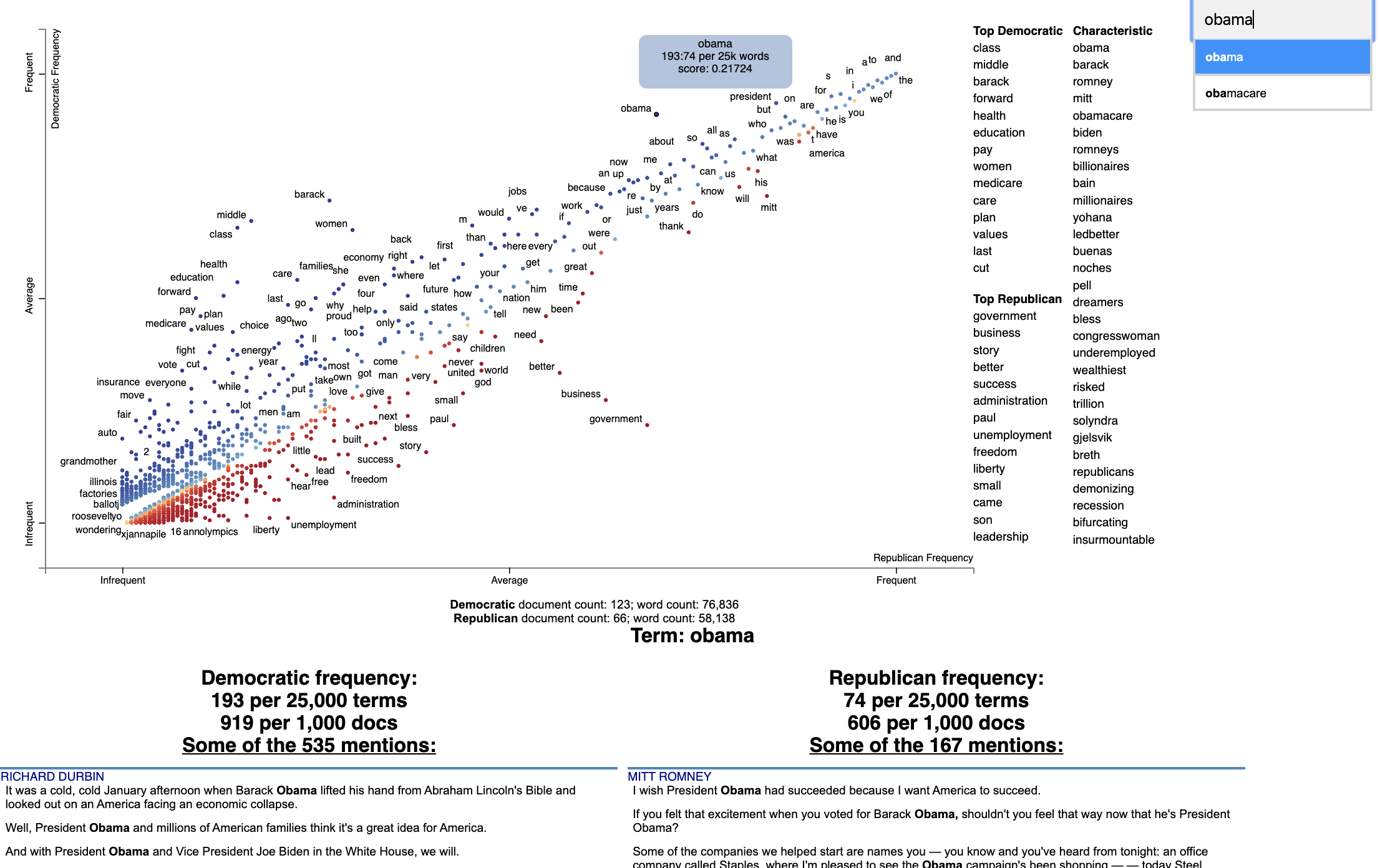## Citation

Jason S. Kessler. Scattertext: a Browser-Based Tool for Visualizing how Corpora Differ. ACL System Demonstrations. 2017. Link to paper: arxiv.org/abs/1703.00565

@article{kessler2017scattertext,
author    = {Kessler, Jason S.},
title     = {Scattertext: a Browser-Based Tool for Visualizing how Corpora Differ},
booktitle = {Proceedings of ACL-2017 System Demonstrations},
year      = {2017},
publisher = {Association for Computational Linguistics},
}


## Installation

Install Python 3.4 or higher and run:

$pip install scattertext If you cannot (or don't want to) install spaCy, substitute nlp = spacy.load('en') lines with nlp = scattertext.WhitespaceNLP.whitespace_nlp. Note, this is not compatible with word_similarity_explorer, and the tokenization and sentence boundary detection capabilities will be low-performance regular expressions. See demo_without_spacy.py for an example. It is recommended you install jieba, spacy, empath, astropy, flashtext, gensim and umap-learn in order to take full advantage of Scattertext. Scattertext should mostly work with Python 2.7, but it may not. The HTML outputs look best in Chrome and Safari. ## Style Guide The name of this project is Scattertext. "Scattertext" is written as a single word and should be capitalized. When used in Python, the package scattertext should be defined to the name st, i.e., import scattertext as st. ## Overview This is a tool that's intended for visualizing what words and phrases are more characteristic of a category than others. Consider the example at the top of the page. Looking at this seem overwhelming. In fact, it's a relatively simple visualization of word use during the 2012 political convention. Each dot corresponds to a word or phrase mentioned by Republicans or Democrats during their conventions. The closer a dot is to the top of the plot, the more frequently it was used by Democrats. The further right a dot, the more that word or phrase was used by Republicans. Words frequently used by both parties, like "of" and "the" and even "Mitt" tend to occur in the upper-right-hand corner. Although very low frequency words have been hidden to preserve computing resources, a word that neither party used, like "giraffe" would be in the bottom-left-hand corner. The interesting things happen close to the upper-left and lower-right corners. In the upper-left corner, words like "auto" (as in auto bailout) and "millionaires" are frequently used by Democrats but infrequently or never used by Republicans. Likewise, terms frequently used by Republicans and infrequently by Democrats occupy the bottom-right corner. These include "big government" and "olympics", referring to the Salt Lake City Olympics in which Gov. Romney was involved. Terms are colored by their association. Those that are more associated with Democrats are blue, and those more associated with Republicans red. Terms that are most characteristic of the both sets of documents are displayed on the far-right of the visualization. The inspiration for this visualization came from Dataclysm (Rudder, 2014). Scattertext is designed to help you build these graphs and efficiently label points on them. The documentation (including this readme) is a work in progress. Please see the tutorial below as well as the PyData 2017 Tutorial. Poking around the code and tests should give you a good idea of how things work. The library covers some novel and effective term-importance formulas, including Scaled F-Score. ## Customizing the Visualization and Plotting Dispersion New in Scattertext 0.1.0, one can use a dataframe for term/metadata positions and other term-specific data. We can also use it to determine term-specific information which is shown after a term is clicked. Note that it is possible to disable the use of document categories in Scattertext, as we shall see in this example. This example covers plotting term dispersion against word frequency and identifying the terms which are most and least dispersed given their frequencies. Using the Rosengren's S dispersion measure (Gries 2021), terms tend to increase in their dispersion scores as they get more frequent. We'll see how we can both plot this effect and factor out the effect of frequency. This, along with a number of other dispersion metrics presented in Gries (2021), are available and documented in the Dispersion class, which we'll use later in the section. Let's start by creating a Convention corpus, but we'll use the CorpusWithoutCategoriesFromParsedDocuments factory to ensure that no categories are included in the corpus. If we try to find document categories, we'll see that all documents have the category '_'. import scattertext as st df = st.SampleCorpora.ConventionData2012.get_data().assign( parse=lambda df: df.text.apply(st.whitespace_nlp_with_sentences)) corpus = st.CorpusWithoutCategoriesFromParsedDocuments( df, parsed_col='parse' ).build().get_unigram_corpus().remove_infrequent_words(minimum_term_count=6) corpus.get_categories() # Returns ['_']  Next, we'll create a dataframe for all terms we'll plot. We'll just start by creating a dataframe where we capture the frequency of each term and various dispersion metrics. These will be shown after a term is activated in the plot. dispersion = st.Dispersion(corpus) dispersion_df = dispersion.get_df() dispersion_df.head(3)  Which returns  Frequency Range SD VC Juilland's D Rosengren's S DP DP norm KL-divergence thank 363 134 3.108113 1.618274 0.707416 0.694898 0.391548 0.391560 0.748808 you 1630 177 12.383708 1.435902 0.888596 0.898805 0.233627 0.233635 0.263337 so 549 155 3.523380 1.212967 0.774299 0.822244 0.283151 0.283160 0.411750  These are discussed in detail in Gries 2021. We'll use Rosengren's S to find the dispersion of each term. It's which a metric designed for corpus parts (convention speeches in our case) of varying length. Where n is the number of documents in the corpus, s_i is the percentage of tokens in the corpus found in document i, v_i is term count in document i, and f is the total number of tokens in the corpus of type term type. In order to start plotting, we'll need to add coordinates for each term to the data frame. To use the dataframe_scattertext function, you need, at a minimum a dataframe with 'X' and 'Y' columns. The Xpos and Ypos columns indicate the positions of the original X and Y values on the scatterplot, and need to be between 0 and 1. Functions in st.Scalers perform this scaling. Absent Xpos or Ypos, st.Scalers.scale would be used. Here is a sample of values: • st.Scalers.scale(vec) Rescales the vector to where the minimum value is 0 and the maximum is 1. • st.Scalers.log_scale(vec) Rescales the lgo of the vector • st.Scalers.dense_ranke(vec) Rescales the dense rank of the vector • st.Scalers.scale_center_zero_abs(vec) Rescales a vector with both positive and negative values such that the 0 value in the original vector is plotted at 0.5, negative values are projected from [-argmax(abs(vec)), 0] to [0, 0.5] and positive values projected from [0, argmax(abs(vec))] to [0.5, 1]. dispersion_df = dispersion_df.assign( X=lambda df: df.Frequency, Xpos=lambda df: st.Scalers.log_scale(df.X), Y=lambda df: df["Rosengren's S"], Ypos=lambda df: st.Scalers.scale(df.Y), )  Note that the Ypos column here is not necessary since Y would automatically be scaled. Finally, since we are not distinguishing between categories, we can set ignore_categories=True. We can now plot this graph using the dataframe_scattertext function: html = st.dataframe_scattertext( corpus, plot_df=dispersion_df, metadata=corpus.get_df()['speaker'] + ' (' + corpus.get_df()['party'].str.upper() + ')', ignore_categories=True, x_label='Log Frequency', y_label="Rosengren's S", y_axis_labels=['Less Dispersion', 'Medium', 'More Dispersion'], )  Which yields (click for an interactive version):Note that we can see various dispersion statistics under a term's name, in addition to the standard usage statistics. To customize the statistics which are displayed, set the term_description_column=[...] parameter with a list of column names to be displayed. One issue in this dispersion chart, which tends to be common to dispersion metrics in general, is that dispersion and frequency tend to have a high correlation, but with a complex, non-linear curve. Depending on the metric, this correlation curve could be power, linear, sigmoidal, or typically, something else. In order to factor out this correlation, we can predict the dispersion from frequency using a non-parametric regressor, and see which terms have the highest and lowest residuals with respect to their expected dispersions based on their frequencies. In this case, we'll use a KNN regressor with 10 neighbors to predict Rosengren'S from term frequencies (dispersion_df.X and .Y respectively), and compute the residual. We'll the residual to color points, with a neutral color for residuals around 0 and other colors for positive and negative values. We'll add a column in the data frame for point colors, and call it ColorScore. It is populated with values between 0 and 1, with 0.5 as a netural color on the d3 interpolateWarm color scale. We use st.Scalers.scale_center_zero_abs, discussed above, to make this transformation. from sklearn.neighbors import KNeighborsRegressor dispersion_df = dispersion_df.assign( Expected=lambda df: KNeighborsRegressor(n_neighbors=10).fit( df.X.values.reshape(-1, 1), df.Y ).predict(df.X.values.reshape(-1, 1)), Residual=lambda df: df.Y - df.Expected, ColorScore=lambda df: st.Scalers.scale_center_zero_abs(df.Residual) )  Now we are ready to plot our colored dispersion chart. We assign the ColorScore column name to the color_score_column paramter in dataframe_scattertext. Additionally, We'd like to populate the two term lists on the left with terms that have high and low residual values, indicating terms which have the most dispersion relative to their frequency-expected level and the lowest. We can do this by the left_list_column parameter. We can specify the upper and lower term list names using the header_names parameter. Finally, we can spiff-up the plot by adding an appealing background color. html = st.dataframe_scattertext( corpus, plot_df=dispersion_df, metadata=corpus.get_df()['speaker'] + ' (' + corpus.get_df()['party'].str.upper() + ')', ignore_categories=True, x_label='Log Frequency', y_label="Rosengren's S", y_axis_labels=['Less Dispersion', 'Medium', 'More Dispersion'], color_score_column='ColorScore', header_names={'upper': 'Lower than Expected', 'lower': 'More than Expected'}, left_list_column='Residual', background_color='#e5e5e3' )  Which yields (click for an interactive version):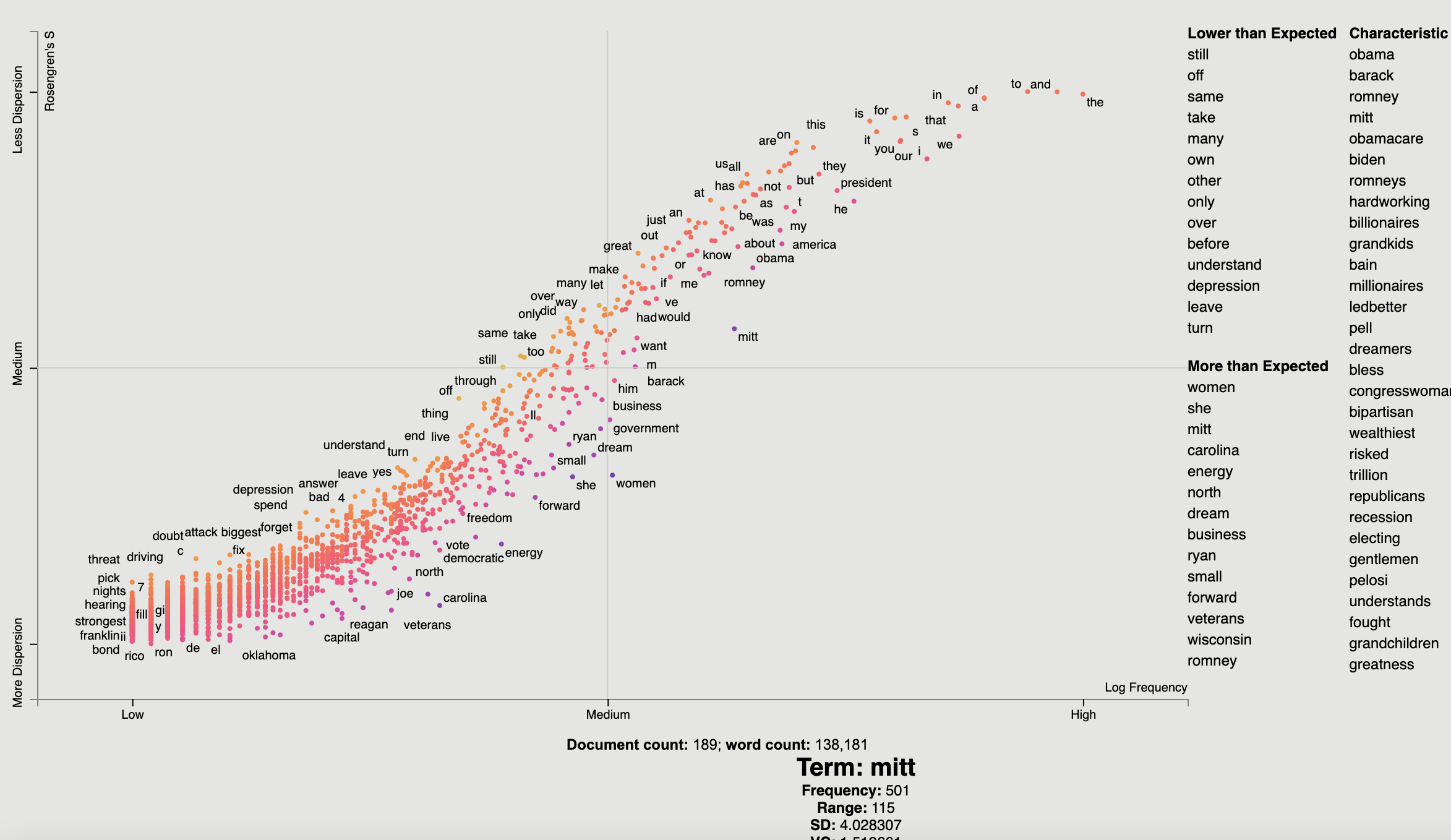## Tutorial ### Help! I don't know Python but I still want to use Scattertext. While you should learn Python fully use Scattertext, I've put some of the basic functionality in a commandline tool. The tool is installed when you follow the procedure laid out above. Run $ scattertext --help from the commandline to see the full usage information. Here's a quick example of how to use vanilla Scattertext on a CSV file. The file needs to have at least two columns, one containing the text to be analyzed, and another containing the category. In the example CSV below, the columns are text and party, respectively.

The example below processes the CSV file, and the resulting HTML visualization into cli_demo.html.

Note, the parameter --minimum_term_frequency=8 omit terms that occur less than 8 times, and --regex_parser indicates a simple regular expression parser should be used in place of spaCy. The flag --one_use_per_doc indicates that term frequency should be calculated by only counting no more than one occurrence of a term in a document.

If you'd like to parse non-English text, you can use the --spacy_language_model argument to configure which spaCy language model the tool will use. The default is 'en' and you can see the others available at https://spacy.io/docs/api/language-models.

$curl -s https://cdn.rawgit.com/JasonKessler/scattertext/master/scattertext/data/political_data.csv | head -2 party,speaker,text democrat,BARACK OBAMA,"Thank you. Thank you. Thank you. Thank you so much.Thank you.Thank you so much. Thank you. Thank you very much, everybody. Thank you.$
$scattertext --datafile=https://cdn.rawgit.com/JasonKessler/scattertext/master/scattertext/data/political_data.csv \ > --text_column=text --category_column=party --metadata_column=speaker --positive_category=democrat \ > --category_display_name=Democratic --not_category_display_name=Republican --minimum_term_frequency=8 \ > --one_use_per_doc --regex_parser --outputfile=cli_demo.html  ### Using Scattertext as a text analysis library: finding characteristic terms and their associations The following code creates a stand-alone HTML file that analyzes words used by Democrats and Republicans in the 2012 party conventions, and outputs some notable term associations. First, import Scattertext and spaCy. >>> import scattertext as st >>> import spacy >>> from pprint import pprint  Next, assemble the data you want to analyze into a Pandas data frame. It should have at least two columns, the text you'd like to analyze, and the category you'd like to study. Here, the text column contains convention speeches while the party column contains the party of the speaker. We'll eventually use the speaker column to label snippets in the visualization. >>> convention_df = st.SampleCorpora.ConventionData2012.get_data() >>> convention_df.iloc party democrat speaker BARACK OBAMA text Thank you. Thank you. Thank you. Thank you so ... Name: 0, dtype: object  Turn the data frame into a Scattertext Corpus to begin analyzing it. To look for differences in parties, set the category_col parameter to 'party', and use the speeches, present in the text column, as the texts to analyze by setting the text col parameter. Finally, pass a spaCy model in to the nlp argument and call build() to construct the corpus. # Turn it into a Scattertext Corpus >>> nlp = spacy.load('en') >>> corpus = st.CorpusFromPandas(convention_df, ... category_col='party', ... text_col='text', ... nlp=nlp).build()  Let's see characteristic terms in the corpus, and terms that are most associated Democrats and Republicans. See slides 52 to 59 of the Turning Unstructured Content ot Kernels of Ideas talk for more details on these approaches. Here are the terms that differentiate the corpus from a general English corpus. >>> print(list(corpus.get_scaled_f_scores_vs_background().index[:10])) ['obama', 'romney', 'barack', 'mitt', 'obamacare', 'biden', 'romneys', 'hardworking', 'bailouts', 'autoworkers']  Here are the terms that are most associated with Democrats: >>> term_freq_df = corpus.get_term_freq_df() >>> term_freq_df['Democratic Score'] = corpus.get_scaled_f_scores('democrat') >>> pprint(list(term_freq_df.sort_values(by='Democratic Score', ascending=False).index[:10])) ['auto', 'america forward', 'auto industry', 'insurance companies', 'pell', 'last week', 'pell grants', "women 's", 'platform', 'millionaires']  And Republicans: >>> term_freq_df['Republican Score'] = corpus.get_scaled_f_scores('republican') >>> pprint(list(term_freq_df.sort_values(by='Republican Score', ascending=False).index[:10])) ['big government', "n't build", 'mitt was', 'the constitution', 'he wanted', 'hands that', 'of mitt', '16 trillion', 'turned around', 'in florida']  ### Visualizing term associations Now, let's write the scatter plot a stand-alone HTML file. We'll make the y-axis category "democrat", and name the category "Democrat" with a capital "D" for presentation purposes. We'll name the other category "Republican" with a capital "R". All documents in the corpus without the category "democrat" will be considered Republican. We set the width of the visualization in pixels, and label each excerpt with the speaker using the metadata parameter. Finally, we write the visualization to an HTML file. >>> html = st.produce_scattertext_explorer(corpus, ... category='democrat', ... category_name='Democratic', ... not_category_name='Republican', ... width_in_pixels=1000, ... metadata=convention_df['speaker']) >>> open("Convention-Visualization.html", 'wb').write(html.encode('utf-8'))  Below is what the webpage looks like. Click it and wait a few minutes for the interactive version.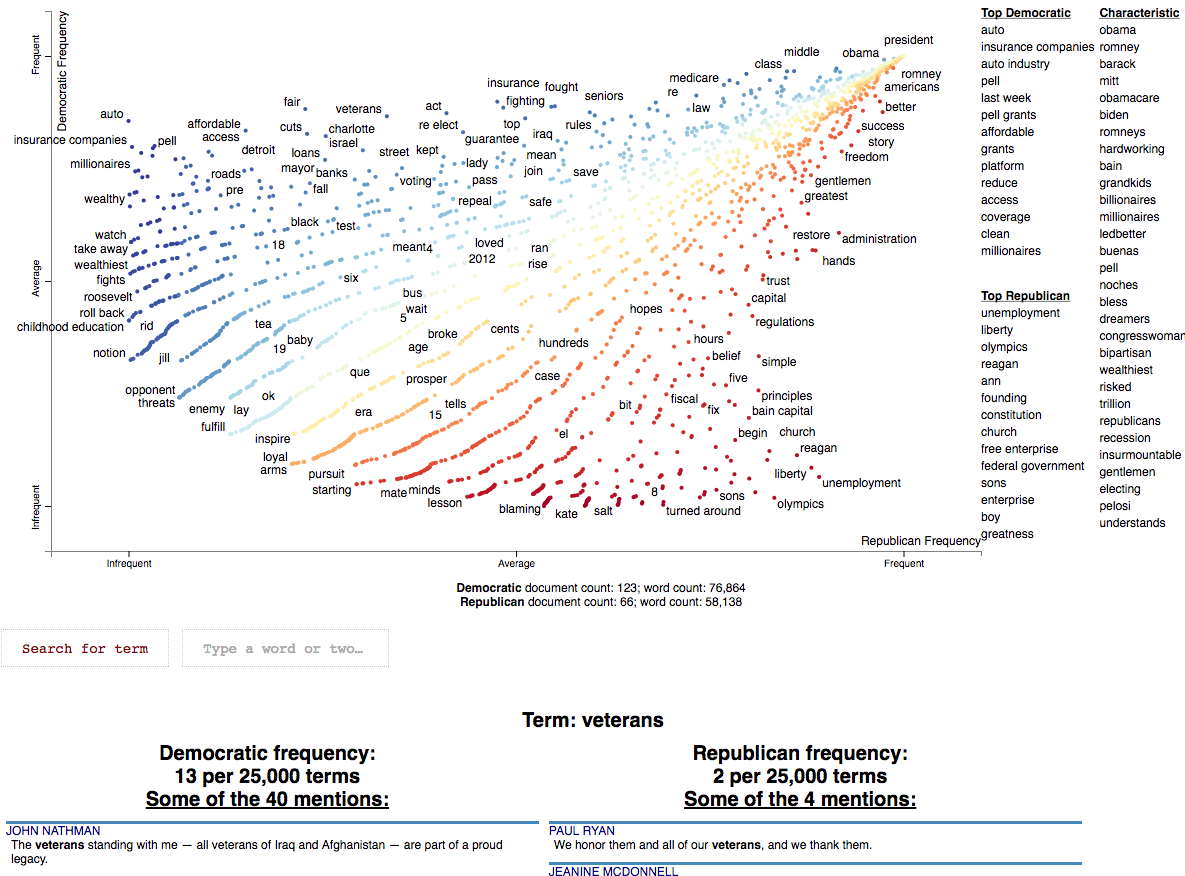### Visualizing Phrase associations Scattertext can also be used to visualize the category association of a variety of different phrase types. The word "phrase" denotes any single or multi-word collocation. #### Using PyTextRank PyTextRank, created by Paco Nathan, is an implementation of a modified version of the TextRank algorithm (Mihalcea and Tarau 2004). It involves graph centrality algorithm to extract a scored list of the most prominent phrases in a document. Here, named entities recognized by spaCy. As of spaCy version 2.2, these are from an NER system trained on Ontonotes 5. Please install pytextrank $ pip3 install pytextrank before continuing with this tutorial.

To use, build a corpus as normal, but make sure you use spaCy to parse each document as opposed a built-in whitespace_nlp-type tokenizer. Note that adding PyTextRank to the spaCy pipeline is not needed, as it will be run separately by the PyTextRankPhrases object. We'll reduce the number of phrases displayed in the chart to 2000 using the AssociationCompactor. The phrases generated will be treated like non-textual features since their document scores will not correspond to word counts.

import pytextrank, spacy
import scattertext as st

convention_df = st.SampleCorpora.ConventionData2012.get_data().assign(
parse=lambda df: df.text.apply(nlp),
party=lambda df: df.party.apply({'democrat': 'Democratic', 'republican': 'Republican'}.get)
)
corpus = st.CorpusFromParsedDocuments(
convention_df,
category_col='party',
parsed_col='parse',
feats_from_spacy_doc=st.PyTextRankPhrases()
).build(
).compact(
AssociationCompactor(2000, use_non_text_features=True)
)


Note that the terms present in the corpus are named entities, and, as opposed to frequency counts, their scores are the eigencentrality scores assigned to them by the TextRank algorithm. Running corpus.get_metadata_freq_df('') will return, for each category, the sums of terms' TextRank scores. The dense ranks of these scores will be used to construct the scatter plot.

term_category_scores = corpus.get_metadata_freq_df('')
print(term_category_scores)
'''
Democratic  Republican
term
our future                                 1.113434    0.699103
their home                                 0.385925    0.000000
our government                             0.185483    0.462122
our workers                                0.199704    0.210989
her family                                 0.540887    0.405552
our time                                   0.510930    0.410058
...
'''


Before we construct the plot, let's some helper variables Since the aggregate TextRank scores aren't particularly interpretable, we'll display the per-category rank of each score in the metadata_description field. These will be displayed after a term is clicked.

term_ranks = np.argsort(np.argsort(-term_category_scores, axis=0), axis=0) + 1
term: '<br/>' + '<br/>'.join(
'<b>%s</b> TextRank score rank: %s/%s' % (cat, term_ranks.loc[term, cat], corpus.get_num_metadata())
for cat in corpus.get_categories())
}


We can construct term scores in a couple ways. One is a standard dense-rank difference, a score which is used in most of the two-category contrastive plots here, which will give us the most category-associated phrases. Another is to use the maximum category-specific score, this will give us the most prominent phrases in each category, regardless of the prominence in the other category. We'll take both approaches in this tutorial, let's compute the second kind of score, the category-specific prominence below.

category_specific_prominence = term_category_scores.apply(
lambda r: r.Democratic if r.Democratic > r.Republican else -r.Republican,
axis=1
)


Now we're ready output this chart. Note that we use a dense_rank transform, which places identically scalled phrases atop each other. We use category_specific_prominence as scores, and set sort_by_dist as False to ensure the phrases displayed on the right-hand side of the chart are ranked by the scores and not distance to the upper-left or lower-right corners. Since matching phrases are treated as non-text features, we encode them as single-phrase topic models and set the topic_model_preview_size to 0 to indicate the topic model list shouldn't be shown. Finally, we set ensure the full documents are displayed. Note the documents will be displayed in order of phrase-specific score.

html = produce_scattertext_explorer(
corpus,
category='Democratic',
not_category_name='Republican',
minimum_term_frequency=0,
pmi_threshold_coefficient=0,
width_in_pixels=1000,
transform=dense_rank,
scores=category_specific_prominence,
sort_by_dist=False,
use_non_text_features=True,
topic_model_term_lists={term: [term] for term in corpus.get_metadata()},
topic_model_preview_size=0,
use_full_doc=True
)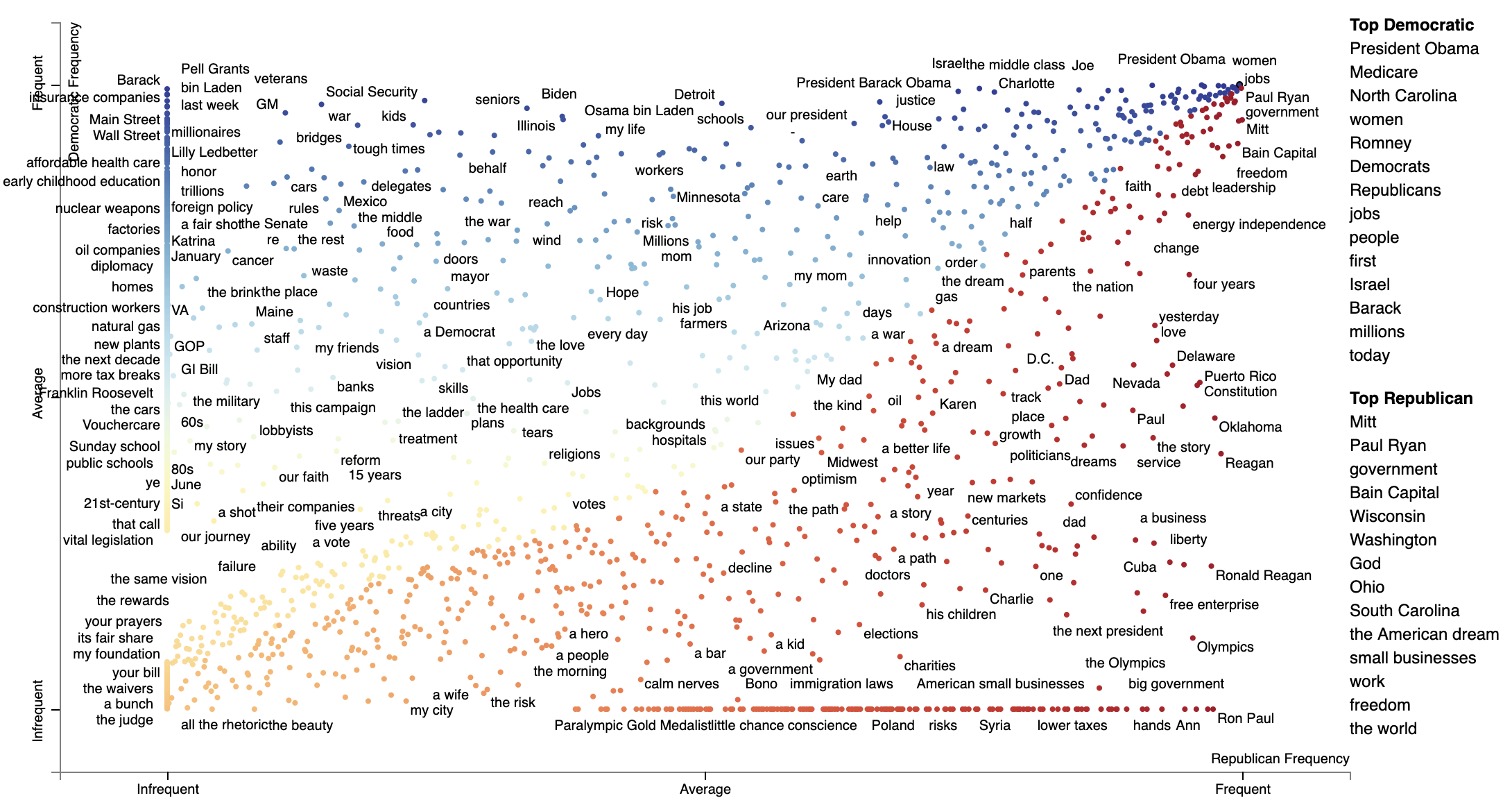The most associated terms in each category make some sense, at least on a post hoc analysis. When referring to (then) Governor Romney, Democrats used his surname "Romney" in their most central mentions of him, while Republicans used the more familiar and humanizing "Mitt". In terms of the President Obama, the phrase "Obama" didn't show up as a top term i n either, the but the first name "Barack" was one of the the most central phrases in Democratic speeches, mirroring "Mitt."

Alternatively, we can Dense Rank Difference in scores to color phrase-points and determine the top phrases to be displayed on the right-hand side of the chart. Instead of setting scores as category-specific prominence scores, we set term_scorer=RankDifference() to inject a way determining term scores into the scatter plot creation process.

html = produce_scattertext_explorer(
corpus,
category='Democratic',
not_category_name='Republican',
minimum_term_frequency=0,
pmi_threshold_coefficient=0,
width_in_pixels=1000,
transform=dense_rank,
use_non_text_features=True,
term_scorer=RankDifference(),
sort_by_dist=False,
topic_model_term_lists={term: [term] for term in corpus.get_metadata()},
topic_model_preview_size=0,
use_full_doc=True
)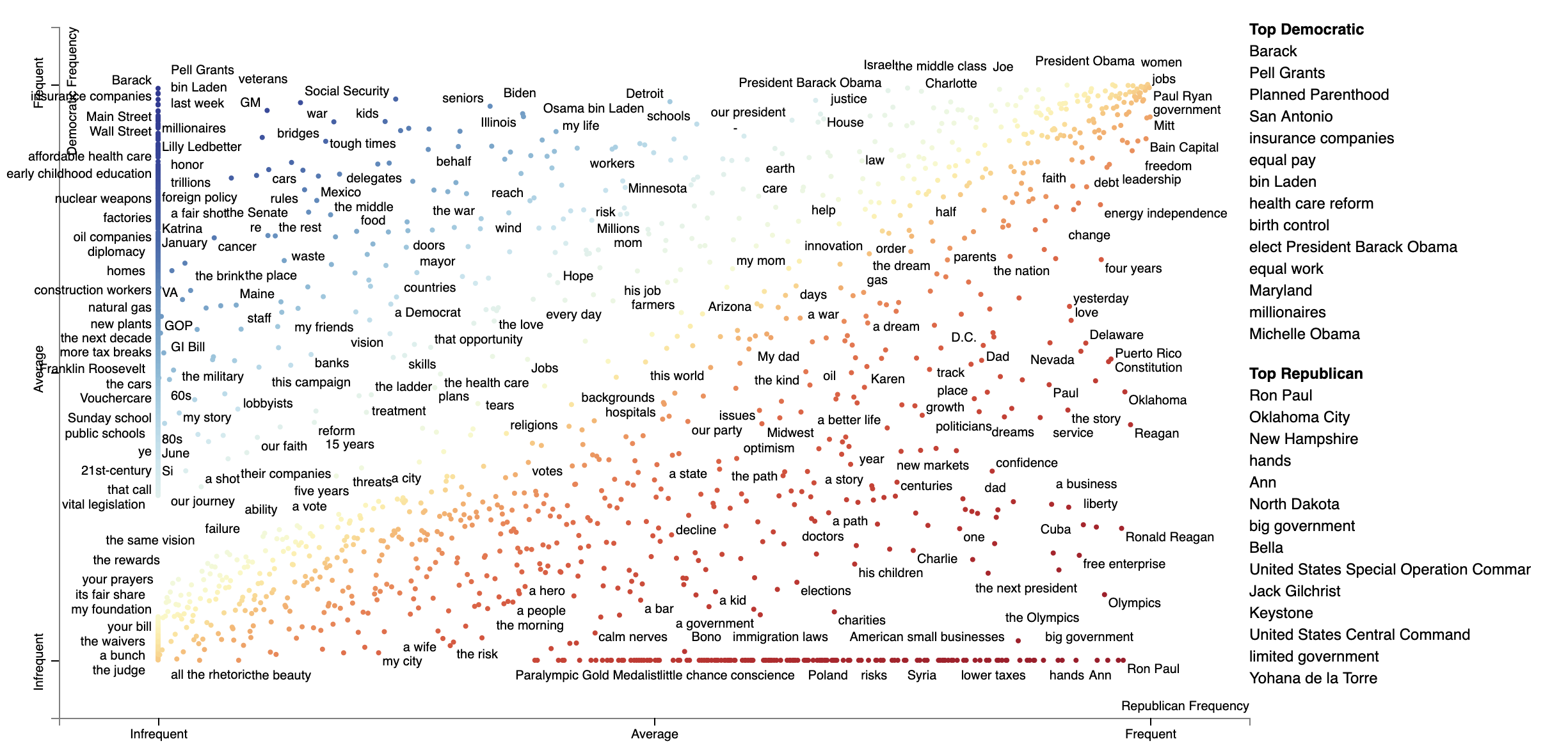#### Using Phrasemachine to find phrases.

Phrasemachine from AbeHandler (Handler et al. 2016) uses regular expressions over sequences of part-of-speech tags to identify noun phrases. This has the advantage over using spaCy's NP-chunking in that it tends to isolote meaningful, large noun phases which are free of appositives.

A opposed to PyTextRank, we'll just use counts of these phrases, treating them like any other term.

import spacy
from scattertext import SampleCorpora, PhraseMachinePhrases, dense_rank, RankDifference, AssociationCompactor, produce_scattertext_explorer
from scattertext.CorpusFromPandas import CorpusFromPandas

corpus = (CorpusFromPandas(SampleCorpora.ConventionData2012.get_data(),
category_col='party',
text_col='text',
feats_from_spacy_doc=PhraseMachinePhrases(),
.build().compact(AssociationCompactor(4000)))

html = produce_scattertext_explorer(corpus,
category='democrat',
category_name='Democratic',
not_category_name='Republican',
minimum_term_frequency=0,
pmi_threshold_coefficient=0,
transform=dense_rank,
term_scorer=RankDifference(),
width_in_pixels=1000)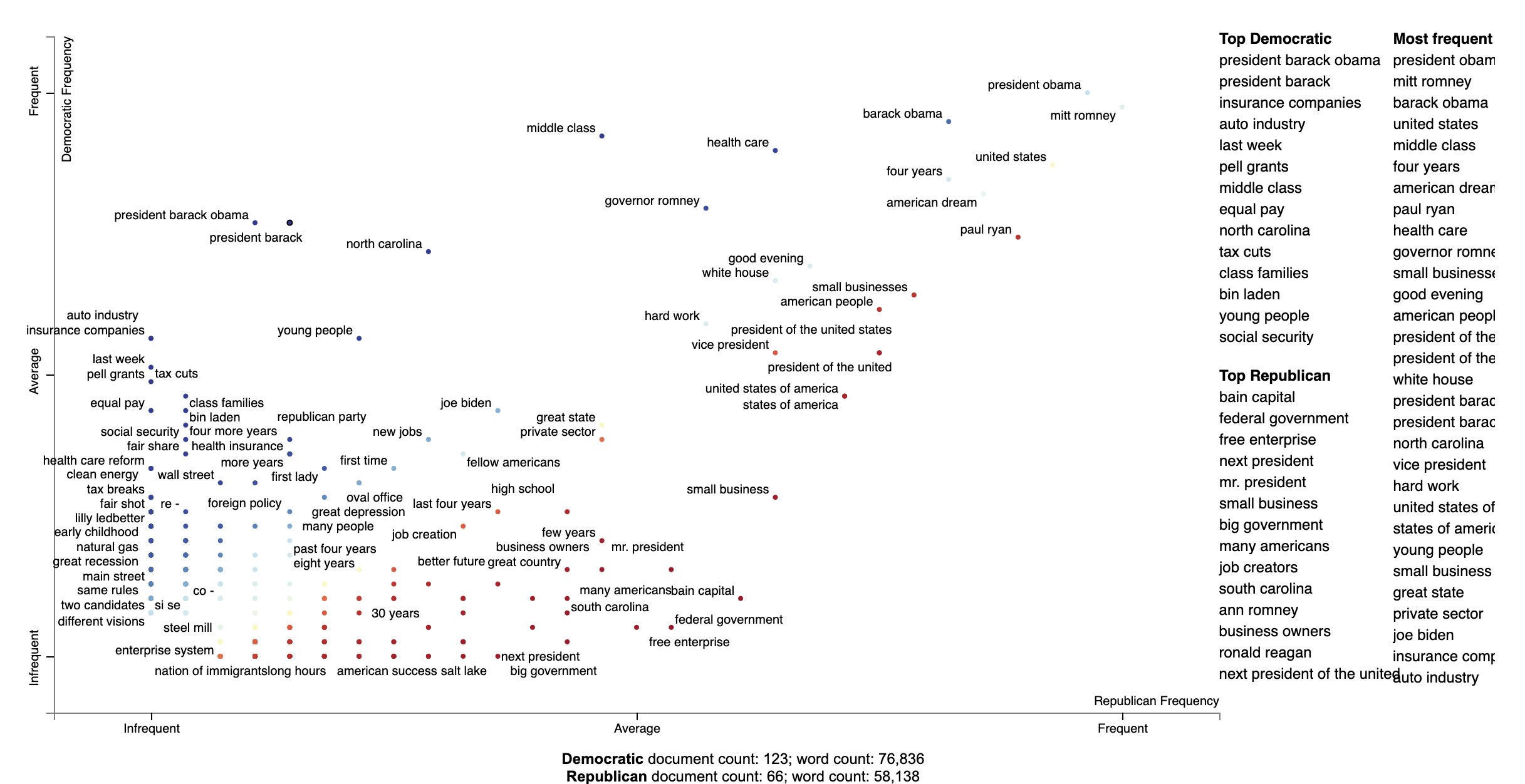### Visualizing Empath topics and categories

In order to visualize Empath (Fast et al., 2016) topics and categories instead of terms, we'll need to create a Corpus of extracted topics and categories rather than unigrams and bigrams. To do so, use the FeatsOnlyFromEmpath feature extractor. See the source code for examples of how to make your own.

When creating the visualization, pass the use_non_text_features=True argument into produce_scattertext_explorer. This will instruct it to use the labeled Empath topics and categories instead of looking for terms. Since the documents returned when a topic or category label is clicked will be in order of the document-level category-association strength, setting use_full_doc=True makes sense, unless you have enormous documents. Otherwise, the first 300 characters will be shown.

(New in 0.0.26). Ensure you include topic_model_term_lists=feat_builder.get_top_model_term_lists() in produce_scattertext_explorer to ensure it bolds passages of snippets that match the topic model.

>>> feat_builder = st.FeatsFromOnlyEmpath()
>>> empath_corpus = st.CorpusFromParsedDocuments(convention_df,
...                                              category_col='party',
...                                              feats_from_spacy_doc=feat_builder,
...                                              parsed_col='text').build()
>>> html = st.produce_scattertext_explorer(empath_corpus,
...                                        category='democrat',
...                                        category_name='Democratic',
...                                        not_category_name='Republican',
...                                        width_in_pixels=1000,
...                                        use_non_text_features=True,
...                                        use_full_doc=True,
...                                        topic_model_term_lists=feat_builder.get_top_model_term_lists())
>>> open("Convention-Visualization-Empath.html", 'wb').write(html.encode('utf-8'))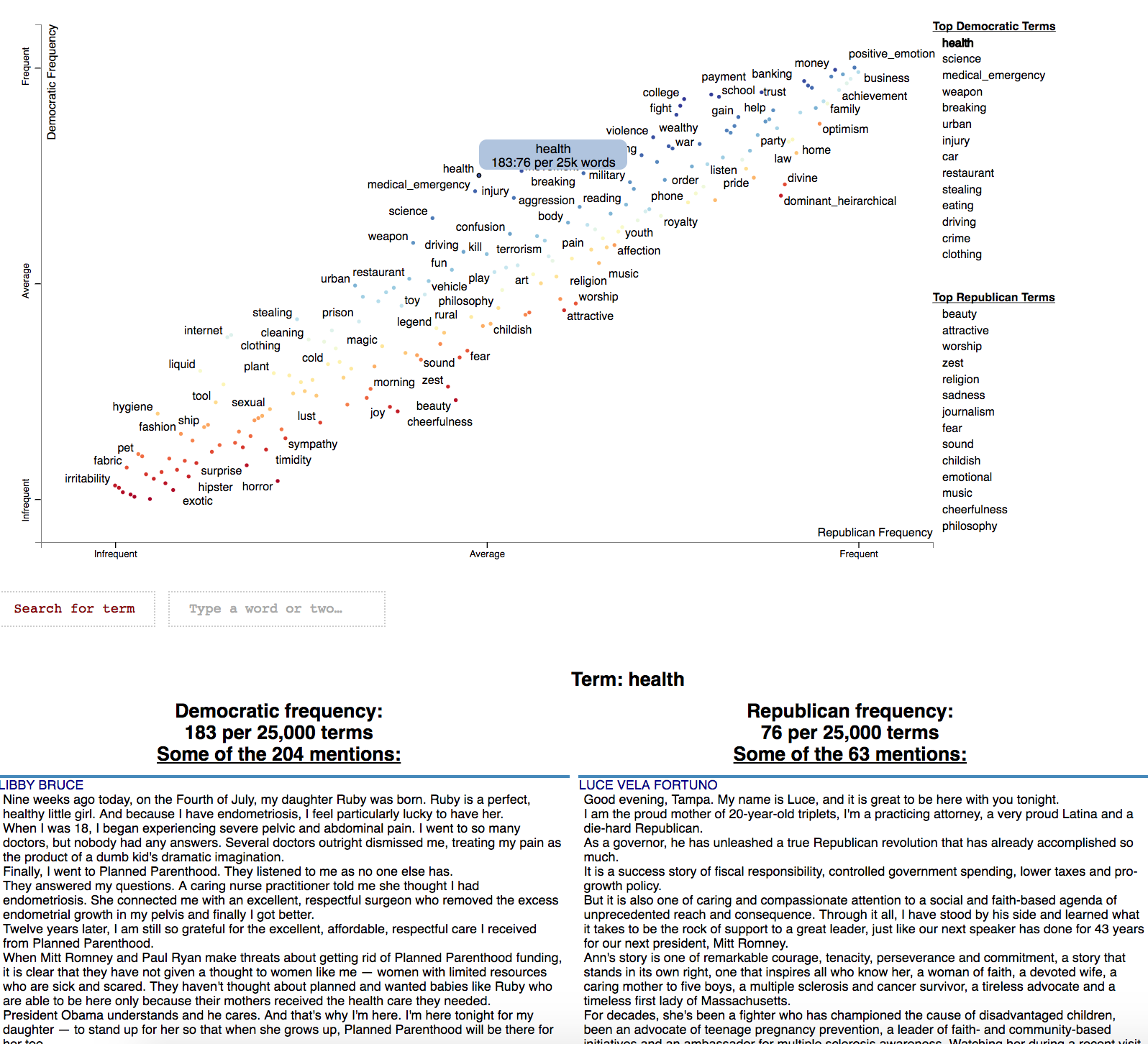### Visualizing General Inquirer Tag Categories and Document Categories

Scattertext also includes a feature builder to explore the relationship between General Inquirer Tag Categoires and Document Categories. We'll use a slightly different approach, looking at relationship of GI Tag Categories to political parties by using the Z-Scores of the Log-Odds-Ratio with Uninformative Dirichlet Priors (Monroe 2008). We'll use the produce_frequency_explorer plot variation to visualize this relationship, setting the x-axis as the number of times a word in the tag category occurs, and the y-axis as the z-score.

We'll use the same data set as before, except we'll use the FeatsFromGeneralInquirer feature builder.

>>> general_inquirer_feature_builder = st.FeatsFromGeneralInquirer()
>>> corpus = st.CorpusFromPandas(convention_df,
...                              category_col='party',
...                              text_col='text',
...                              nlp=st.whitespace_nlp_with_sentences,
...                              feats_from_spacy_doc=general_inquirer_feature_builder).build()


Next, we'll call produce_frequency_explorer in a similar way we called produce_scattertext_explorer in the previous section. There are a few differences, however. First, we specify the LogOddsRatioUninformativeDirichletPrior term scorer, which scores the relationships between the categories. The grey_threshold indicates the points scoring between [-1.96, 1.96] (i.e., p > 0.05) should be colored gray. The argument metadata_descriptions=general_inquirer_feature_builder.get_definitions() indicates that a dictionary mapping the tag name to a string definition is passed. When a tag is clicked, the definition in the dictionary will be shown below the plot, as shown in the image following the snippet.

>>> html = st.produce_frequency_explorer(corpus,
...                                      category='democrat',
...                                      category_name='Democratic',
...                                      not_category_name='Republican',
...                                      use_non_text_features=True,
...                                      use_full_doc=True,
...                                      term_scorer=st.LogOddsRatioUninformativeDirichletPrior(),
...                                      grey_threshold=1.96,
...                                      width_in_pixels=1000,
...                                      topic_model_term_lists=general_inquirer_feature_builder.get_top_model_term_lists(),


Here's the resulting chart.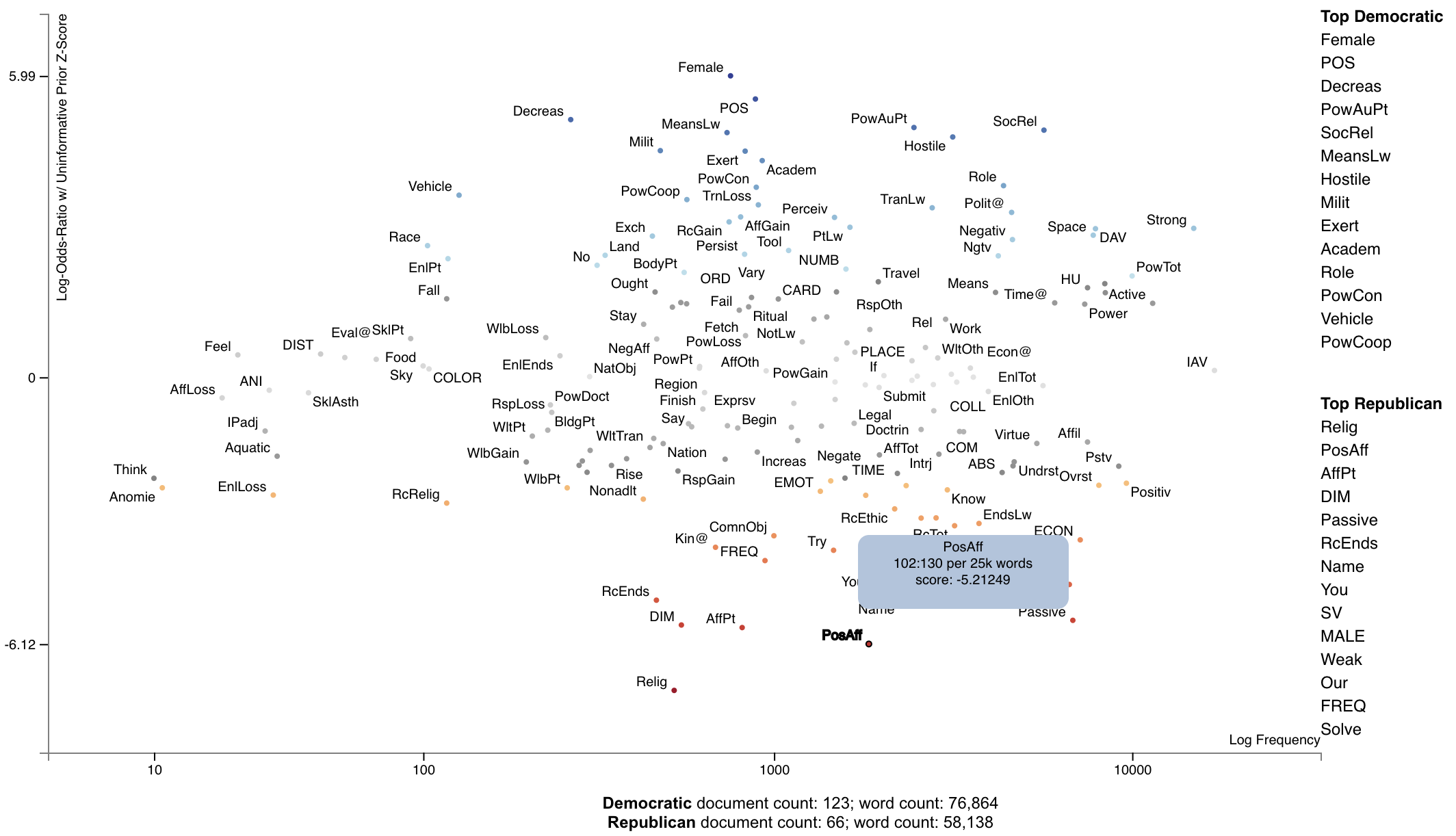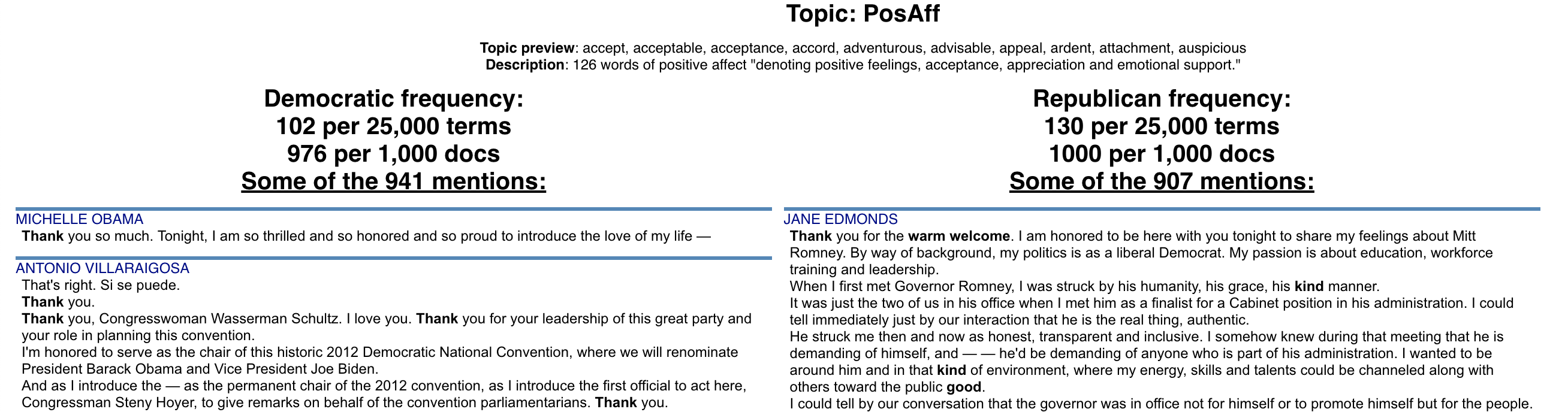### Visualizing the Moral Foundations 2.0 Dictionary

The [Moral Foundations Theory] proposes six psychological constructs as building blocks of moral thinking, as described in Graham et al. (2013). These foundations are, as described on [moralfoundations.org]: care/harm, fairness/cheating, loyalty/betrayal, authority/subversion, sanctity/degradation, and liberty/oppression. Please see the site for a more in-depth discussion of these foundations.

Frimer et al. (2019) created the Moral Foundations Dictionary 2.0, or a lexicon of terms which invoke a moral foundation as a virtue (favorable toward the foundation) or a vice (in opposition to the foundation).

This dictionary can be used in the same way as the General Inquirer. In this example, we can plot the Cohen's d scores of foundation-word counts relative to the frequencies words involving those foundations were invoked.

We can first load the the corpus as normal, and use st.FeatsFromMoralFoundationsDictionary() to extract features.

import scattertext as st

convention_df = st.SampleCorpora.ConventionData2012.get_data()
moral_foundations_feats = st.FeatsFromMoralFoundationsDictionary()
corpus = st.CorpusFromPandas(convention_df,
category_col='party',
text_col='text',
nlp=st.whitespace_nlp_with_sentences,
feats_from_spacy_doc=moral_foundations_feats).build()


Next, let's use Cohen's d term scorer to analyze the corpus, and describe a set of Cohen's d association scores.

cohens_d_scorer = st.CohensD(corpus).use_metadata()
term_scorer = cohens_d_scorer.set_categories('democrat', ['republican']).term_scorer.get_score_df()


Which yields the following data frame:

cohens_d cohens_d_se cohens_d_z cohens_d_p hedges_r hedges_r_se hedges_r_z hedges_r_p m1 m2 count1 count2 docs1 docs2
care.virtue 0.662891 0.149425 4.43629 4.57621e-06 0.660257 0.159049 4.15129 1.65302e-05 0.195049 0.12164 760 379 115 54
care.vice 0.24435 0.146025 1.67335 0.0471292 0.243379 0.152654 1.59432 0.0554325 0.0580005 0.0428358 244 121 80 41
fairness.virtue 0.176794 0.145767 1.21286 0.112592 0.176092 0.152164 1.15725 0.123586 0.0502469 0.0403369 225 107 71 39
fairness.vice 0.0707162 0.145528 0.485928 0.313509 0.0704352 0.151711 0.464273 0.321226 0.00718627 0.00573227 32 14 21 10
authority.virtue -0.0187793 0.145486 -0.12908 0.551353 -0.0187047 0.15163 -0.123357 0.549088 0.358192 0.361191 1281 788 122 66
authority.vice -0.0354164 0.145494 -0.243422 0.596161 -0.0352757 0.151646 -0.232619 0.591971 0.00353465 0.00390602 20 14 14 10
sanctity.virtue -0.512145 0.147848 -3.46399 0.999734 -0.51011 0.156098 -3.26788 0.999458 0.0587987 0.101677 265 309 74 48
sanctity.vice -0.108011 0.145589 -0.74189 0.770923 -0.107582 0.151826 -0.708585 0.760709 0.00845048 0.0109339 35 28 23 20
loyalty.virtue -0.413696 0.147031 -2.81367 0.997551 -0.412052 0.154558 -2.666 0.996162 0.259296 0.309776 1056 717 119 66
loyalty.vice -0.0854683 0.145549 -0.587213 0.72147 -0.0851287 0.151751 -0.560978 0.712594 0.00124518 0.00197022 5 5 5 4

This data frame gives us Cohen's d scores (and their standard errors and z-scores), Hedge's r scores (ditto), the mean document-length normalized topic usage per category (where the in-focus category is m1 [in this case Democrats] and the out-of-focus is m2), the raw number of words used in for each topic (count1 and count2), and the number of documents in each category with the topic (docs1 and docs2).

Note that Cohen's d is the difference of m1 and m2 divided by their pooled standard deviation.

Now, let's plot the d-scores of foundations vs. their frequencies.

html = st.produce_frequency_explorer(
corpus,
category='democrat',
category_name='Democratic',
not_category_name='Republican',
use_non_text_features=True,
use_full_doc=True,
grey_threshold=0,
width_in_pixels=1000,
topic_model_term_lists=moral_foundations_feats.get_top_model_term_lists(),
)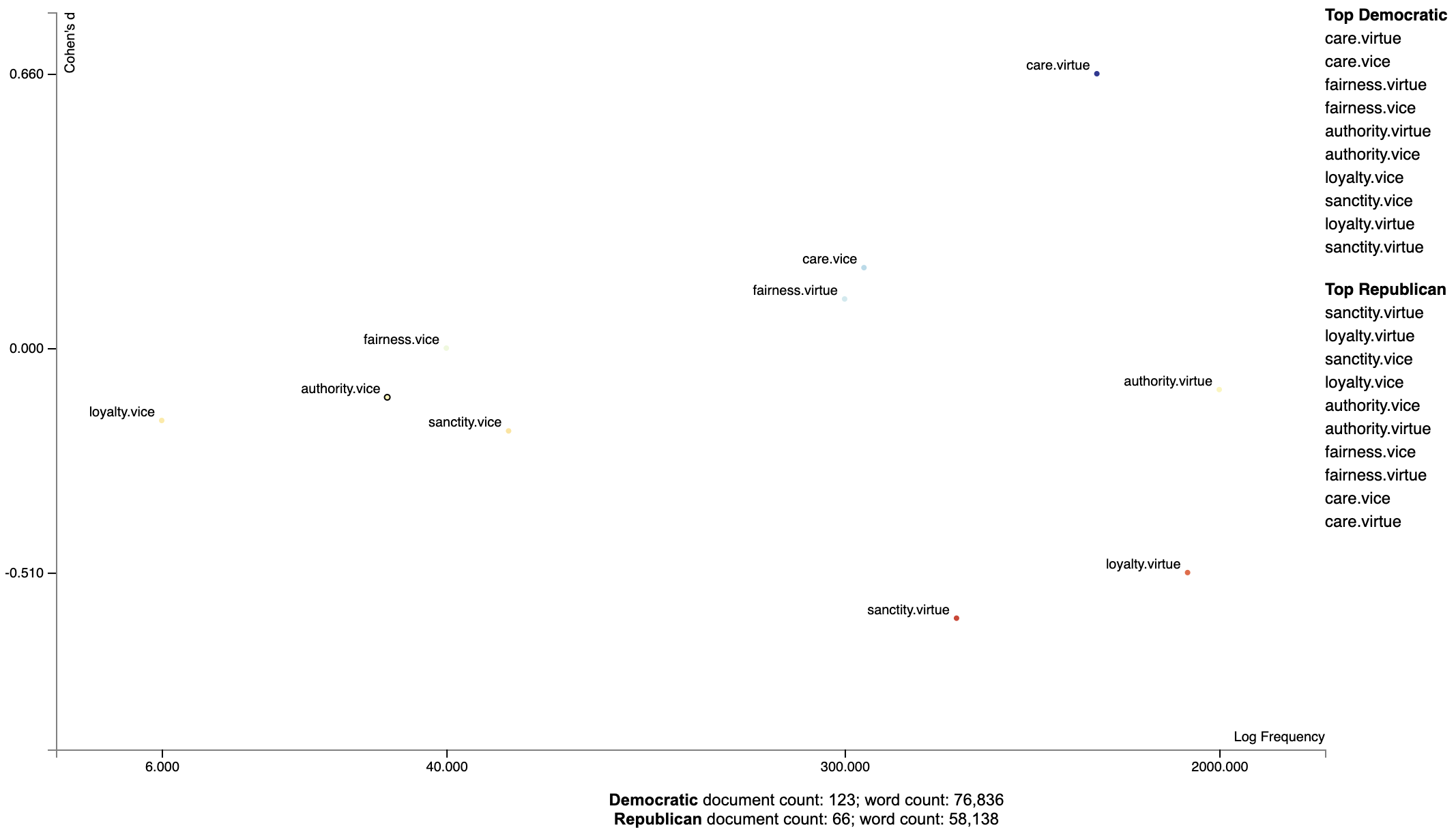### Ordering Terms by Corpus Characteristicness

Often the terms of most interest are ones that are characteristic to the corpus as a whole. These are terms which occur frequently in all sets of documents being studied, but relatively infrequent compared to general term frequencies.

We can produce a plot with a characteristic score on the x-axis and class-association scores on the y-axis using the function produce_characteristic_explorer.

Corpus characteristicness is the difference in dense term ranks between the words in all of the documents in the study and a general English-language frequency list. See this Talk on Term-Class Association Scores for a more thorough explanation.

import scattertext as st

corpus = (st.CorpusFromPandas(st.SampleCorpora.ConventionData2012.get_data(),
category_col='party',
text_col='text',
nlp=st.whitespace_nlp_with_sentences)
.build()
.get_unigram_corpus()
.compact(st.ClassPercentageCompactor(term_count=2,
term_ranker=st.OncePerDocFrequencyRanker)))
html = st.produce_characteristic_explorer(
corpus,
category='democrat',
category_name='Democratic',
not_category_name='Republican',
)
open('demo_characteristic_chart.html', 'wb').write(html.encode('utf-8'))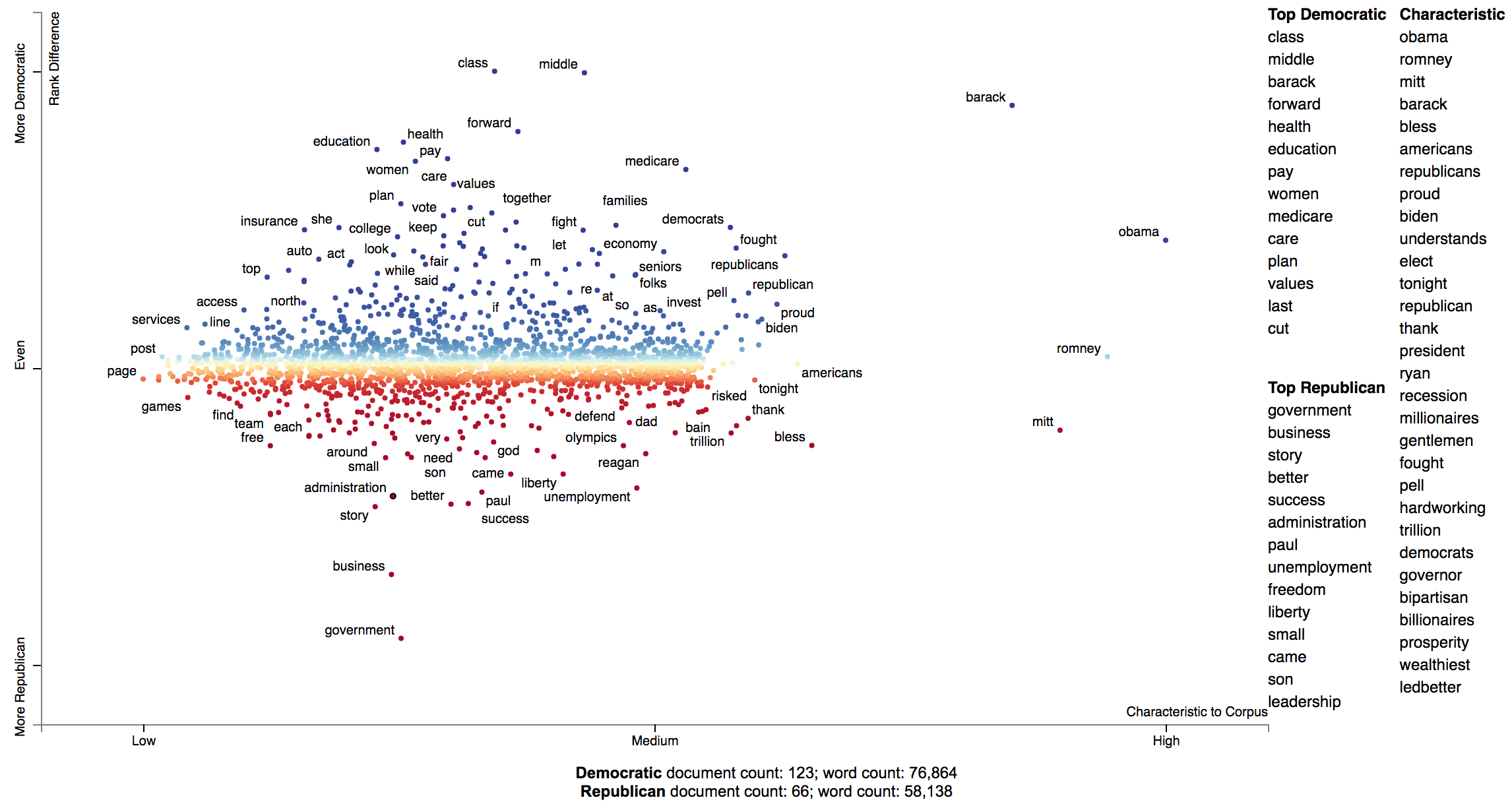### Document-Based Scatterplots

In addition to words, phases and topics, we can make each point correspond to a document. Let's first create a corpus object for the 2012 Conventions data set. This explanation follows demo_pca_documents.py

import pandas as pd
from sklearn.feature_extraction.text import TfidfTransformer
import scattertext as st
from scipy.sparse.linalg import svds

convention_df = st.SampleCorpora.ConventionData2012.get_data()
convention_df['parse'] = convention_df['text'].apply(st.whitespace_nlp_with_sentences)
corpus = (st.CorpusFromParsedDocuments(convention_df,
category_col='party',
parsed_col='parse')
.build()
.get_stoplisted_unigram_corpus())


Next, let's add the document names as meta data in the corpus object. The add_doc_names_as_metadata function takes an array of document names, and populates a new corpus' meta data with those names. If two documents have the same name, it appends a number (starting with 1) to the name.

corpus = corpus.add_doc_names_as_metadata(corpus.get_df()['speaker'])


Next, we find tf.idf scores for the corpus' term-document matrix, run sparse SVD, and add them to a projection data frame, making the x and y-axes the first two singular values, and indexing it on the corpus' meta data, which corresponds to the document names.

embeddings = TfidfTransformer().fit_transform(corpus.get_term_doc_mat())
u, s, vt = svds(embeddings, k=3, maxiter=20000, which='LM')
projection = pd.DataFrame({'term': corpus.get_metadata(), 'x': u.T, 'y': u.T}).set_index('term')


Finally, set scores as 1 for Democrats and 0 for Republicans, rendering Republican documents as red points and Democratic documents as blue. For more on the produce_pca_explorer function, see Using SVD to visualize any kind of word embeddings.

category = 'democrat'
scores = (corpus.get_category_ids() == corpus.get_categories().index(category)).astype(int)
html = st.produce_pca_explorer(corpus,
category=category,
category_name='Democratic',
not_category_name='Republican',
width_in_pixels=1000,
show_axes=False,
use_non_text_features=True,
use_full_doc=True,
projection=projection,
scores=scores,
show_top_terms=False)


Click for an interactive version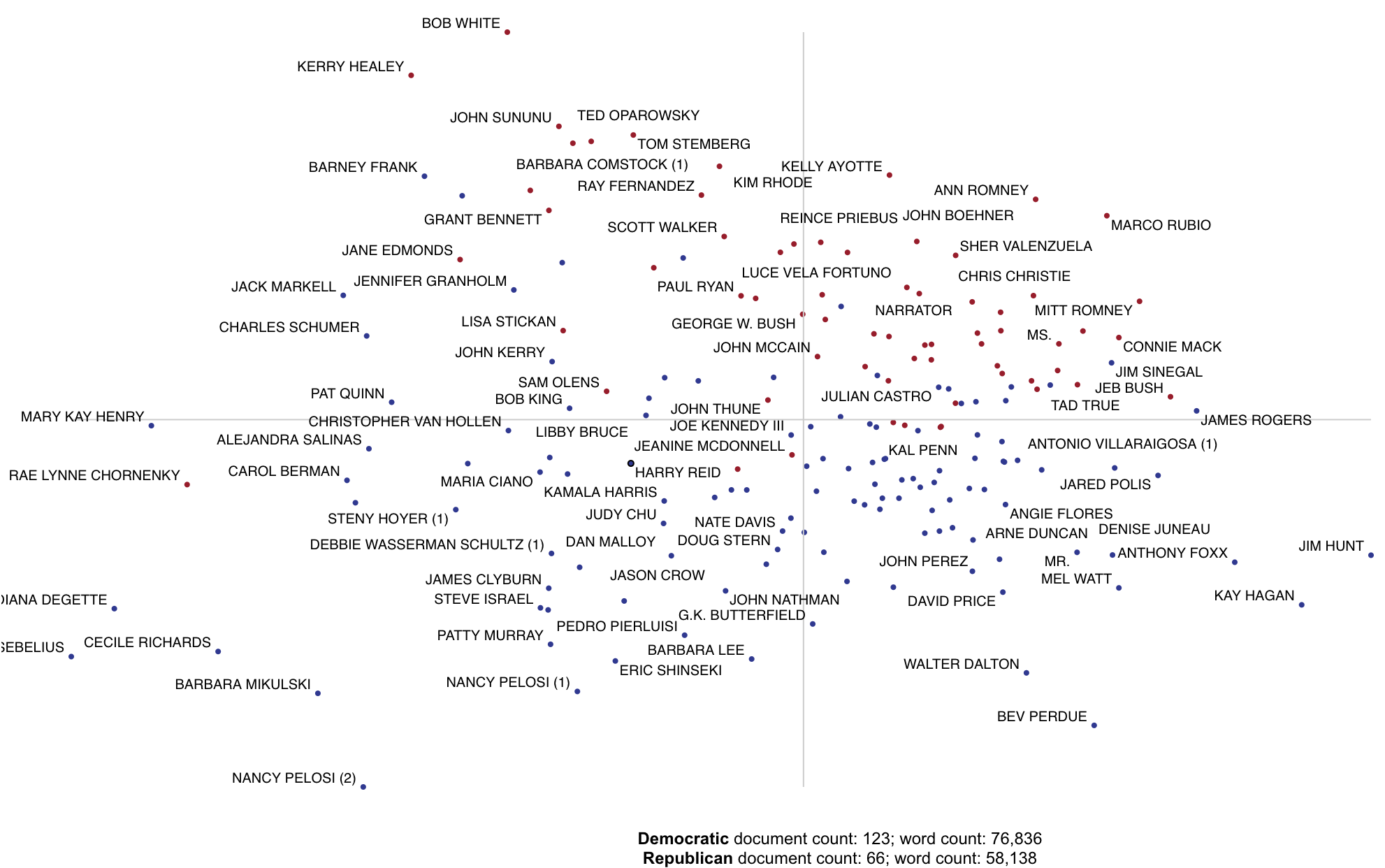### Using Cohen's d or Hedge's r to visualize effect size.

Cohen's d is a popular metric used to measure effect size. The definitions of Cohen's d and Hedge's r from (Shinichi and Cuthill 2017) are implemented in Scattertext.

>>> convention_df = st.SampleCorpora.ConventionData2012.get_data()
>>> corpus = (st.CorpusFromPandas(convention_df,
...                               category_col='party',
...                               text_col='text',
...                               nlp=st.whitespace_nlp_with_sentences)
...           .build()
...           .get_unigram_corpus())


We can create a term scorer object to examine the effect sizes and other metrics.

>>> term_scorer = st.CohensD(corpus).set_categories('democrat', ['republican'])
cohens_d  cohens_d_se  cohens_d_z     cohens_d_p  hedges_r  hedges_r_se  hedges_r_z  hedges_r_p        m1        m2
obama      1.187378     0.024588   48.290444   0.000000e+00  1.187322     0.018419   64.461363         0.0  0.007778  0.002795
class      0.855859     0.020848   41.052045   0.000000e+00  0.855818     0.017227   49.677688         0.0  0.002222  0.000375
middle     0.826895     0.020553   40.232746   0.000000e+00  0.826857     0.017138   48.245626         0.0  0.002316  0.000400
president  0.820825     0.020492   40.056541   0.000000e+00  0.820786     0.017120   47.942661         0.0  0.010231  0.005369
barack     0.730624     0.019616   37.245725  6.213052e-304  0.730589     0.016862   43.327800         0.0  0.002547  0.000725


Our calculation of Cohen's d is not directly based on term counts. Rather, we divide each document's term counts by the total number of terms in the document before calculating the statistics. m1 and m2 are, respectively the mean portions of words in speeches made by Democrats and Republicans that were the term in question. The effect size (cohens_d) is the difference between these means divided by the pooled standard standard deviation. cohens_d_se is the standard error of the statistic, while cohens_d_z and cohens_d_p are the Z-scores and p-values indicating the statistical significance of the effect. Corresponding columns are present for Hedge's r, and unbiased version of Cohen's d.

>>> st.produce_frequency_explorer(
corpus,
category='democrat',
category_name='Democratic',
not_category_name='Republican',
term_scorer=st.CohensD(corpus),
grey_threshold=0
)


Click for an interactive version.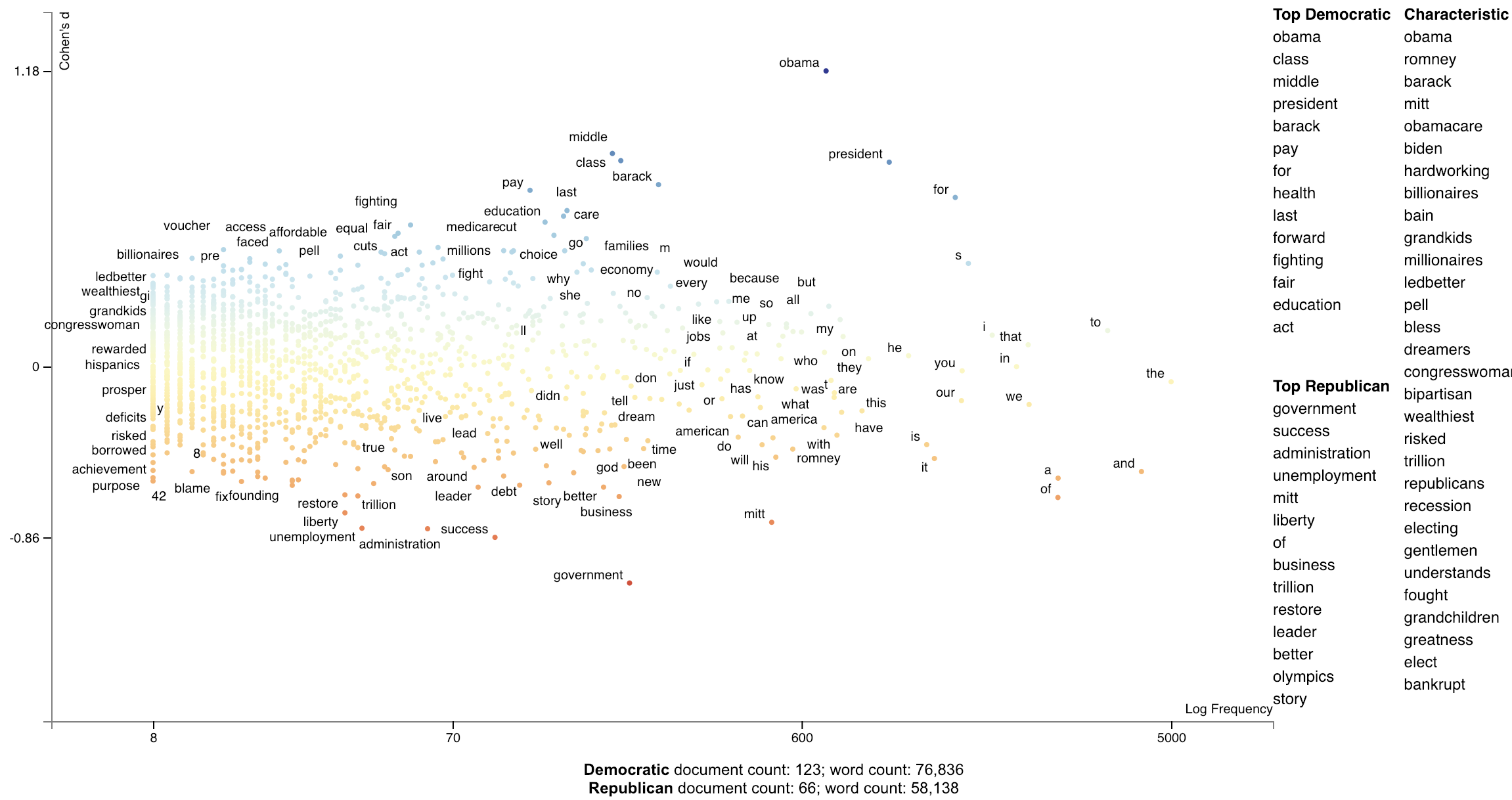### Using Bi-Normal Separation (BNS) to score terms

Bi-Normal Separation (BNS) (Forman, 2008) was added in version 0.1.8. A variation of (BNS) is used where $F^{-1}(tpr) - F^{-1}(fpr)$ is not used as an absolute value, but kept as a difference. This allows for terms strongly indicative of true positives and false positives to have a high or low score. Note that tpr and fpr are scaled to between $[\alpha, 1-\alpha]$ where alpha is $\in [0, 1]$. In Forman (2008) and earlier literature $\alpha=0.0005$. In personal correspondence with Forman, he kindly suggested using $\frac{1.}{\mbox{minimum(positives, negatives)}}$. I have implemented this as $\alpha=\frac{1.}{\mbox{minimum documents in least frequent category}}$

corpus = (st.CorpusFromPandas(convention_df,
category_col='party',
text_col='text',
nlp=st.whitespace_nlp_with_sentences)
.build()
.get_unigram_corpus()
.remove_infrequent_words(3, term_ranker=st.OncePerDocFrequencyRanker))

term_scorer = (st.BNSScorer(corpus).set_categories('democrat'))
print(term_scorer.get_score_df().sort_values(by='democrat BNS'))

html = st.produce_frequency_explorer(
corpus,
category='democrat',
category_name='Democratic',
not_category_name='Republican',
scores=term_scorer.get_score_df()['democrat BNS'].reindex(corpus.get_terms()).values,
minimum_term_frequency=0,
grey_threshold=0,
y_label=f'Bi-normal Separation (alpha={term_scorer.prior_counts})'
)


BNS Scored terms using an algorithmically found alpha.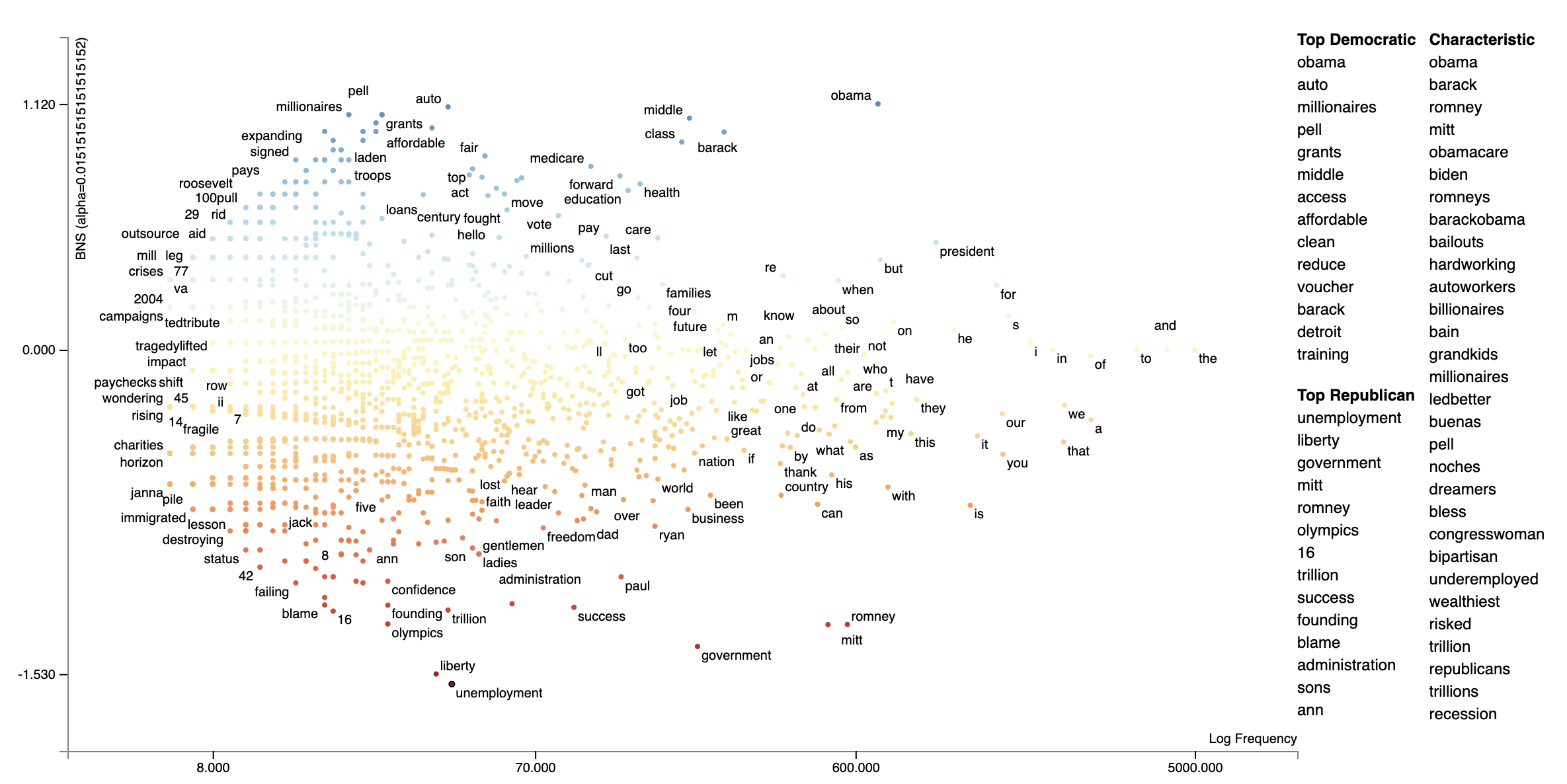### Using correlations to explain classifiers

We can train a classifier to produce a prediction score for each document. Often classifiers or regressors use features which take into account features beyond the ones represented by Scatterext, be they n-gram, topic, extra-linguistic, neural, etc.

We can use Scattertext to visualize the correlations between unigrams (or really any feature representation) and the document scores produced by a model.

In the following example, we train a linear SVM using unigram and bi-gram features on the entire convention data set, and use the model to make a prediction on each document, and finally using Pearson's $r$ to correlate unigram features to the distance from the SVM decision boundary.

from sklearn.svm import LinearSVC

import scattertext as st

df = st.SampleCorpora.ConventionData2012.get_data().assign(
parse=lambda df: df.text.apply(st.whitespace_nlp_with_sentences)
)

corpus = st.CorpusFromParsedDocuments(
df, category_col='party', parsed_col='parse'
).build()

X = corpus.get_term_doc_mat()
y = corpus.get_category_ids()

clf = LinearSVC()
clf.fit(X=X, y=y==corpus.get_categories().index('democrat'))
doc_scores = clf.decision_function(X=X)

compactcorpus = corpus.get_unigram_corpus().compact(st.AssociationCompactor(2000))

plot_df = st.Correlations().set_correlation_type(
'pearsonr'
).get_correlation_df(
corpus=compactcorpus,
document_scores=doc_scores
).reindex(compactcorpus.get_terms()).assign(
X=lambda df: df.Frequency,
Y=lambda df: df['r'],
Xpos=lambda df: st.Scalers.dense_rank(df.X),
Ypos=lambda df: st.Scalers.scale_center_zero_abs(df.Y),
SuppressDisplay=False,
ColorScore=lambda df: df.Ypos,
)

html = st.dataframe_scattertext(
compactcorpus,
plot_df=plot_df,
category='democrat',
category_name='Democratic',
not_category_name='Republican',
width_in_pixels=1000,
unified_context=False,
ignore_categories=False,
color_score_column='ColorScore',
left_list_column='ColorScore',
y_label="Pearson r (correlation to SVM document score)",
x_label='Frequency Ranks',
'lower': 'Top Republican'},
)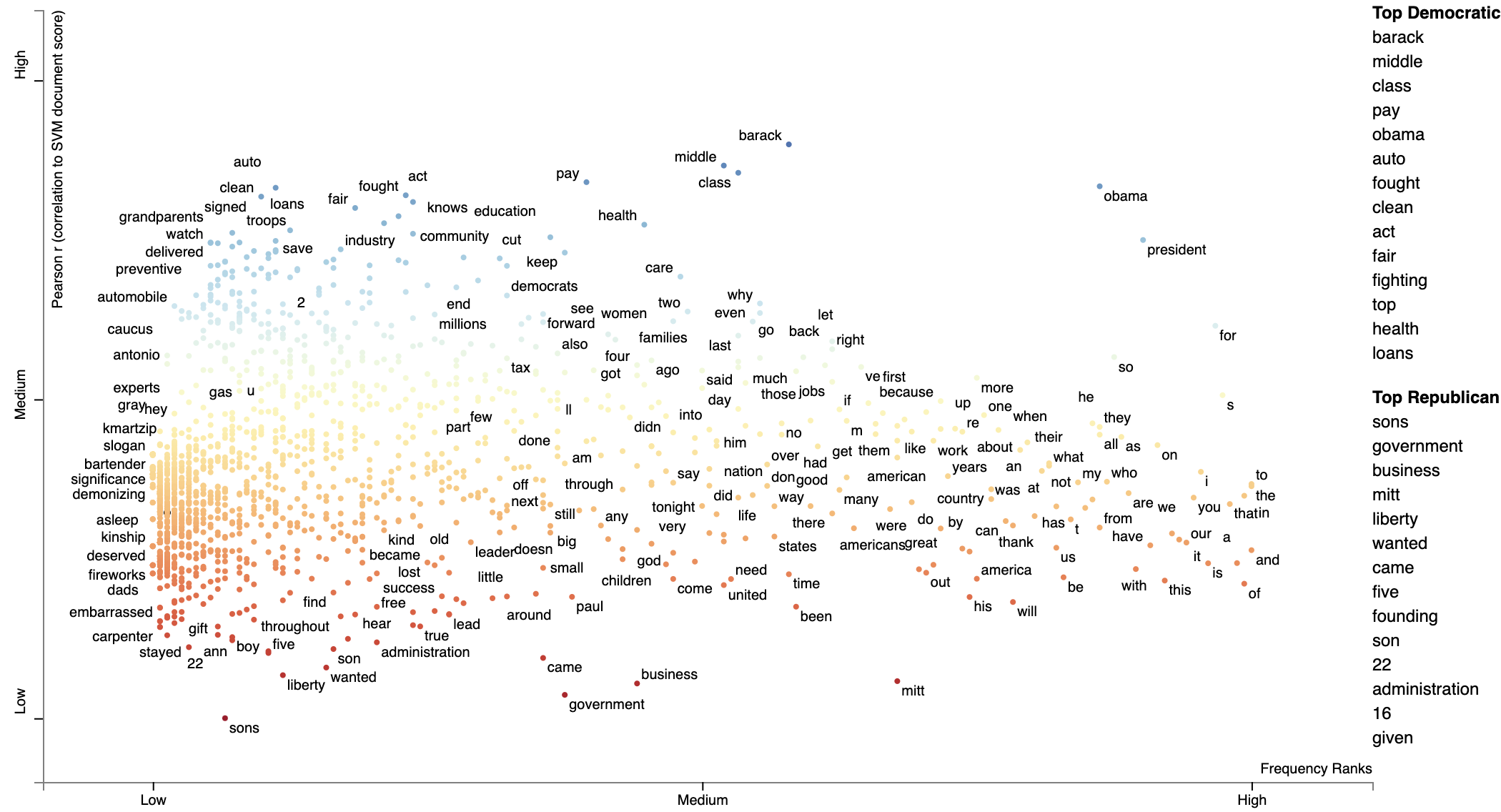### Using Custom Background Word Frequencies

Scattertext relies on a set of general-domain English word frequencies when computing unigram characteristic
scores. When using running Scattertext on non-English data or in a specific domain, the quality of the scores will degrade.

Ensure that you are on Scattertext 0.1.6 or higher.

To remedy this, one can add a custom set of background scores to a Corpus-like object, using the Corpus.set_background_corpus function. The function takes a pd.Series object, indexed on terms with numeric count values.

By default, [!understanding-scaled-f-score](Scaled F-Score) is used to rank how characteristic terms are.

The example below illustrates using Polish background word frequencies.

First, we produce a Series object mapping Polish words to their frequencies using a list from the https://github.com/oprogramador/most-common-words-by-language repo.

polish_word_frequencies = pd.read_csv(
'https://raw.githubusercontent.com/hermitdave/FrequencyWords/master/content/2016/pl/pl_50k.txt',
sep = ' ',
names = ['Word', 'Frequency']
).set_index('Word')['Frequency']


Note the composition of the Series

>>> polish_word_frequencies
Word
nie    5875385
to     4388099
się    3507076
w      2723767
na     2309765
Name: Frequency, dtype: int64


Next, we build a DataFrame, reviews_df, consisting of document which appear (to a non-Polish speaker) to be positive and negative hotel reviews from the https://klejbenchmark.com/tasks/ corpus (Kocoń, et al. 2019). Note this data is under a CC BY-NC-SA 4.0 license. These are labeled as "__label__meta_plus_m" and "__label__meta_minus_m". We will use Scattertext to compare those reviews and determine

nlp = spacy.blank('pl')

with ZipFile(io.BytesIO(urlopen(
'https://klejbenchmark.com/static/data/klej_polemo2.0-in.zip'
lambda df: df.target.isin(['__label__meta_plus_m', '__label__meta_minus_m'])
].assign(
Parse = lambda df: df.sentence.apply(nlp)
)


Next, we wish to create a ParsedCorpus object from review_df. In preparation, we first assemble a list of Polish stopwords from the stopwords repository. We also create the not_a_word regular expression to filter out terms which do not contain a letter.

polish_stopwords = {
stopword for stopword in
urlopen(
'https://raw.githubusercontent.com/bieli/stopwords/master/polish.stopwords.txt'
if stopword.strip()
}

not_a_word = re.compile(r'^\W+$')  With these present, we can build a corpus from review_df with the category being the binary "target" column. We reduce the term space to unigrams and then run the filter_out which takes a function to determine if a term should be removed from the corpus. The function identifies terms which are in the Polish stoplist or do not contain a letter. Finally, terms occurring less than 20 times in the corpus are removed. We set the background frequency Series we created early as the background corpus. corpus = st.CorpusFromParsedDocuments( review_df, category_col='target', parsed_col='Parse' ).build( ).get_unigram_corpus( ).filter_out( lambda term: term in polish_stopwords or not_a_word.match(term) is not None ).remove_infrequent_words( minimum_term_count=20 ).set_background_corpus( polish_word_frequencies )  Note that a minimum word count of 20 was chosen to ensure that only around 2,000 terms would be displayed >>> corpus.get_num_terms() 2023  Running get_term_and_background_counts shows us total term counts in the corpus compare to background frequency counts. We limit this to terms which only occur in the corpus. >>> corpus.get_term_and_background_counts()[ ... lambda df: df.corpus > 0 ... ].sort_values(by='corpus', ascending=False) background corpus m 341583838.0 4819.0 hotelu 33108.0 1812.0 hotel 297974790.0 1651.0 doktor 154840.0 1534.0 polecam 0.0 1438.0 ... ... ... szoku 0.0 21.0 badaniem 0.0 21.0 balkonu 0.0 21.0 stopnia 0.0 21.0 wobec 0.0 21.0  Interesting, the term "polecam" appears very frequently in the corpus, but does not appear at all in the background corpus, making it highly characteristic. Judging from Google Translate, it appears to mean something related to "recommend". We are now ready to display the plot. html = st.produce_scattertext_explorer( corpus, category='__label__meta_plus_m', category_name='Plus-M', not_category_name='Minus-M', minimum_term_frequency=1, width_in_pixels=1000, transform=st.Scalers.dense_rank )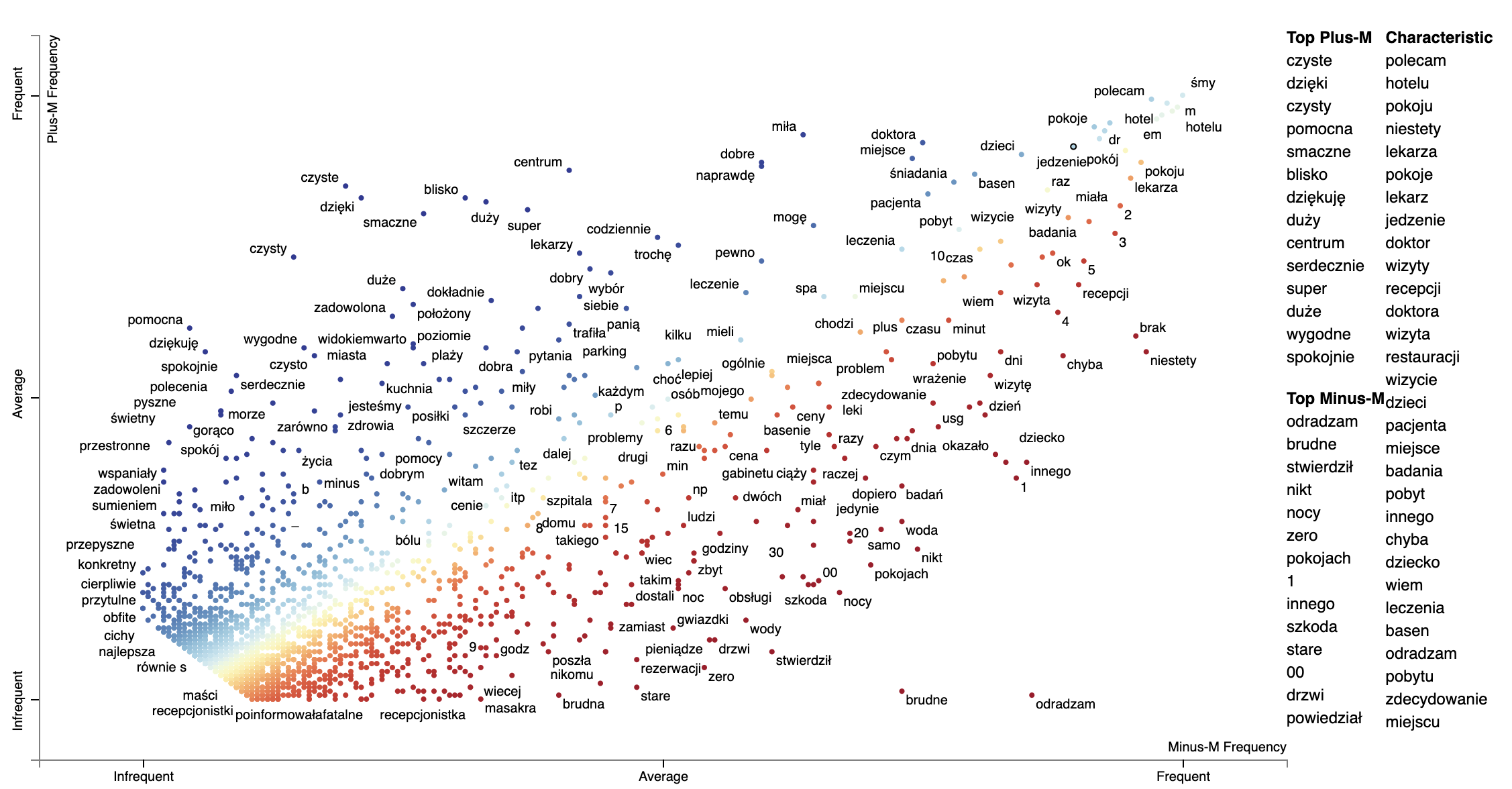We can change the formula which is used to produce the Characteristic scores using the characteristic_scorer parameter to produce_scattertext_explorer. It takes a instance of a descendant of the CharacteristicScorer class. See DenseRankCharacteristicness.py for an example of how to make your own. Example of plotting with a modified characteristic scorer, html = st.produce_scattertext_explorer( corpus, category='__label__meta_plus_m', category_name='Plus-M', not_category_name='Minus-M', minimum_term_frequency=1, transform=st.Scalers.dense_rank, characteristic_scorer=st.DenseRankCharacteristicness(),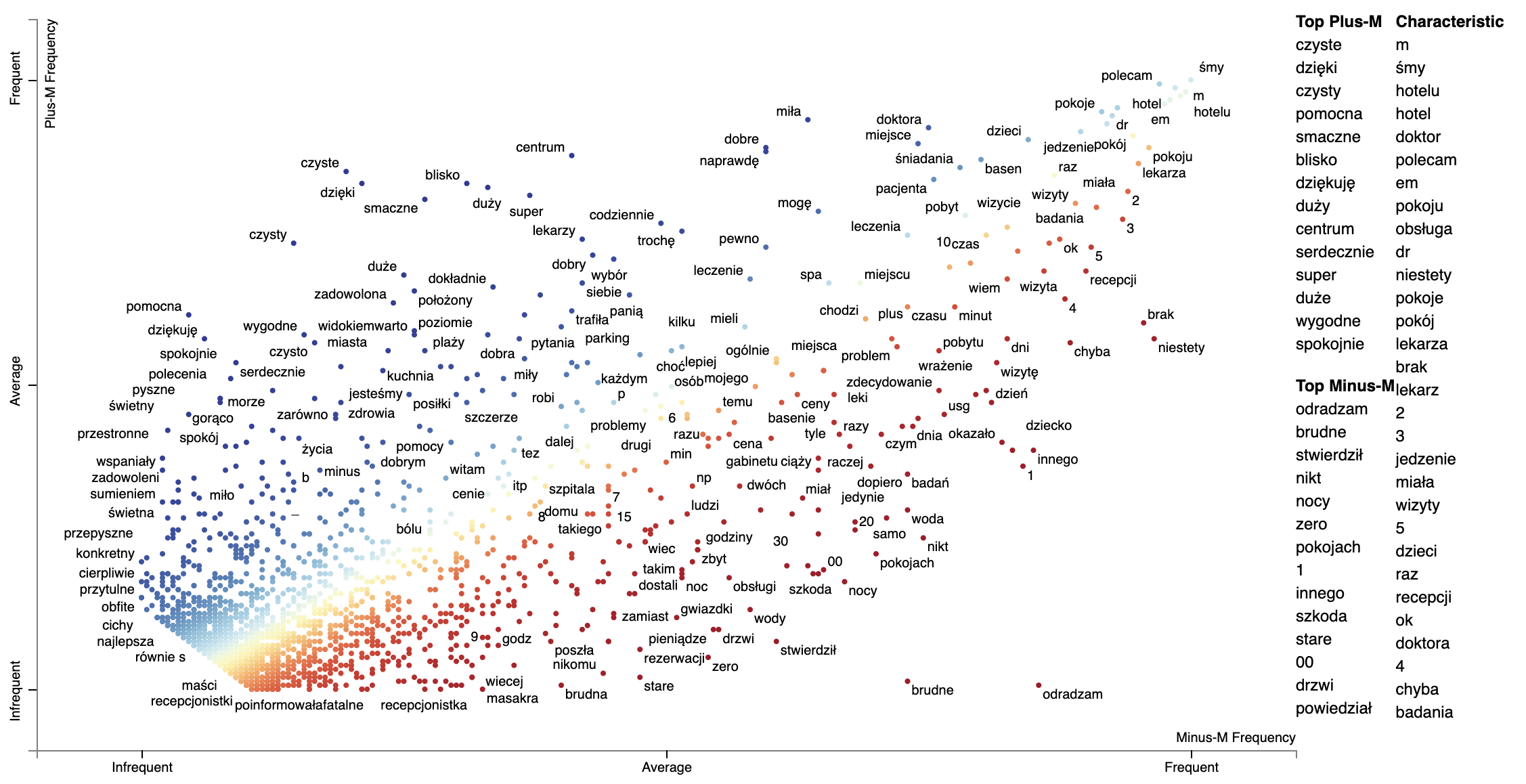Note that numbers show up as more characteristic using the Dense Rank Difference. It may be they occur unusually frequently in this corpus, or perhaps the background word frequencies under counted mumbers. ### Plotting word productivity Word productivity is one strategy for plotting word-based charts describing an uncategorized corpus. Productivity is defined in Schumann (2016) (Jason: check this) as the entropy of ngrams which contain a term. For the entropy computation, the probability of an n-gram wrt the term whose productivity is being calculated is the frequency of the n-gram divided by the term's frequency. Since productivity highly correlates with frequency, the recommended metric to plot is the dense rank difference between frequency and productivity. The snippet below plots words in the convention corpus based on their log frequency and their productivity. The function st.whole_corpus_productivity_scores returns a DataFrame giving each word's productivity. For example, in the convention corpus, Productivity scores should be calculated on a Corpus-like object which contains a complete set of unigrams and at least bigrams. This corpus should not be compacted before the productivity score calculation. The terms with lower productivity have more limited usage (e.g., "thank" for "thank you", "united" for "united steates") while the terms with higher productivity occurr in a wider varity of contexts ("getting", "actually", "political", etc.). import spacy import scattertext as st corpus_no_cat = st.CorpusWithoutCategoriesFromParsedDocuments( st.SampleCorpora.ConventionData2012.get_data().assign( Parse=lambda df: [x for x in spacy.load('en_core_web_sm').pipe(df.text)]), parsed_col='Parse' ).build() compact_corpus_no_cat = corpus_no_cat.get_stoplisted_unigram_corpus().remove_infrequent_words(9) plot_df = st.whole_corpus_productivity_scores(corpus_no_cat).assign( RankDelta = lambda df: st.RankDifference().get_scores( a=df.Productivity, b=df.Frequency ) ).reindex( compact_corpus_no_cat.get_terms() ).dropna().assign( X=lambda df: df.Frequency, Xpos=lambda df: st.Scalers.log_scale(df.Frequency), Y=lambda df: df.RankDelta, Ypos=lambda df: st.Scalers.scale(df.RankDelta), ) html = st.dataframe_scattertext( compact_corpus_no_cat.whitelist_terms(plot_df.index), plot_df=plot_df, metadata=lambda df: df.get_df()['speaker'], ignore_categories=True, x_label='Rank Frequency', y_label="Productivity", left_list_column='Ypos', color_score_column='Ypos', y_axis_labels=['Least Productive', 'Average Productivity', 'Most Productive'], header_names={'upper': 'Most Productive', 'lower': 'Least Productive', 'right': 'Characteristic'}, horizontal_line_y_position=0 )### Understanding Scaled F-Score Let's now turn our attention to a novel term scoring metric, Scaled F-Score. We'll examine this on a unigram version of the Rotten Tomatoes corpus (Pang et al. 2002). It contains excerpts of positive and negative movie reviews. Please see Scaled F Score Explanation for a notebook version of this analysis.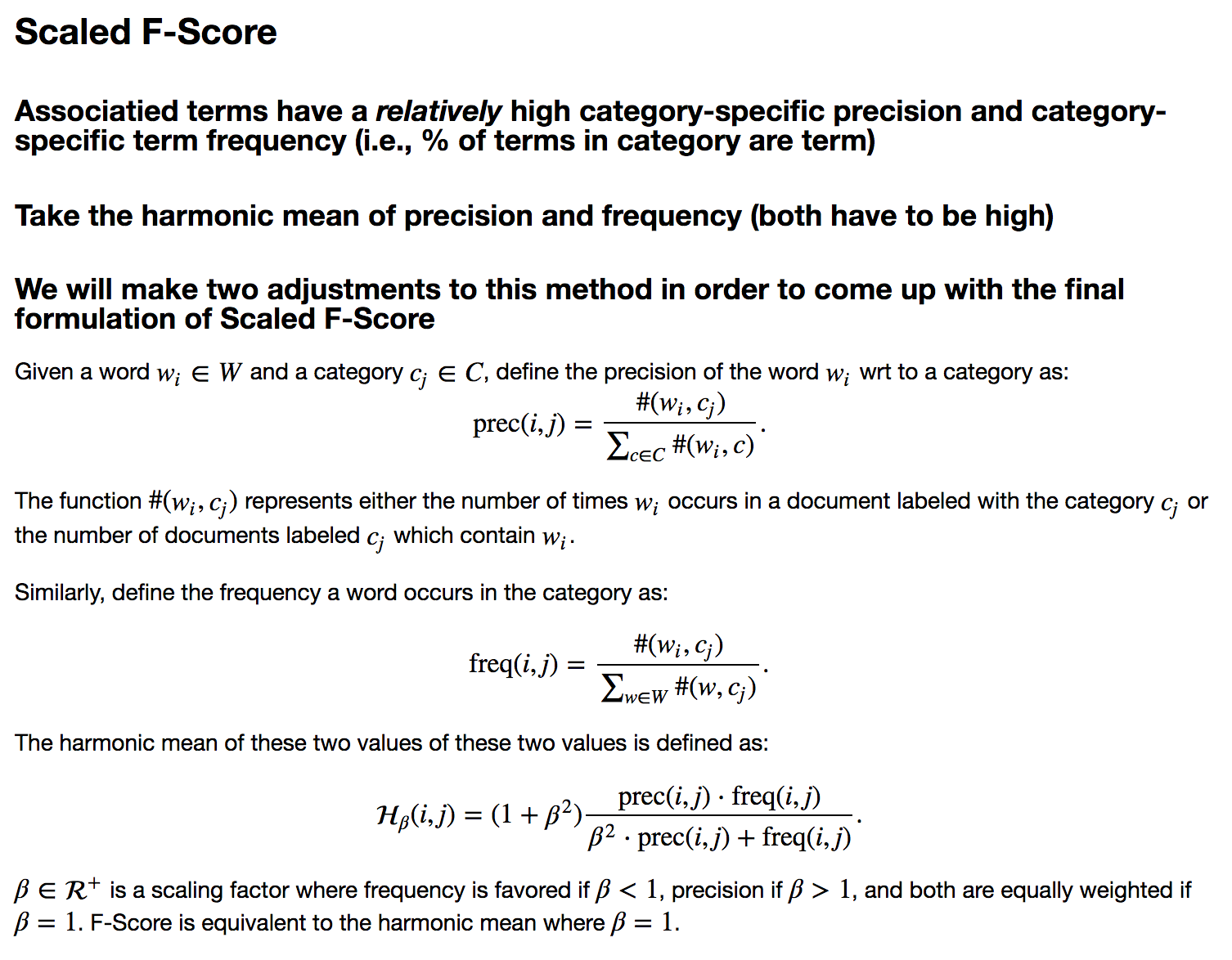from scipy.stats import hmean term_freq_df = corpus.get_unigram_corpus().get_term_freq_df()[['Positive freq', 'Negative freq']] term_freq_df = term_freq_df[term_freq_df.sum(axis=1) > 0] term_freq_df['pos_precision'] = (term_freq_df['Positive freq'] * 1./ (term_freq_df['Positive freq'] + term_freq_df['Negative freq'])) term_freq_df['pos_freq_pct'] = (term_freq_df['Positive freq'] * 1. /term_freq_df['Positive freq'].sum()) term_freq_df['pos_hmean'] = (term_freq_df .apply(lambda x: (hmean([x['pos_precision'], x['pos_freq_pct']]) if x['pos_precision'] > 0 and x['pos_freq_pct'] > 0 else 0), axis=1)) term_freq_df.sort_values(by='pos_hmean', ascending=False).iloc[:10]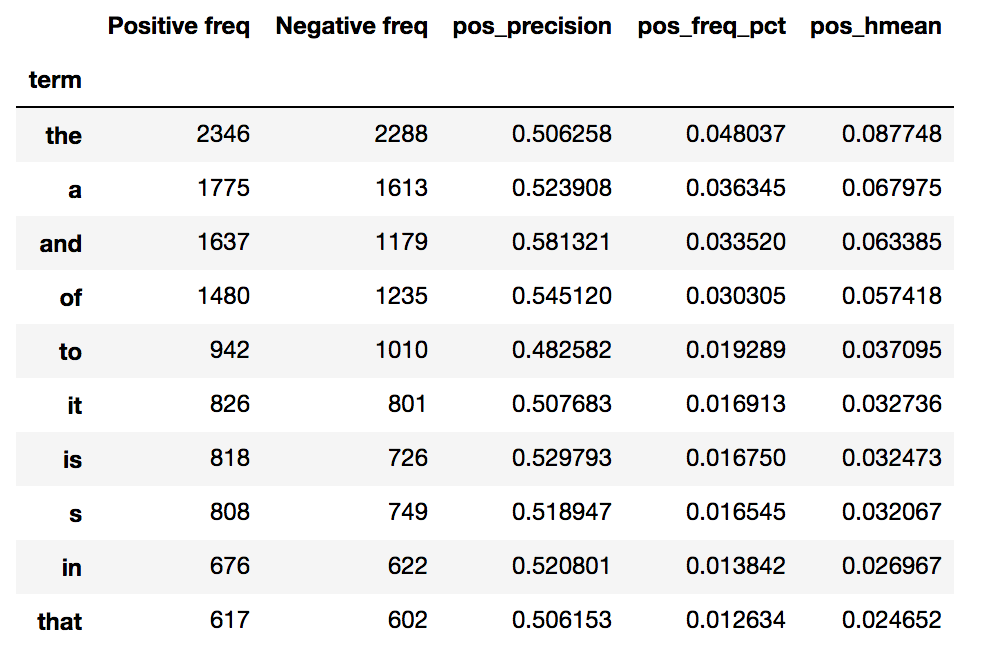If we plot term frequency on the x-axis and the percentage of a term's occurrences which are in positive documents (i.e., its precision) on the y-axis, we can see that low-frequency terms have a much higher variation in the precision. Given these terms have low frequencies, the harmonic means are low. Thus, the only terms which have a high harmonic mean are extremely frequent words which tend to all have near average precisions. freq = term_freq_df.pos_freq_pct.values prec = term_freq_df.pos_precision.values html = st.produce_scattertext_explorer( corpus.remove_terms(set(corpus.get_terms()) - set(term_freq_df.index)), category='Positive', not_category_name='Negative', not_categories=['Negative'], x_label = 'Portion of words used in positive reviews', original_x = freq, x_coords = (freq - freq.min())/freq.max(), x_axis_values = [int(freq.min()*1000)/1000., int(freq.max() * 1000)/1000.], y_label = 'Portion of documents containing word that are positive', original_y = prec, y_coords = (prec - prec.min())/prec.max(), y_axis_values = [int(prec.min() * 1000)/1000., int((prec.max()/2.)*1000)/1000., int(prec.max() * 1000)/1000.], scores = term_freq_df.pos_hmean.values, sort_by_dist=False, show_characteristic=False ) file_name = 'not_normed_freq_prec.html' open(file_name, 'wb').write(html.encode('utf-8')) IFrame(src=file_name, width = 1300, height=700)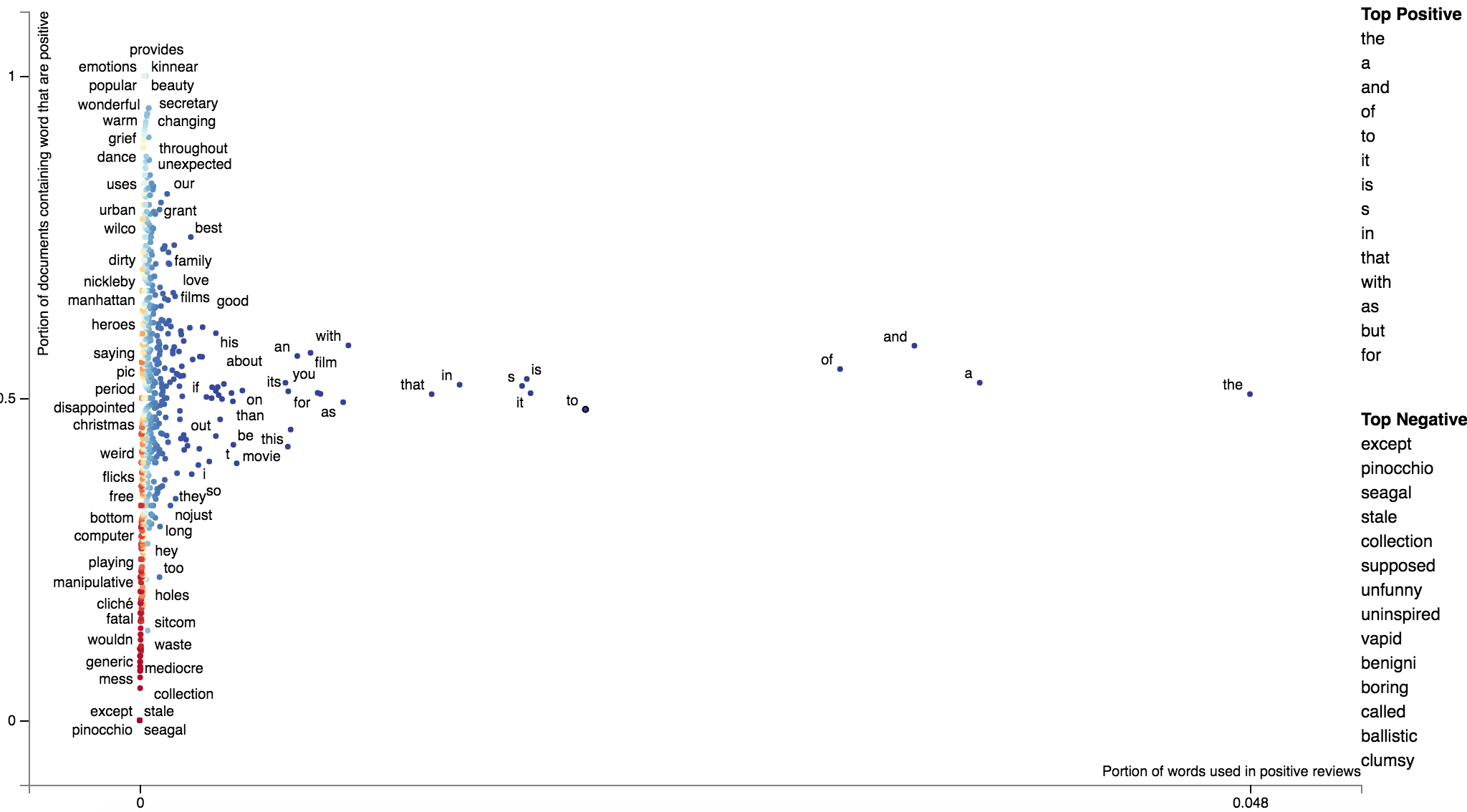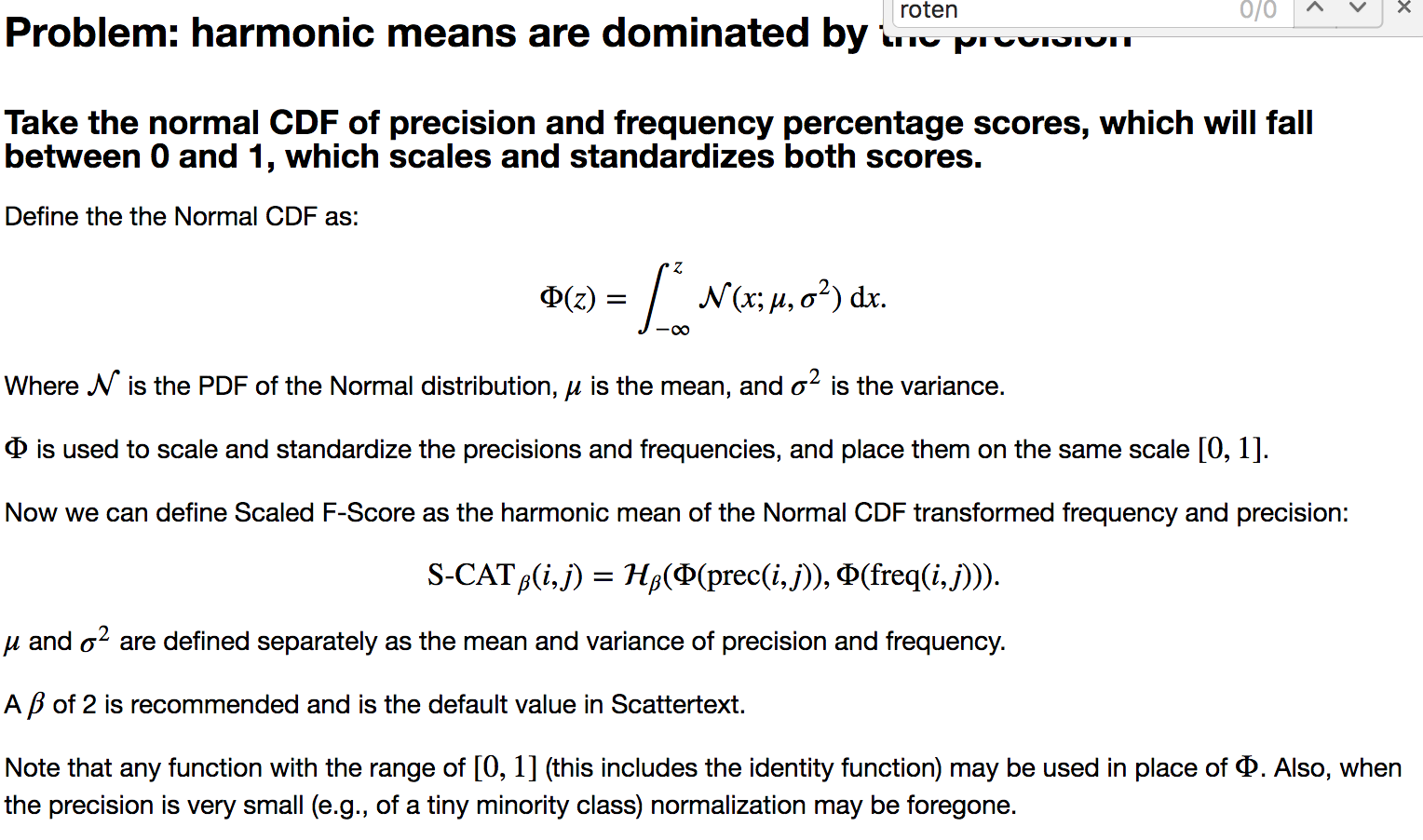from scipy.stats import norm def normcdf(x): return norm.cdf(x, x.mean(), x.std ()) term_freq_df['pos_precision_normcdf'] = normcdf(term_freq_df.pos_precision) term_freq_df['pos_freq_pct_normcdf'] = normcdf(term_freq_df.pos_freq_pct.values) term_freq_df['pos_scaled_f_score'] = hmean([term_freq_df['pos_precision_normcdf'], term_freq_df['pos_freq_pct_normcdf']]) term_freq_df.sort_values(by='pos_scaled_f_score', ascending=False).iloc[:10]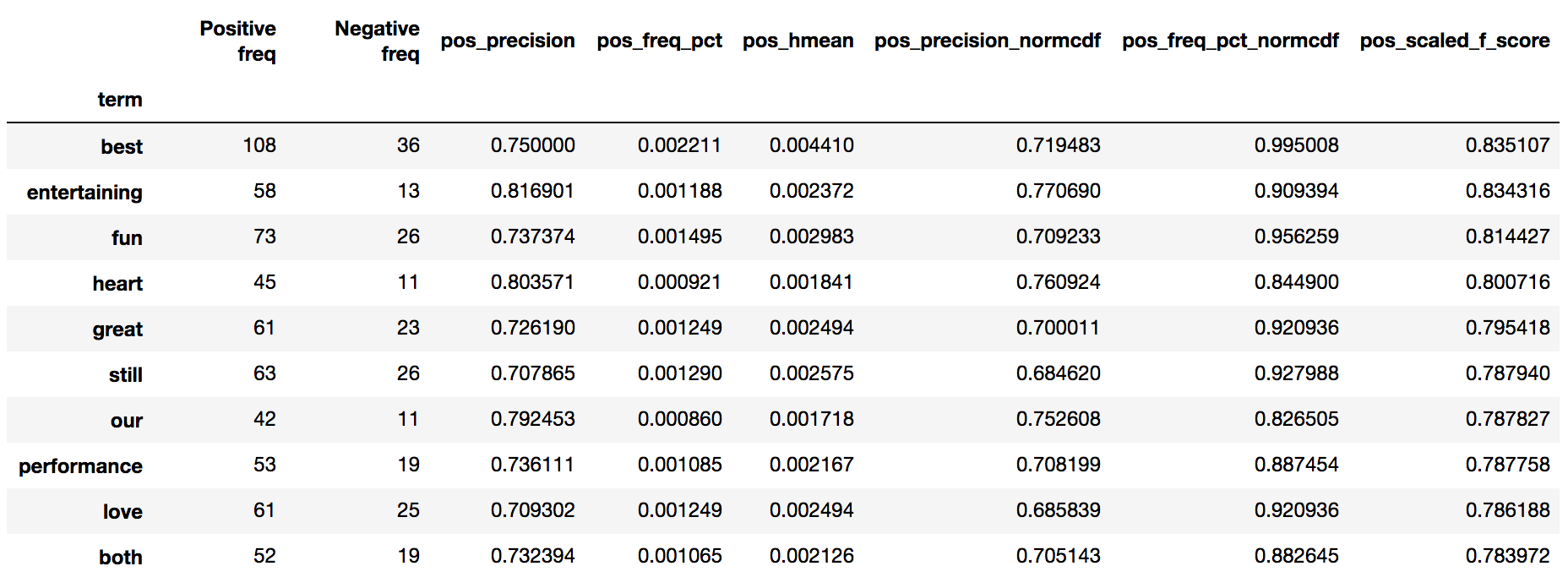freq = term_freq_df.pos_freq_pct_normcdf.values prec = term_freq_df.pos_precision_normcdf.values html = st.produce_scattertext_explorer( corpus.remove_terms(set(corpus.get_terms()) - set(term_freq_df.index)), category='Positive', not_category_name='Negative', not_categories=['Negative'], x_label = 'Portion of words used in positive reviews (norm-cdf)', original_x = freq, x_coords = (freq - freq.min())/freq.max(), x_axis_values = [int(freq.min()*1000)/1000., int(freq.max() * 1000)/1000.], y_label = 'documents containing word that are positive (norm-cdf)', original_y = prec, y_coords = (prec - prec.min())/prec.max(), y_axis_values = [int(prec.min() * 1000)/1000., int((prec.max()/2.)*1000)/1000., int(prec.max() * 1000)/1000.], scores = term_freq_df.pos_scaled_f_score.values, sort_by_dist=False, show_characteristic=False )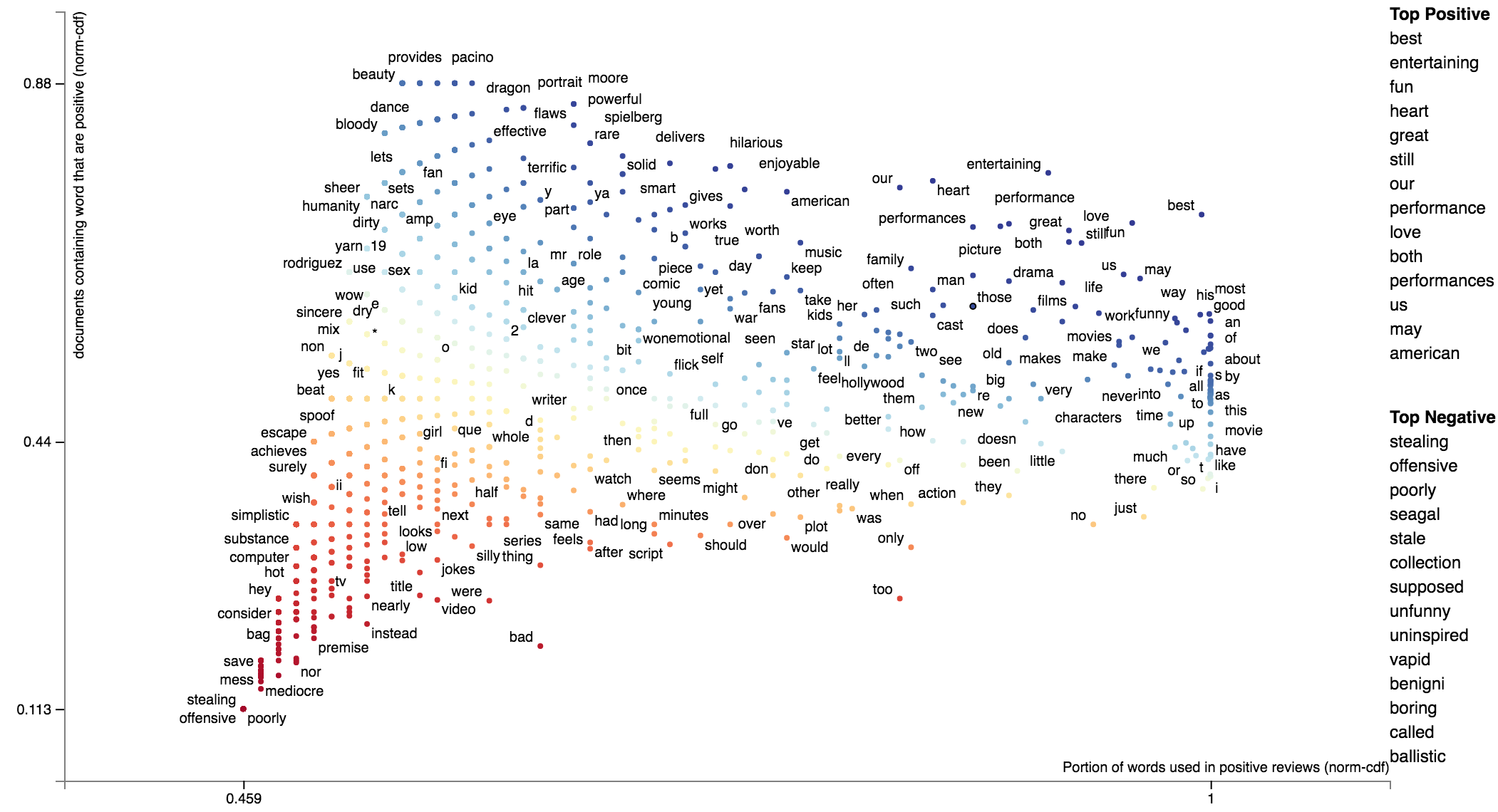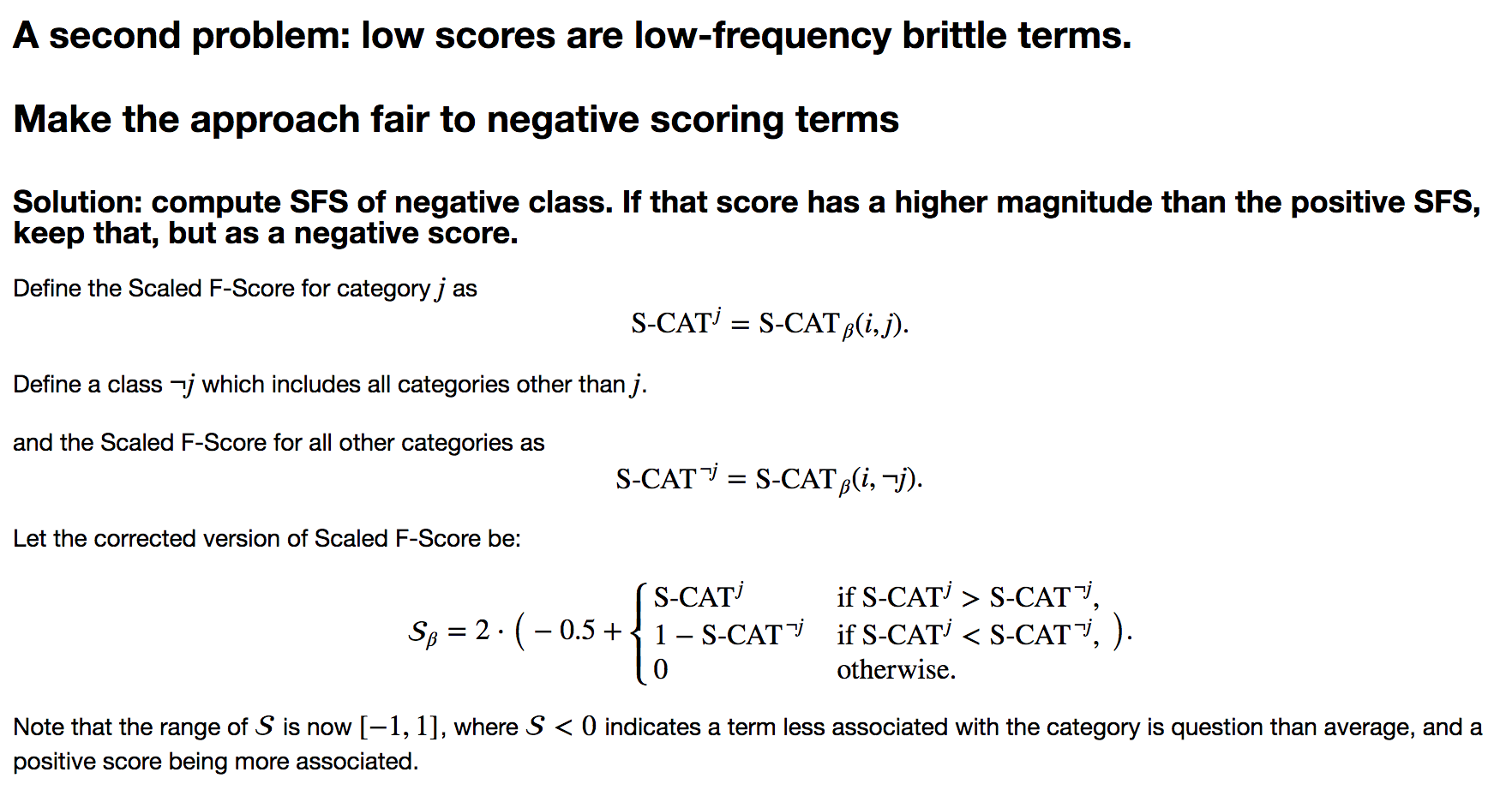term_freq_df['neg_precision_normcdf'] = normcdf((term_freq_df['Negative freq'] * 1./ (term_freq_df['Negative freq'] + term_freq_df['Positive freq']))) term_freq_df['neg_freq_pct_normcdf'] = normcdf((term_freq_df['Negative freq'] * 1. /term_freq_df['Negative freq'].sum())) term_freq_df['neg_scaled_f_score'] = hmean([term_freq_df['neg_precision_normcdf'], term_freq_df['neg_freq_pct_normcdf']]) term_freq_df['scaled_f_score'] = 0 term_freq_df.loc[term_freq_df['pos_scaled_f_score'] > term_freq_df['neg_scaled_f_score'], 'scaled_f_score'] = term_freq_df['pos_scaled_f_score'] term_freq_df.loc[term_freq_df['pos_scaled_f_score'] < term_freq_df['neg_scaled_f_score'], 'scaled_f_score'] = 1-term_freq_df['neg_scaled_f_score'] term_freq_df['scaled_f_score'] = 2 * (term_freq_df['scaled_f_score'] - 0.5) term_freq_df.sort_values(by='scaled_f_score', ascending=True).iloc[:10]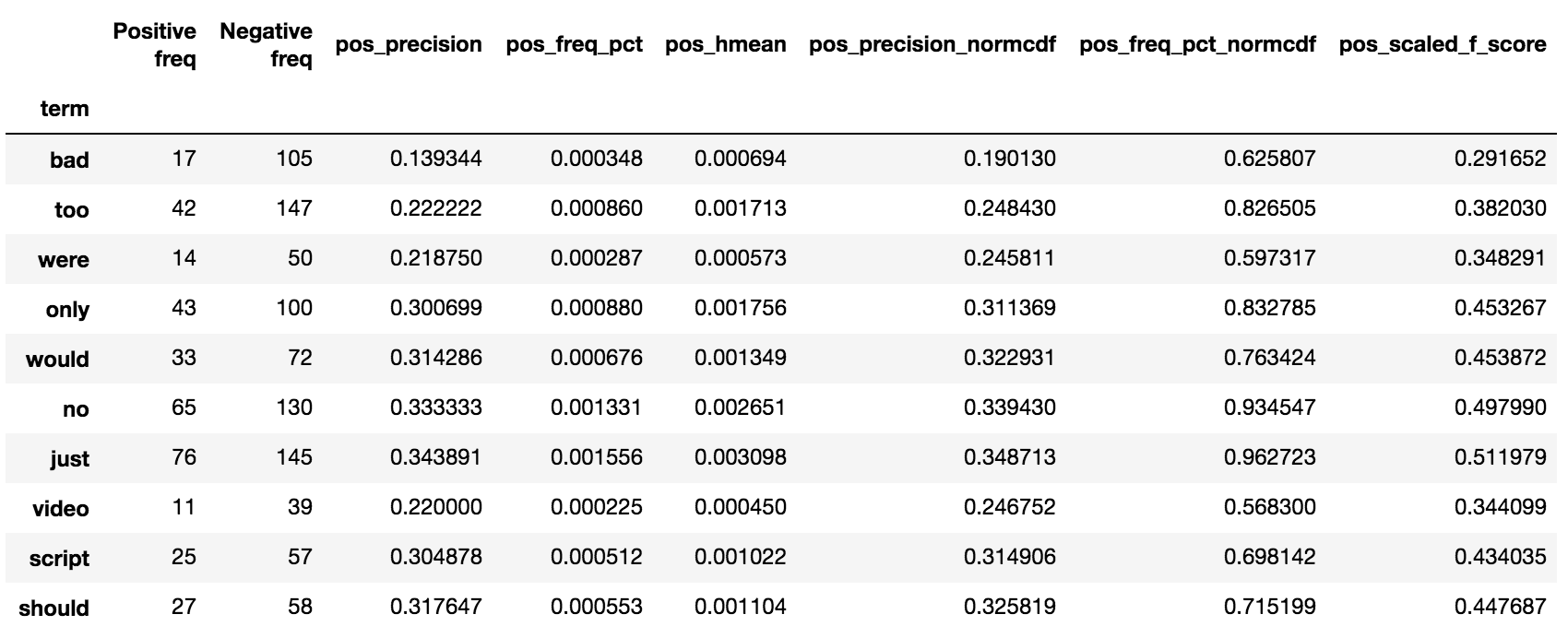is_pos = term_freq_df.pos_scaled_f_score > term_freq_df.neg_scaled_f_score freq = term_freq_df.pos_freq_pct_normcdf*is_pos - term_freq_df.neg_freq_pct_normcdf*~is_pos prec = term_freq_df.pos_precision_normcdf*is_pos - term_freq_df.neg_precision_normcdf*~is_pos def scale(ar): return (ar - ar.min())/(ar.max() - ar.min()) def close_gap(ar): ar[ar > 0] -= ar[ar > 0].min() ar[ar < 0] -= ar[ar < 0].max() return ar html = st.produce_scattertext_explorer( corpus.remove_terms(set(corpus.get_terms()) - set(term_freq_df.index)), category='Positive', not_category_name='Negative', not_categories=['Negative'], x_label = 'Frequency', original_x = freq, x_coords = scale(close_gap(freq)), x_axis_labels = ['Frequent in Neg', 'Not Frequent', 'Frequent in Pos'], y_label = 'Precision', original_y = prec, y_coords = scale(close_gap(prec)), y_axis_labels = ['Neg Precise', 'Imprecise', 'Pos Precise'], scores = (term_freq_df.scaled_f_score.values + 1)/2, sort_by_dist=False, show_characteristic=False )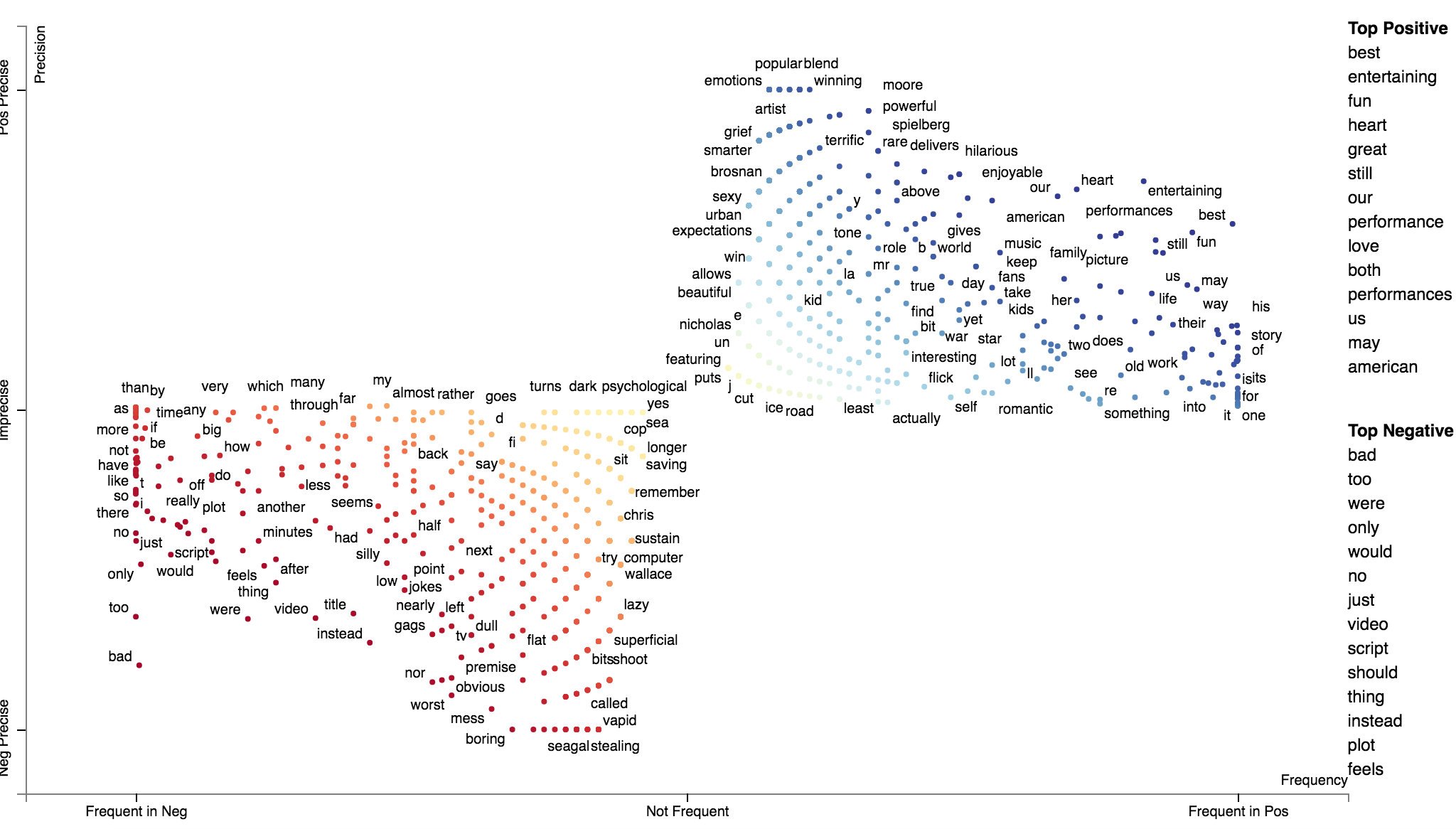We can use st.ScaledFScorePresets as a term scorer to display terms' Scaled F-Score on the y-axis and term frequencies on the x-axis. html = st.produce_frequency_explorer( corpus.remove_terms(set(corpus.get_terms()) - set(term_freq_df.index)), category='Positive', not_category_name='Negative', not_categories=['Negative'], term_scorer=st.ScaledFScorePresets(beta=1, one_to_neg_one=True), metadata = rdf['movie_name'], grey_threshold=0 )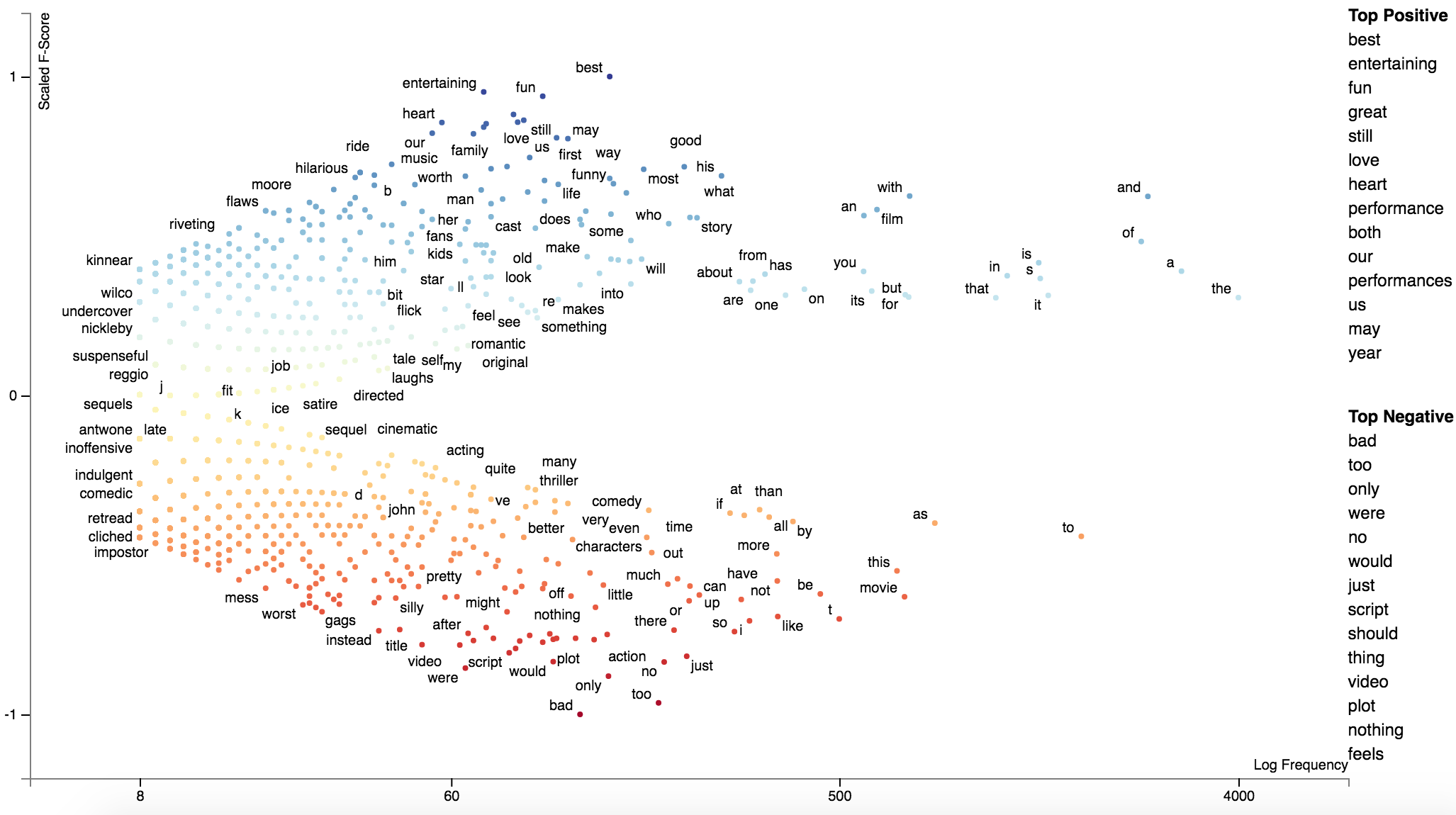### Alternative term scoring methods Scaled F-Score is not the only scoring method included in Scattertext. Please click on one of the links below to view a notebook which describes how other class association scores work and can be visualized through Scattertext. New in 0.0.2.73 is the delta JS-Divergence scorer DeltaJSDivergence scorer (Gallagher et al. 2020), and its corresponding compactor (JSDCompactor.) See demo_deltajsd.py for an example usage. ### The position-select-plot process New in 0.0.2.72 Scattertext was originally set up to visualize corpora objects, which are connected sets of documents and terms to visualize. The "compaction" process allows users to eliminate terms which may not be associated with a category using a variety of feature selection methods. The issue with this is that the terms eliminated during the selection process are not taken into account when scaling term positions. This issue can be mitigated by using the position-select-plot process, where term positions are pre-determined before the selection process is made. Let's first use the 2012 conventions corpus, update the category names, and create a unigram corpus. import scattertext as st import numpy as np df = st.SampleCorpora.ConventionData2012.get_data().assign( parse=lambda df: df.text.apply(st.whitespace_nlp_with_sentences) ).assign(party=lambda df: df['party'].apply({'democrat': 'Democratic', 'republican': 'Republican'}.get)) corpus = st.CorpusFromParsedDocuments( df, category_col='party', parsed_col='parse' ).build().get_unigram_corpus() category_name = 'Democratic' not_category_name = 'Republican'  Next, let's create a dataframe consisting of the original counts and their log-scale positions. def get_log_scale_df(corpus, y_category, x_category): term_coord_df = corpus.get_term_freq_df('') # Log scale term counts (with a smoothing constant) as the initial coordinates coord_columns = [] for category in [y_category, x_category]: col_name = category + '_coord' term_coord_df[col_name] = np.log(term_coord_df[category] + 1e-6) / np.log(2) coord_columns.append(col_name) # Scale these coordinates to between 0 and 1 min_offset = term_coord_df[coord_columns].min(axis=0).min() for coord_column in coord_columns: term_coord_df[coord_column] -= min_offset max_offset = term_coord_df[coord_columns].max(axis=0).max() for coord_column in coord_columns: term_coord_df[coord_column] /= max_offset return term_coord_df # Get term coordinates from original corpus term_coordinates = get_log_scale_df(corpus, category_name, not_category_name) print(term_coordinates)  Here is a preview of the term_coordinates dataframe. The Democrat and Republican columns contain the term counts, while the _coord columns contain their logged coordinates. Visualizing 7,973 terms is difficult (but possible) for people running Scattertext on most computers.  Democratic Republican Democratic_coord Republican_coord term thank 158 205 0.860166 0.872032 you 836 794 0.936078 0.933729 so 337 212 0.894681 0.873562 much 84 76 0.831380 0.826820 very 62 75 0.817543 0.826216 ... ... ... ... ... precinct 0 2 0.000000 0.661076 godspeed 0 1 0.000000 0.629493 beauty 0 1 0.000000 0.629493 bumper 0 1 0.000000 0.629493 sticker 0 1 0.000000 0.629493 [7973 rows x 4 columns]  We can visualize this full data set by running the following code block. We'll create a custom Javascript function to populate the tooltip with the original term counts, and create a Scattertext Explorer where the x and y coordinates and original values are specified from the data frame. Additionally, we can use show_diagonal=True to draw a dashed diagonal line across the plot area. You can click the chart below to see the interactive version. Note that it will take a while to load. # The tooltip JS function. Note that d is is the term data object, and ox and oy are the original x- and y- # axis counts. get_tooltip_content = ('(function(d) {return d.term + "<br/>' + not_category_name + ' Count: " ' + '+ d.ox +"<br/>' + category_name + ' Count: " + d.oy})') html_orig = st.produce_scattertext_explorer( corpus, category=category_name, not_category_name=not_category_name, minimum_term_frequency=0, pmi_threshold_coefficient=0, width_in_pixels=1000, metadata=corpus.get_df()['speaker'], show_diagonal=True, original_y=term_coordinates[category_name], original_x=term_coordinates[not_category_name], x_coords=term_coordinates[category_name + '_coord'], y_coords=term_coordinates[not_category_name + '_coord'], max_overlapping=3, use_global_scale=True, get_tooltip_content=get_tooltip_content, )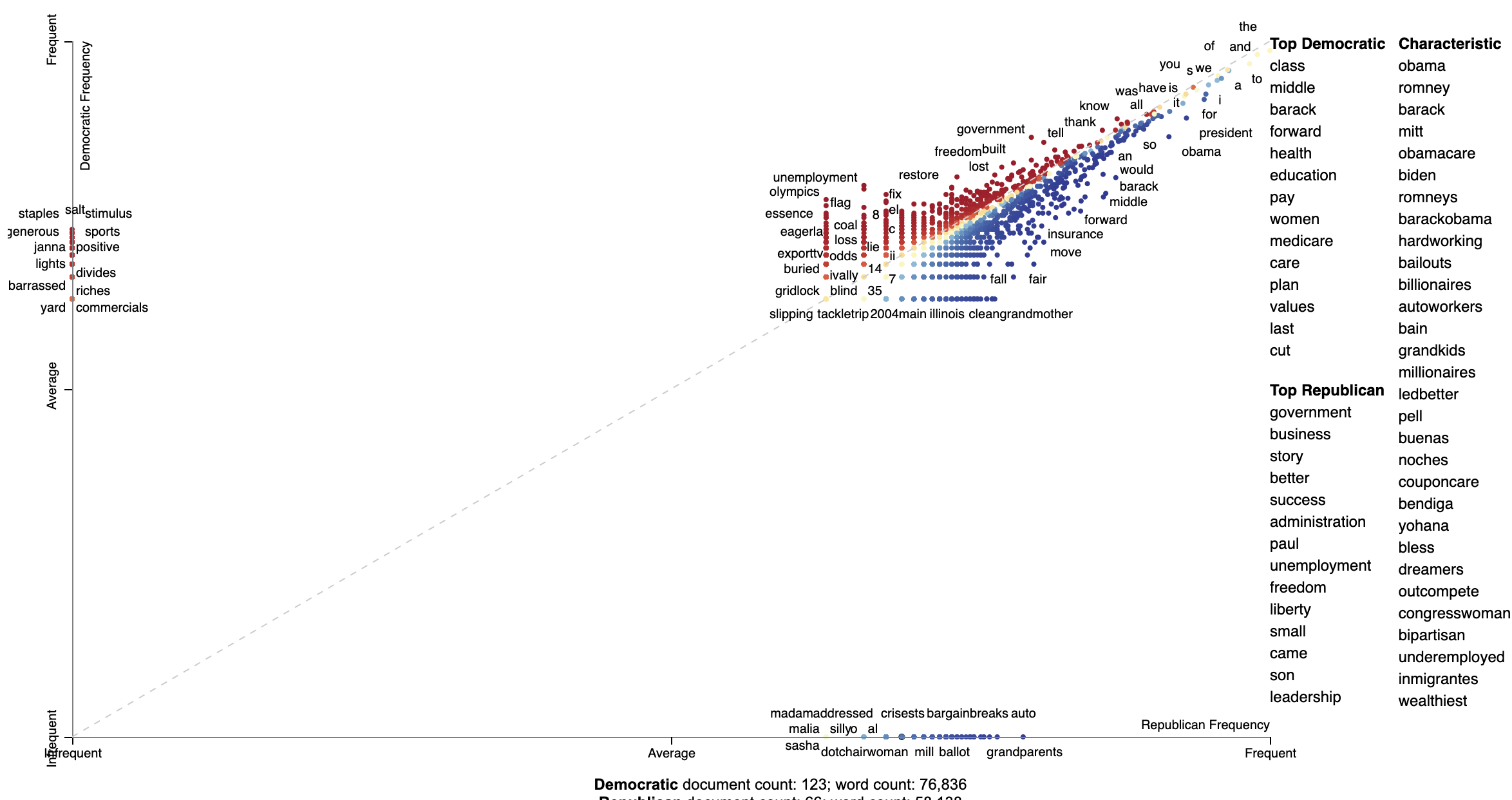Next, we can visualize the compacted version of the corpus. The compaction, using ClassPercentageCompactor, selects terms which frequently in each category. The term_count parameter, set to 2, is used to determine the percentage threshold for terms to keep in a particular category. This is done using by calculating the percentile of terms (types) in each category which appear more than two times. We find the smallest percentile, and only include terms which occur above that percentile in a given category. Note that this compaction leaves only 2,828 terms. This number is much easier for Scattertext to display in a browser. # Select terms which appear a minimum threshold in both corpora compact_corpus = corpus.compact(st.ClassPercentageCompactor(term_count=2)) # Only take term coordinates of terms remaining in corpus term_coordinates = term_coordinates.loc[compact_corpus.get_terms()] html_compact = st.produce_scattertext_explorer( compact_corpus, category=category_name, not_category_name=not_category_name, minimum_term_frequency=0, pmi_threshold_coefficient=0, width_in_pixels=1000, metadata=corpus.get_df()['speaker'], show_diagonal=True, original_y=term_coordinates[category_name], original_x=term_coordinates[not_category_name], x_coords=term_coordinates[category_name + '_coord'], y_coords=term_coordinates[not_category_name + '_coord'], max_overlapping=3, use_global_scale=True, get_tooltip_content=get_tooltip_content, )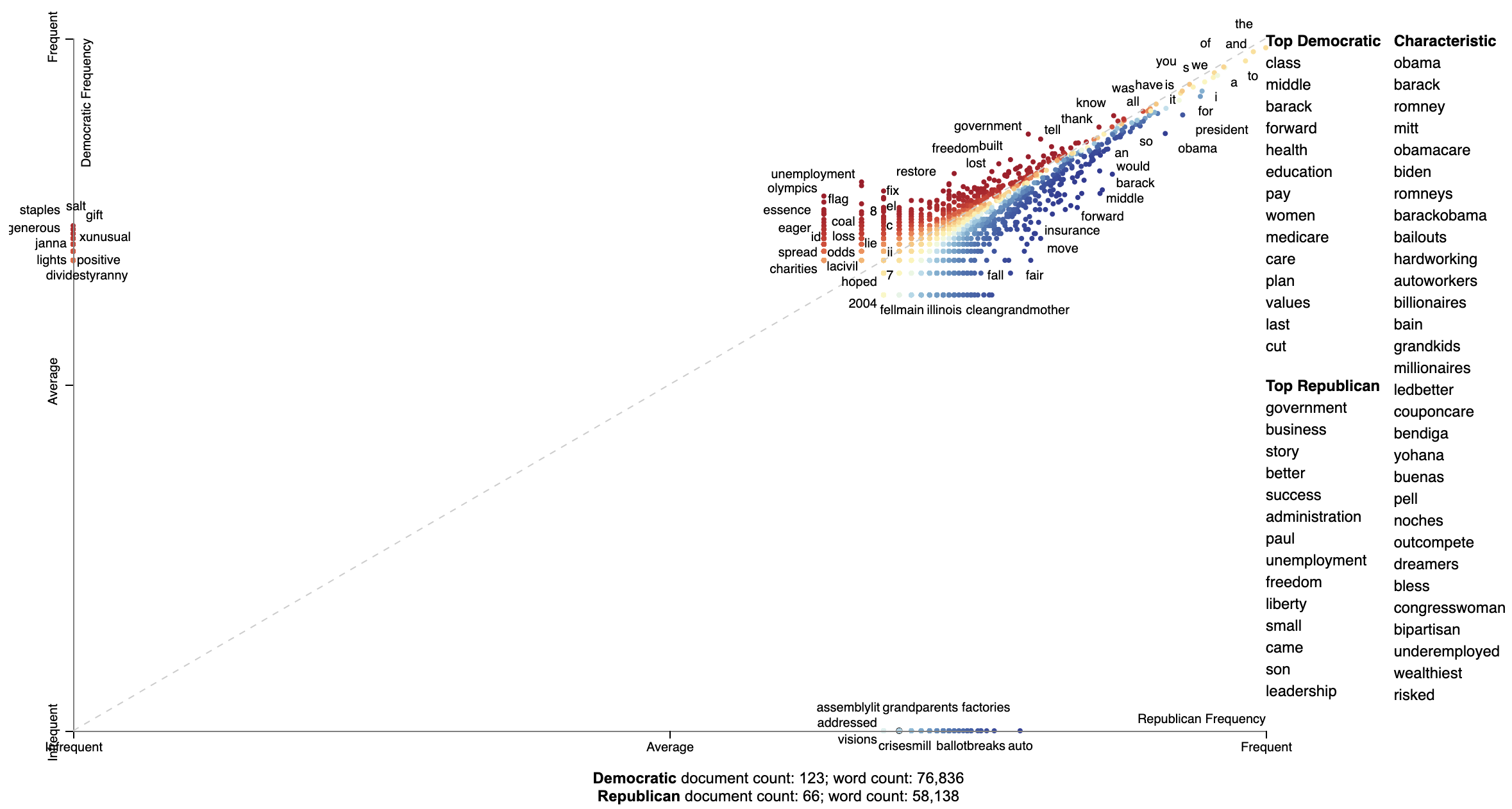## Advanced uses ### Visualizing differences based on only term frequencies Occasionally, only term frequency statistics are available. This may happen in the case of very large, lost, or proprietary data sets. TermCategoryFrequencies is a corpus representation,that can accept this sort of data, along with any categorized documents that happen to be available. Let use the Corpus of Contemporary American English as an example. We'll construct a visualization to analyze the difference between spoken American English and English that occurs in fiction. df = (pd.read_excel('https://www.wordfrequency.info/files/genres_sample.xls') .dropna() .set_index('lemma')[['SPOKEN', 'FICTION']] .iloc[:1000]) df.head() ''' SPOKEN FICTION lemma the 3859682.0 4092394.0 I 1346545.0 1382716.0 they 609735.0 352405.0 she 212920.0 798208.0 would 233766.0 229865.0 '''  Transforming this into a visualization is extremely easy. Just pass a dataframe indexed on terms with columns indicating category-counts into the the TermCategoryFrequencies constructor. term_cat_freq = st.TermCategoryFrequencies(df)  And call produce_scattertext_explorer normally: html = st.produce_scattertext_explorer( term_cat_freq, category='SPOKEN', category_name='Spoken', not_category_name='Fiction', )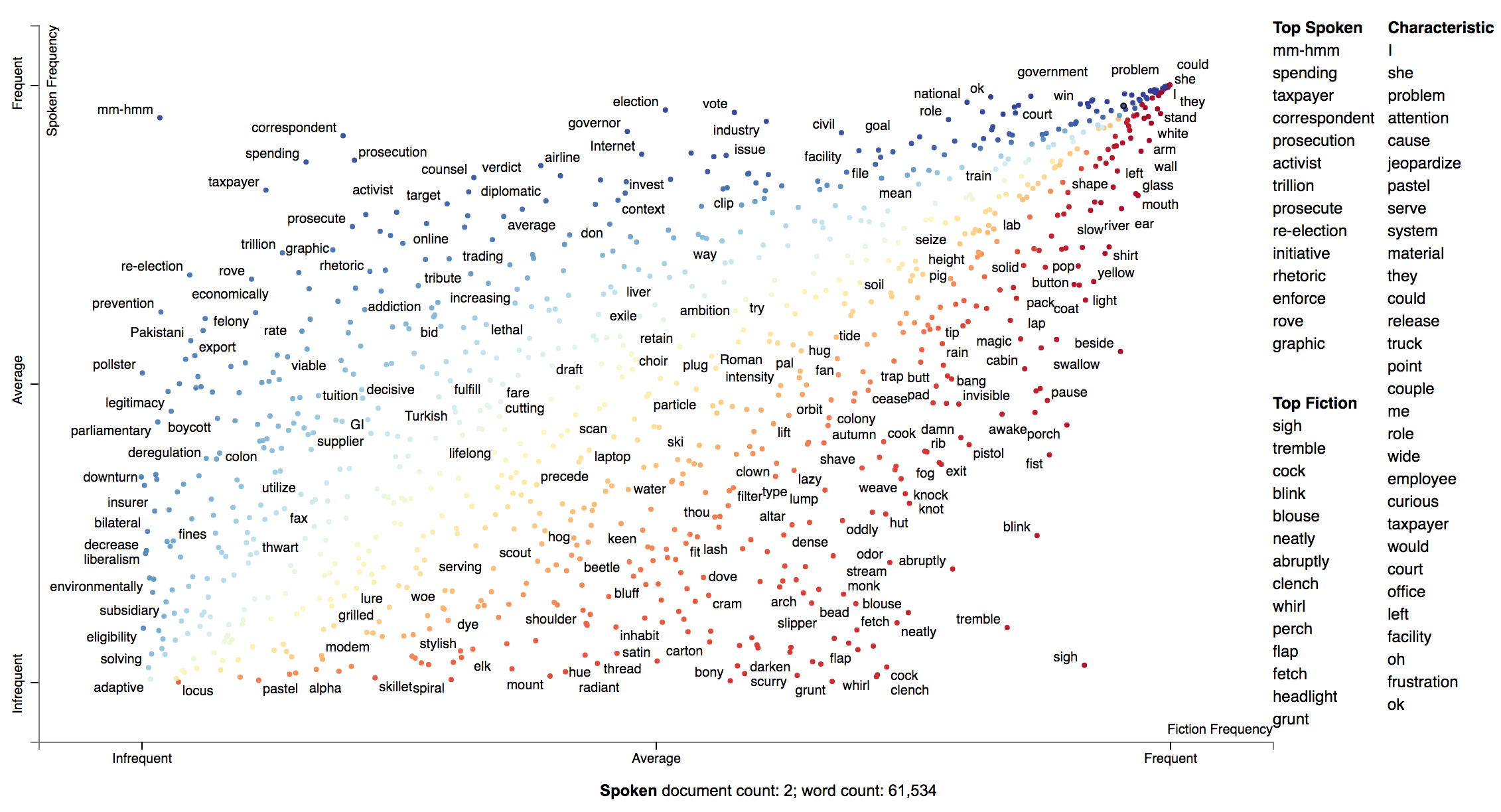If you'd like to incorporate some documents into the visualization, you can add them into to the TermCategoyFrequencies object. First, let's extract some example Fiction and Spoken documents from the sample COCA corpus. import requests, zipfile, io coca_sample_url = 'http://corpus.byu.edu/cocatext/samples/text.zip' zip_file = zipfile.ZipFile(io.BytesIO(requests.get(coca_sample_url).content)) document_df = pd.DataFrame( [{'text': zip_file.open(fn).read().decode('utf-8'), 'category': 'SPOKEN'} for fn in zip_file.filelist if fn.filename.startswith('w_spok')][:2] + [{'text': zip_file.open(fn).read().decode('utf-8'), 'category': 'FICTION'} for fn in zip_file.filelist if fn.filename.startswith('w_fic')][:2])  And we'll pass the documents_df dataframe into TermCategoryFrequencies via the document_category_df parameter. Ensure the dataframe has two columns, 'text' and 'category'. Afterward, we can call produce_scattertext_explorer (or your visualization function of choice) normally. doc_term_cat_freq = st.TermCategoryFrequencies(df, document_category_df=document_df) html = st.produce_scattertext_explorer( doc_term_cat_freq, category='SPOKEN', category_name='Spoken', not_category_name='Fiction', )  ### Visualizing query-based categorical differences Word representations have recently become a hot topic in NLP. While lots of work has been done visualizing how terms relate to one another given their scores (e.g., http://projector.tensorflow.org/), none to my knowledge has been done visualizing how we can use these to examine how document categories differ. In this example given a query term, "jobs", we can see how Republicans and Democrats talk about it differently. In this configuration of Scattertext, words are colored by their similarity to a query phrase. This is done using spaCy-provided GloVe word vectors (trained on the Common Crawl corpus). The cosine distance between vectors is used, with mean vectors used for phrases. The calculation of the most similar terms associated with each category is a simple heuristic. First, sets of terms closely associated with a category are found. Second, these terms are ranked based on their similarity to the query, and the top rank terms are displayed to the right of the scatterplot. A term is considered associated if its p-value is less than 0.05. P-values are determined using Monroe et al. (2008)'s difference in the weighted log-odds-ratios with an uninformative Dirichlet prior. This is the only model-based method discussed in Monroe et al. that does not rely on a large, in-domain background corpus. Since we are scoring bigrams in addition to the unigrams scored by Monroe, the size of the corpus would have to be larger to have high enough bigram counts for proper penalization. This function relies the Dirichlet distribution's parameter alpha, a vector, which is uniformly set to 0.01. Here is the code to produce such a visualization. >>> from scattertext import word_similarity_explorer >>> html = word_similarity_explorer(corpus, ... category='democrat', ... category_name='Democratic', ... not_category_name='Republican', ... target_term='jobs', ... minimum_term_frequency=5, ... pmi_threshold_coefficient=4, ... width_in_pixels=1000, ... metadata=convention_df['speaker'], ... alpha=0.01, ... max_p_val=0.05, ... save_svg_button=True) >>> open("Convention-Visualization-Jobs.html", 'wb').write(html.encode('utf-8'))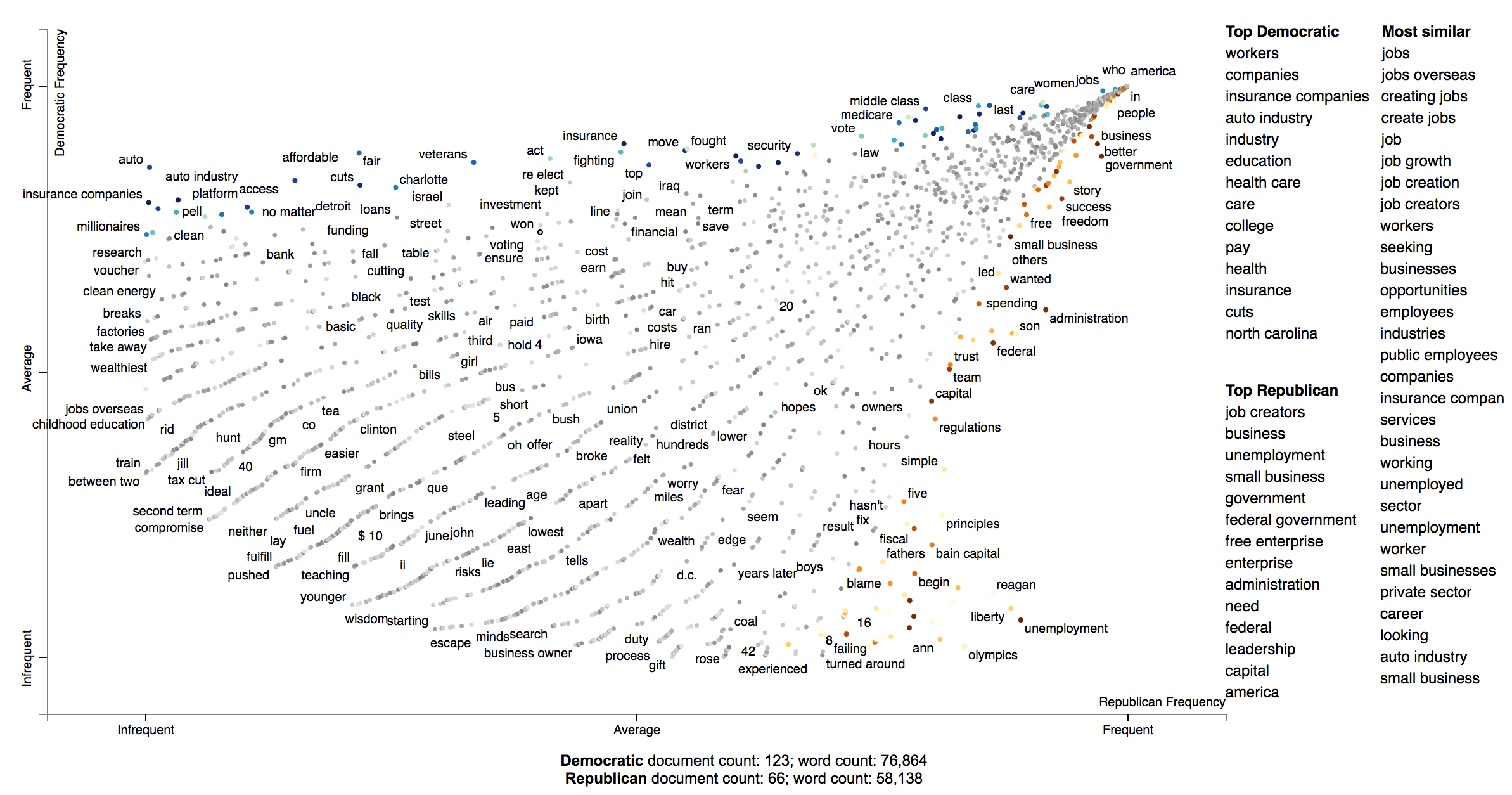#### Developing and using bespoke word representations Scattertext can interface with Gensim Word2Vec models. For example, here's a snippet from demo_gensim_similarity.py which illustrates how to train and use a word2vec model on a corpus. Note the similarities produced reflect quirks of the corpus, e.g., "8" tends to refer to the 8% unemployment rate at the time of the convention. import spacy from gensim.models import word2vec from scattertext import SampleCorpora, word_similarity_explorer_gensim, Word2VecFromParsedCorpus from scattertext.CorpusFromParsedDocuments import CorpusFromParsedDocuments nlp = spacy.en.English() convention_df = SampleCorpora.ConventionData2012.get_data() convention_df['parsed'] = convention_df.text.apply(nlp) corpus = CorpusFromParsedDocuments(convention_df, category_col='party', parsed_col='parsed').build() model = word2vec.Word2Vec(size=300, alpha=0.025, window=5, min_count=5, max_vocab_size=None, sample=0, seed=1, workers=1, min_alpha=0.0001, sg=1, hs=1, negative=0, cbow_mean=0, iter=1, null_word=0, trim_rule=None, sorted_vocab=1) html = word_similarity_explorer_gensim(corpus, category='democrat', category_name='Democratic', not_category_name='Republican', target_term='jobs', minimum_term_frequency=5, pmi_threshold_coefficient=4, width_in_pixels=1000, metadata=convention_df['speaker'], word2vec=Word2VecFromParsedCorpus(corpus, model).train(), max_p_val=0.05, save_svg_button=True) open('./demo_gensim_similarity.html', 'wb').write(html.encode('utf-8'))  How Democrats and Republicans talked differently about "jobs" in their 2012 convention speeches.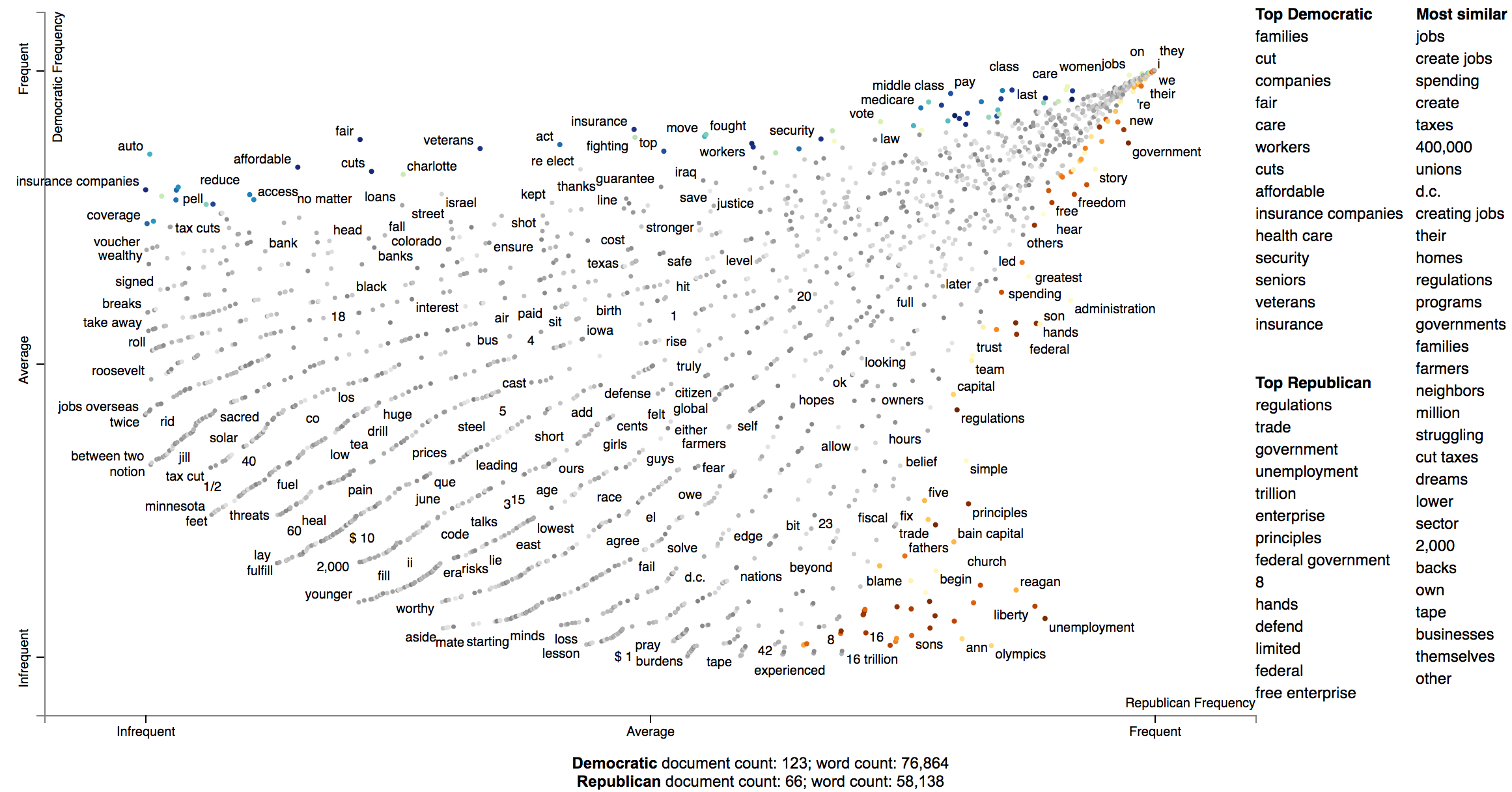### Visualizing any kind of term score We can use Scattertext to visualize alternative types of word scores, and ensure that 0 scores are greyed out. Use the sparse_explroer function to acomplish this, and see its source code for more details. >>> from sklearn.linear_model import Lasso >>> from scattertext import sparse_explorer >>> html = sparse_explorer(corpus, ... category='democrat', ... category_name='Democratic', ... not_category_name='Republican', ... scores = corpus.get_regression_coefs('democrat', Lasso(max_iter=10000)), ... minimum_term_frequency=5, ... pmi_threshold_coefficient=4, ... width_in_pixels=1000, ... metadata=convention_df['speaker']) >>> open('./Convention-Visualization-Sparse.html', 'wb').write(html.encode('utf-8'))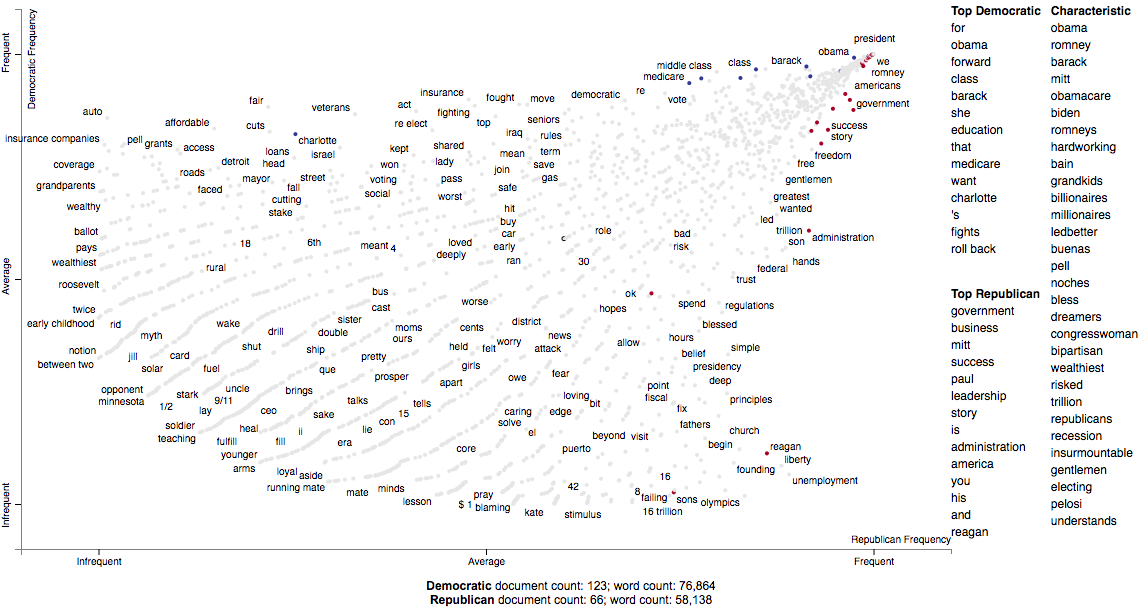### Custom term positions You can also use custom term positions and axis labels. For example, you can base terms' y-axis positions on a regression coefficient and their x-axis on term frequency and label the axes accordingly. The one catch is that axis positions must be scaled between 0 and 1. First, let's define two scaling functions: scale to project positive values to [0,1], and zero_centered_scale project real values to [0,1], with negative values always <0.5, and positive values always >0.5. >>> def scale(ar): ... return (ar - ar.min()) / (ar.max() - ar.min()) ... >>> def zero_centered_scale(ar): ... ar[ar > 0] = scale(ar[ar > 0]) ... ar[ar < 0] = -scale(-ar[ar < 0]) ... return (ar + 1) / 2.  Next, let's compute and scale term frequencies and L2-penalized regression coefficients. We'll hang on to the original coefficients and allow users to view them by mousing over terms. >>> from sklearn.linear_model import LogisticRegression >>> import numpy as np >>> >>> frequencies_scaled = scale(np.log(term_freq_df.sum(axis=1).values)) >>> scores = corpus.get_logreg_coefs('democrat', ... LogisticRegression(penalty='l2', C=10, max_iter=10000, n_jobs=-1)) >>> scores_scaled = zero_centered_scale(scores)  Finally, we can write the visualization. Note the use of the x_coords and y_coords parameters to store the respective coordinates, the scores and sort_by_dist arguments to register the original coefficients and use them to rank the terms in the right-hand list, and the x_label and y_label arguments to label axes. >>> html = produce_scattertext_explorer(corpus, ... category='democrat', ... category_name='Democratic', ... not_category_name='Republican', ... minimum_term_frequency=5, ... pmi_threshold_coefficient=4, ... width_in_pixels=1000, ... x_coords=frequencies_scaled, ... y_coords=scores_scaled, ... scores=scores, ... sort_by_dist=False, ... metadata=convention_df['speaker'], ... x_label='Log frequency', ... y_label='L2-penalized logistic regression coef') >>> open('demo_custom_coordinates.html', 'wb').write(html.encode('utf-8'))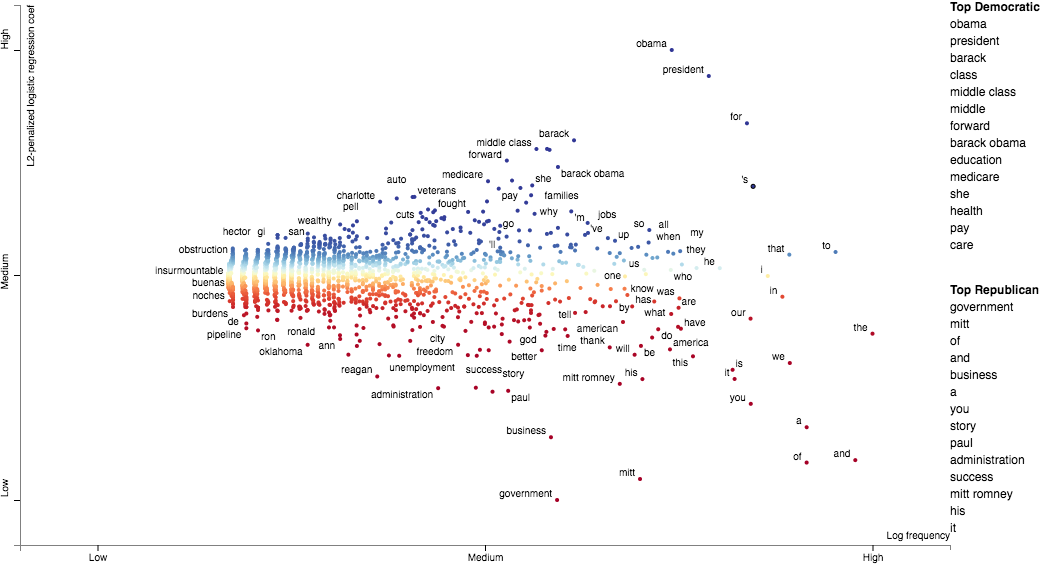### Emoji analysis The Emoji analysis capability displays a chart of the category-specific distribution of Emoji. Let's look at a new corpus, a set of tweets. We'll build a visualization showing how men and women use emoji differently. Note: the following example is implemented in demo_emoji.py. First, we'll load the dataset and parse it using NLTK's tweet tokenizer. Note, install NLTK before running this example. It will take some time for the dataset to download. import nltk, urllib.request, io, agefromname, zipfile import scattertext as st import pandas as pd with zipfile.ZipFile(io.BytesIO(urllib.request.urlopen( 'http://followthehashtag.com/content/uploads/USA-Geolocated-tweets-free-dataset-Followthehashtag.zip' ).read())) as zf: df = pd.read_excel(zf.open('dashboard_x_usa_x_filter_nativeretweets.xlsx')) nlp = st.tweet_tokenzier_factory(nltk.tokenize.TweetTokenizer()) df['parse'] = df['Tweet content'].apply(nlp) df.iloc ''' Tweet Id 721318437075685382 Date 2016-04-16 Hour 12:44 User Name Bill Schulhoff Nickname BillSchulhoff Bio Husband,Dad,GrandDad,Ordained Minister, Umpire... Tweet content Wind 3.2 mph NNE. Barometer 30.20 in, Rising s... Favs NaN RTs NaN Latitude 40.7603 Longitude -72.9547 Country US Place (as appears on Bio) East Patchogue, NY Profile picture http://pbs.twimg.com/profile_images/3788000007... Followers 386 Following 705 Listed 24 Tweet language (ISO 639-1) en Tweet Url http://www.twitter.com/BillSchulhoff/status/72... parse Wind 3.2 mph NNE. Barometer 30.20 in, Rising s... Name: 0, dtype: object '''  Next, we'll use the AgeFromName package to find the probabilities of the gender of each user given their first name. First, we'll find a dataframe indexed on first names that contains the probability that each someone with that first name is male (male_prob). male_prob = agefromname.AgeFromName().get_all_name_male_prob() male_prob.iloc ''' hi 1.00000 lo 0.95741 prob 1.00000 Name: aaban, dtype: float64 '''  Next, we'll extract the first names of each user, and use the male_prob data frame to find users whose names indicate there is at least a 90% chance they are either male or female, label those users, and create new data frame df_mf with only those users. df['first_name'] = df['User Name'].apply(lambda x: x.split().lower() if type(x) == str and len(x.split()) > 0 else x) df_aug = pd.merge(df, male_prob, left_on='first_name', right_index=True) df_aug['gender'] = df_aug['prob'].apply(lambda x: 'm' if x > 0.9 else 'f' if x < 0.1 else '?') df_mf = df_aug[df_aug['gender'].isin(['m', 'f'])]  The key to this analysis is to construct a corpus using only the emoji extractor st.FeatsFromSpacyDocOnlyEmoji which builds a corpus only from emoji and not from anything else. corpus = st.CorpusFromParsedDocuments( df_mf, parsed_col='parse', category_col='gender', feats_from_spacy_doc=st.FeatsFromSpacyDocOnlyEmoji() ).build()  Next, we'll run this through a standard produce_scattertext_explorer visualization generation. html = st.produce_scattertext_explorer( corpus, category='f', category_name='Female', not_category_name='Male', use_full_doc=True, term_ranker=OncePerDocFrequencyRanker, sort_by_dist=False, metadata=(df_mf['User Name'] + ' (@' + df_mf['Nickname'] + ') ' + df_mf['Date'].astype(str)), width_in_pixels=1000 ) open("EmojiGender.html", 'wb').write(html.encode('utf-8'))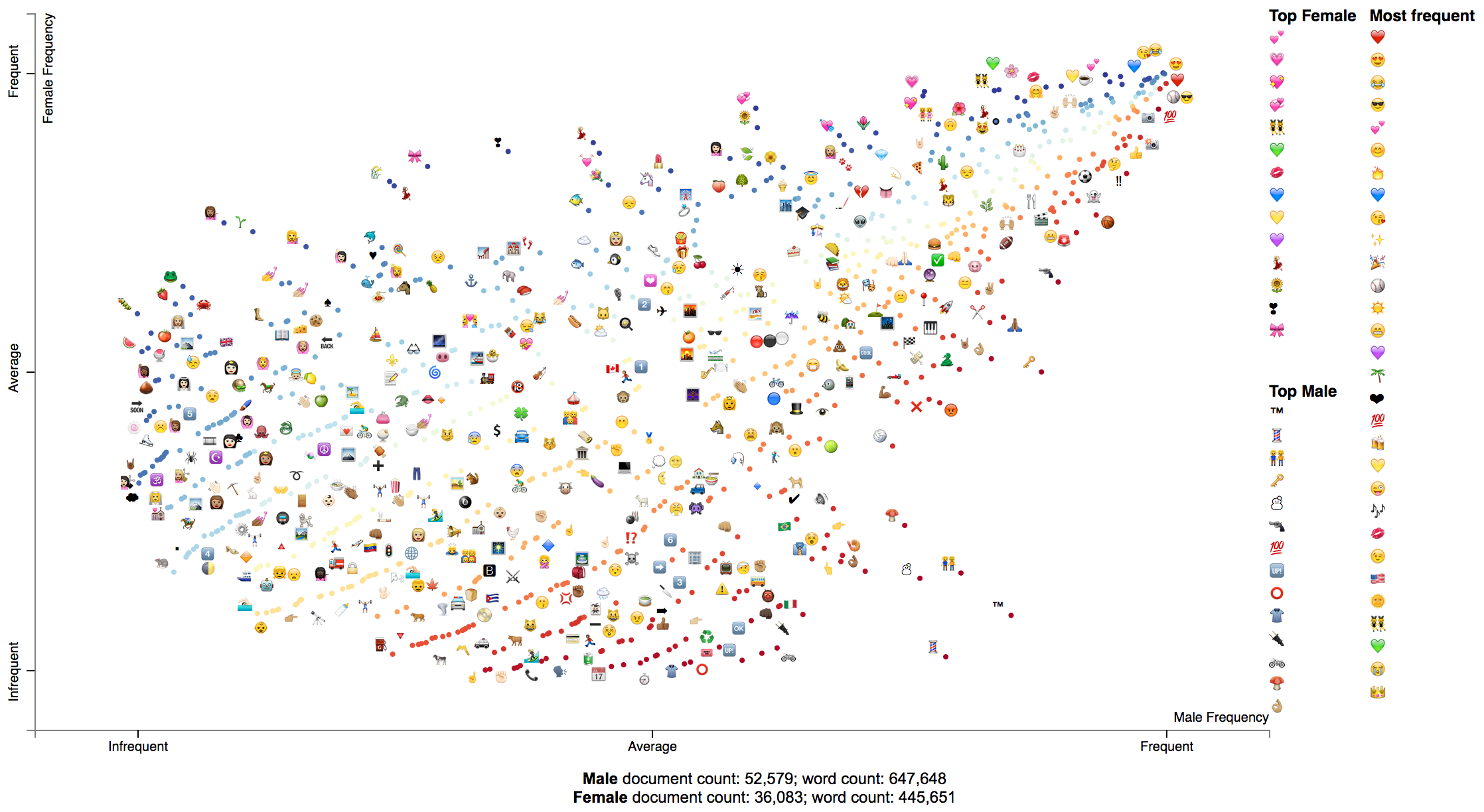### Visualizing SentencePiece Tokens SentencePiece tokenization is a subword tokenization technique which relies on a language-model to produce optimized tokenization. It has been used in large, transformer-based contextual language models. Ensure to run $ pip install sentencepiece before running this example.

First, let's load the political convention data set as normal.

import tempfile
import re
import scattertext as st

convention_df = st.SampleCorpora.ConventionData2012.get_data()
convention_df['parse'] = convention_df.text.apply(st.whitespace_nlp_with_sentences)


Next, let's train a SentencePiece tokenizer based on this data. The train_sentence_piece_tokenizer function trains a SentencePieceProcessor on the data set and returns it. You can of course use any SentencePieceProcessor.


def train_sentence_piece_tokenizer(documents, vocab_size):
'''
:param documents: list-like, a list of str documents
:vocab_size int: the size of the vocabulary to output

:return sentencepiece.SentencePieceProcessor
'''
import sentencepiece as spm
sp = None
with tempfile.NamedTemporaryFile(delete=True) as tempf:
with tempfile.NamedTemporaryFile(delete=True) as tempm:
tempf.write(('\n'.join(documents)).encode())
spm.SentencePieceTrainer.Train(
'--input=%s --model_prefix=%s --vocab_size=%s' % (tempf.name, tempm.name, vocab_size)
)
sp = spm.SentencePieceProcessor()
return sp
sp = train_sentence_piece_tokenizer(convention_df.text.values, vocab_size=2000)



Next, let's add the SentencePiece tokens as metadata when creating our corpus. In order to do this, pass a FeatsFromSentencePiece instance into the feats_from_spacy_doc parameter. Pass the SentencePieceProcessor into the constructor.

corpus = st.CorpusFromParsedDocuments(convention_df,
parsed_col='parse',
category_col='party',
feats_from_spacy_doc=st.FeatsFromSentencePiece(sp)).build()


Now we can create the SentencePiece token scatter plot.

html = st.produce_scattertext_explorer(
corpus,
category='democrat',
category_name='Democratic',
not_category_name='Republican',
sort_by_dist=False,
metadata=convention_df['party'] + ': ' + convention_df['speaker'],
term_scorer=st.RankDifference(),
transform=st.Scalers.dense_rank,
use_non_text_features=True,
use_full_doc=True,
)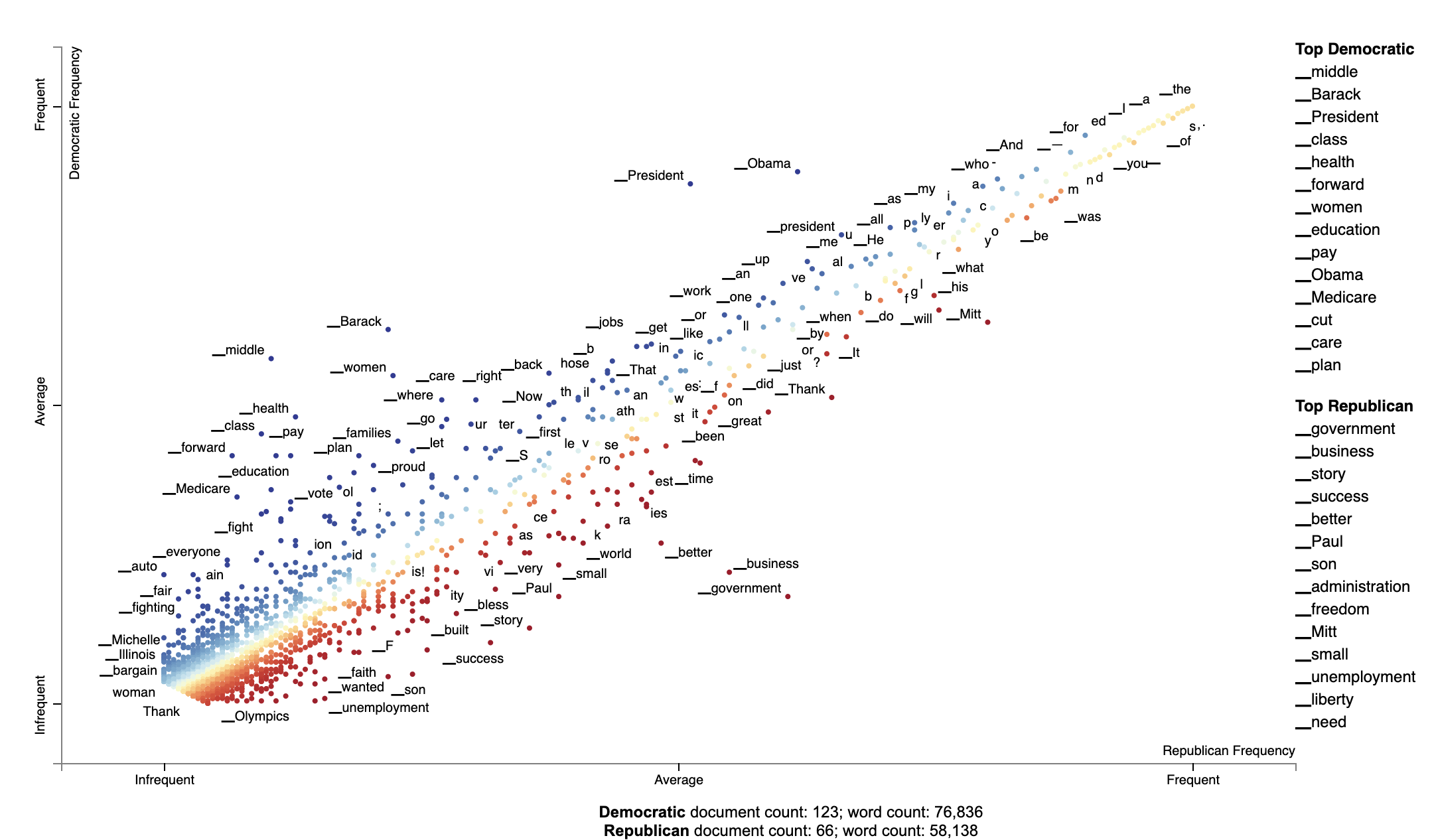### Visualizing scikit-learn text classification weights

Suppose you'd like to audit or better understand weights or importances given to bag-of-words features by a classifier.

It's easy to use Scattertext to do, if you use a Scikit-learn-style classifier.

For example the Lighting package makes available high-performance linear classifiers which are have Scikit-compatible interfaces.

First, let's import sklearn's text feature extraction classes, the 20 Newsgroup corpus, Lightning's Primal Coordinate Descent classifier, and Scattertext. We'll also fetch the training portion of the Newsgroup corpus.

from lightning.classification import CDClassifier
from sklearn.datasets import fetch_20newsgroups
from sklearn.feature_extraction.text import CountVectorizer, TfidfVectorizer

import scattertext as st

newsgroups_train = fetch_20newsgroups(
subset='train',
)


Next, we'll tokenize our corpus twice. Once into tfidf features which will be used to train the classifier, an another time into ngram counts that will be used by Scattertext. It's important that both vectorizers share the same vocabulary, since we'll need to apply the weight vector from the model onto our Scattertext Corpus.

vectorizer = TfidfVectorizer()
tfidf_X = vectorizer.fit_transform(newsgroups_train.data)
count_vectorizer = CountVectorizer(vocabulary=vectorizer.vocabulary_)


Next, we use the CorpusFromScikit factory to build a Scattertext Corpus object. Ensure the X parameter is a document-by-feature matrix. The argument to the y parameter is an array of class labels. Each label is an integer representing a different news group. We the feature_vocabulary is the vocabulary used by the vectorizers. The category_names are a list of the 20 newsgroup names which as a class-label list. The raw_texts is a list of the text of newsgroup texts.

corpus = st.CorpusFromScikit(
X=count_vectorizer.fit_transform(newsgroups_train.data),
y=newsgroups_train.target,
feature_vocabulary=vectorizer.vocabulary_,
category_names=newsgroups_train.target_names,
raw_texts=newsgroups_train.data
).build()


Now, we can train the model on tfidf_X and the categoricla response variable, and capture feature weights for category 0 ("alt.atheism").

clf = CDClassifier(penalty="l1/l2",
loss="squared_hinge",
multiclass=True,
max_iter=20,
alpha=1e-4,
C=1.0 / tfidf_X.shape,
tol=1e-3)
clf.fit(tfidf_X, newsgroups_train.target)
term_scores = clf.coef_


Finally, we can create a Scattertext plot. We'll use the Monroe-style visualization, and automatically select around 4000 terms that encompass the set of frequent terms, terms with high absolute scores, and terms that are characteristic of the corpus.

html = st.produce_frequency_explorer(
corpus,
'alt.atheism',
scores=term_scores,
use_term_significance=False,
terms_to_include=st.AutoTermSelector.get_selected_terms(corpus, term_scores, 4000),
metadata = ['/'.join(fn.split('/')[-2:]) for fn in newsgroups_train.filenames]
)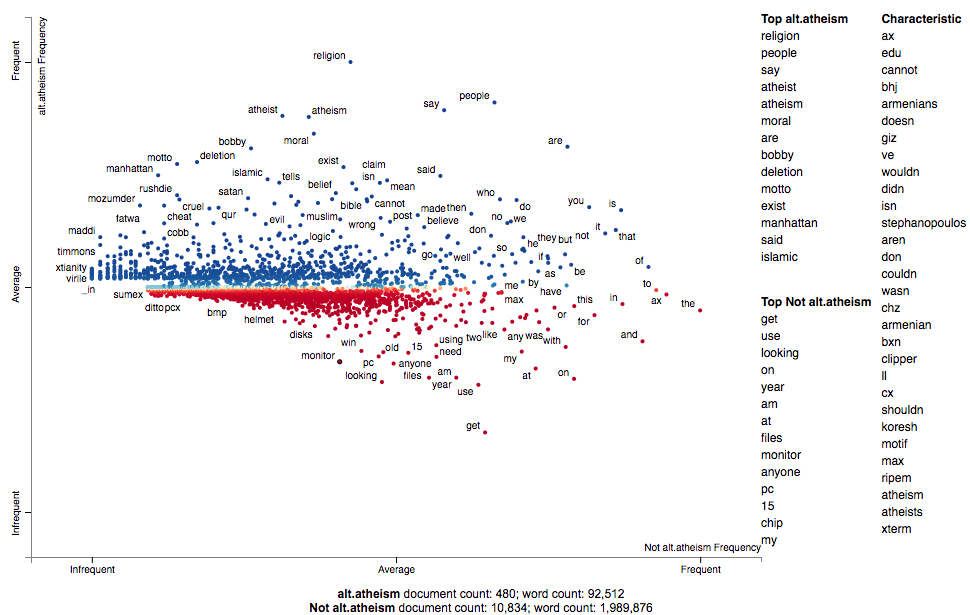Let's take a look at the performance of the classifier:

newsgroups_test = fetch_20newsgroups(subset='test',
X_test = vectorizer.transform(newsgroups_test.data)
pred = clf.predict(X_test)
f1 = f1_score(pred, newsgroups_test.target, average='micro')
print("Microaveraged F1 score", f1)


Microaveraged F1 score 0.662108337759. Not bad over a ~0.05 baseline.

### Creating lexicalized semiotic squares

Please see Signo for an introduction to semiotic squares.

Some variants of the semiotic square-creator are can be seen in this notebook, which studies words and phrases in headlines that had low or high Facebook engagement and were published by either BuzzFeed or the New York Times: [http://nbviewer.jupyter.org/github/JasonKessler/PuPPyTalk/blob/master/notebooks/Explore-Headlines.ipynb]

The idea behind the semiotic square is to express the relationship between two opposing concepts and concepts things within a larger domain of a discourse. Examples of opposed concepts life or death, male or female, or, in our example, positive or negative sentiment. Semiotics squares are comprised of four "corners": the upper two corners are the opposing concepts, while the bottom corners are the negation of the concepts.

Circumscribing the negation of a concept involves finding everything in the domain of discourse that isn't associated with the concept. For example, in the life-death opposition, one can consider the universe of discourse to be all animate beings, real and hypothetical. The not-alive category will cover dead things, but also hypothetical entities like fictional characters or sentient AIs.

In building lexicalized semiotic squares, we consider concepts to be documents labeled in a corpus. Documents, in this setting, can belong to one of three categories: two labels corresponding to the opposing concepts, a neutral category, indicating a document is in the same domain as the opposition, but cannot fall into one of opposing categories.

In the example below positive and negative movie reviews are treated as the opposing categories, while plot descriptions of the same movies are treated as the neutral category.

Terms associated with one of the two opposing categories (relative only to the other) are listed as being associated with that category. Terms associated with a netural category (e.g., not positive) are terms which are associated with the disjunction of the opposite category and the neutral category. For example, not-positive terms are those most associated with the set of negative reviews and plot descriptions vs. positive reviews.

Common terms among adjacent corners of the square are also listed.

An HTML-rendered square is accompanied by a scatter plot. Points on the plot are terms. The x-axis is the Z-score of the association to one of the opposed concepts. The y-axis is the Z-score how associated a term is with the neutral set of documents relative to the opposed set. A point's red-blue color indicate the term's opposed-association, while the more desaturated a term is, the more it is associated with the neutral set of documents.

import scattertext as st
movie_df = st.SampleCorpora.RottenTomatoes.get_data()
movie_df.category = movie_df.category.apply\
(lambda x: {'rotten': 'Negative', 'fresh': 'Positive', 'plot': 'Plot'}[x])
corpus = st.CorpusFromPandas(
movie_df,
category_col='category',
text_col='text',
nlp=st.whitespace_nlp_with_sentences
).build().get_unigram_corpus()

semiotic_square = st.SemioticSquare(
corpus,
category_a='Positive',
category_b='Negative',
neutral_categories=['Plot'],
scorer=st.RankDifference(),
labels={'not_a_and_not_b': 'Plot Descriptions', 'a_and_b': 'Reviews'}
)

html = st.produce_semiotic_square_explorer(semiotic_square,
category_name='Positive',
not_category_name='Negative',
x_label='Fresh-Rotten',
y_label='Plot-Review',
neutral_category_name='Plot Description',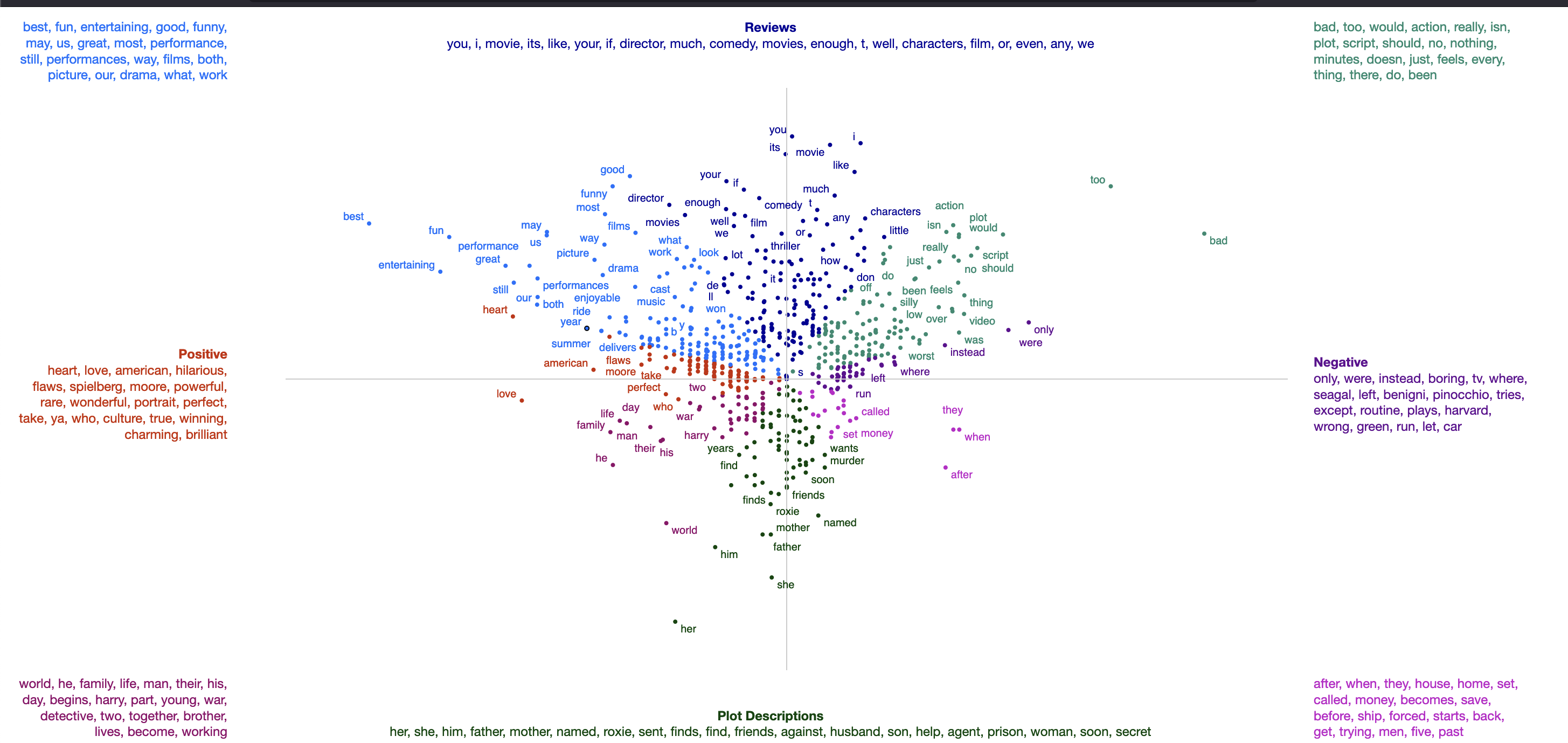There are a number of other types of semiotic square construction functions.

### Visualizing Topic Models

A frequently requested feature of Scattertext has been the ability to visualize topic models. While this capability has existed in some forms (e.g., the Empath visualization), I've finally gotten around to implementing a concise API for such a visualization. There are three main ways to visualize topic models using Scattertext. The first is the simplest: manually entering topic models and visualizing them. The second uses a Scikit-Learn pipeline to produce the topic models for visualization. The third is a novel topic modeling technique, based on finding terms similar to a custom set of seed terms.

#### Manually entered topic models

If you have already created a topic model, simply structure it as a dictionary. This dictionary is keyed on string which serve as topic titles and are displayed in the main scatterplot. The values are lists of words that belong to that topic. The words that are in each topic list are bolded when they appear in a snippet.

Note that currently, there is no support for keyword scores.

For example, one might manually the following topic models to explore in the Convention corpus:

topic_model = {
'money': ['money','bank','banks','finances','financial','loan','dollars','income'],
'jobs':['jobs','workers','labor','employment','worker','employee','job'],
'patriotic':['america','country','flag','americans','patriotism','patriotic'],
}


We can use the FeatsFromTopicModel class to transform this topic model into one which can be visualized using Scattertext. This is used just like any other feature builder, and we pass the topic model object into produce_scattertext_explorer.

import scattertext as st

topic_feature_builder = st.FeatsFromTopicModel(topic_model)

topic_corpus = st.CorpusFromParsedDocuments(
convention_df,
category_col='party',
parsed_col='parse',
feats_from_spacy_doc=topic_feature_builder
).build()

html = st.produce_scattertext_explorer(
topic_corpus,
category='democrat',
category_name='Democratic',
not_category_name='Republican',
width_in_pixels=1000,
use_non_text_features=True,
use_full_doc=True,
pmi_threshold_coefficient=0,
topic_model_term_lists=topic_feature_builder.get_top_model_term_lists()
)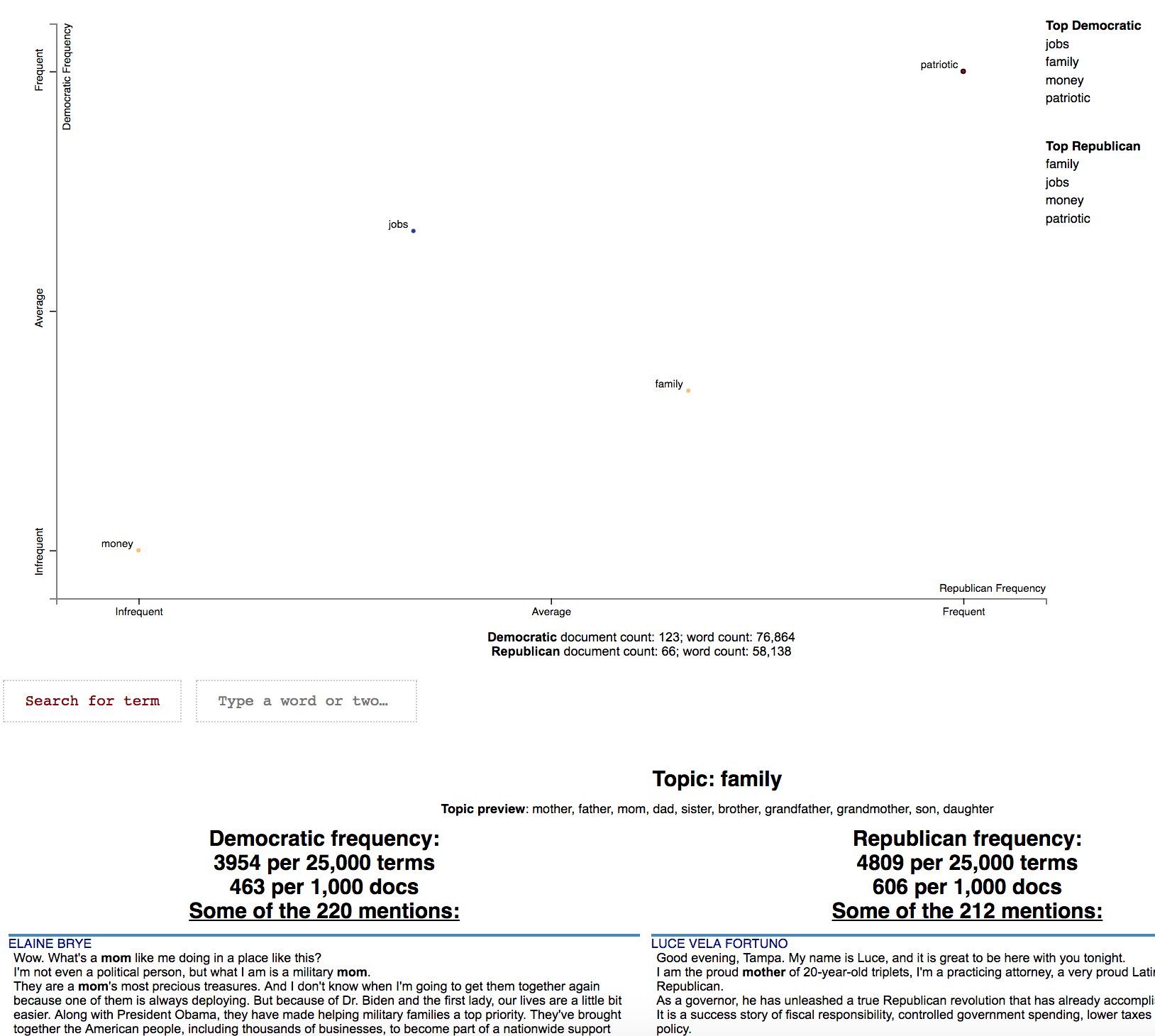#### Using Scikit-Learn for Topic Modeling

Since topic modeling using document-level coocurence generally produces poor results, I've added a SentencesForTopicModeling class which allows clusterting by coocurence at the sentence-level. It requires a ParsedCorpus object to be passed to its constructor, and creates a term-sentence matrix internally.

Next, you can create a topic model dictionary like the one above by passing in a Scikit-Learn clustering or dimensionality reduction pipeline. The only constraint is the last transformer in the pipeline must populate a components_ attribute.

The num_topics_per_term attribute specifies how many terms should be added to a list.

In the following example, we'll use NMF to cluster a stoplisted, unigram corpus of documents, and use the topic model dictionary to create a FeatsFromTopicModel, just like before.

Note that in produce_scattertext_explorer, we make the topic_model_preview_size 20 in order to show a preview of the first 20 terms in the topic in the snippet view as opposed to the default 10.

from sklearn.decomposition import NMF
from sklearn.feature_extraction.text import TfidfTransformer
from sklearn.pipeline import Pipeline

convention_df = st.SampleCorpora.ConventionData2012.get_data()
convention_df['parse'] = convention_df['text'].apply(st.whitespace_nlp_with_sentences)

unigram_corpus = (st.CorpusFromParsedDocuments(convention_df,
category_col='party',
parsed_col='parse')
.build().get_stoplisted_unigram_corpus())
topic_model = st.SentencesForTopicModeling(unigram_corpus).get_topics_from_model(
Pipeline([
('tfidf', TfidfTransformer(sublinear_tf=True)),
('nmf', (NMF(n_components=100, alpha=.1, l1_ratio=.5, random_state=0)))
]),
num_terms_per_topic=20
)

topic_feature_builder = st.FeatsFromTopicModel(topic_model)

topic_corpus = st.CorpusFromParsedDocuments(
convention_df,
category_col='party',
parsed_col='parse',
feats_from_spacy_doc=topic_feature_builder
).build()

html = st.produce_scattertext_explorer(
topic_corpus,
category='democrat',
category_name='Democratic',
not_category_name='Republican',
width_in_pixels=1000,
use_non_text_features=True,
use_full_doc=True,
pmi_threshold_coefficient=0,
topic_model_term_lists=topic_feature_builder.get_top_model_term_lists(),
topic_model_preview_size=20
)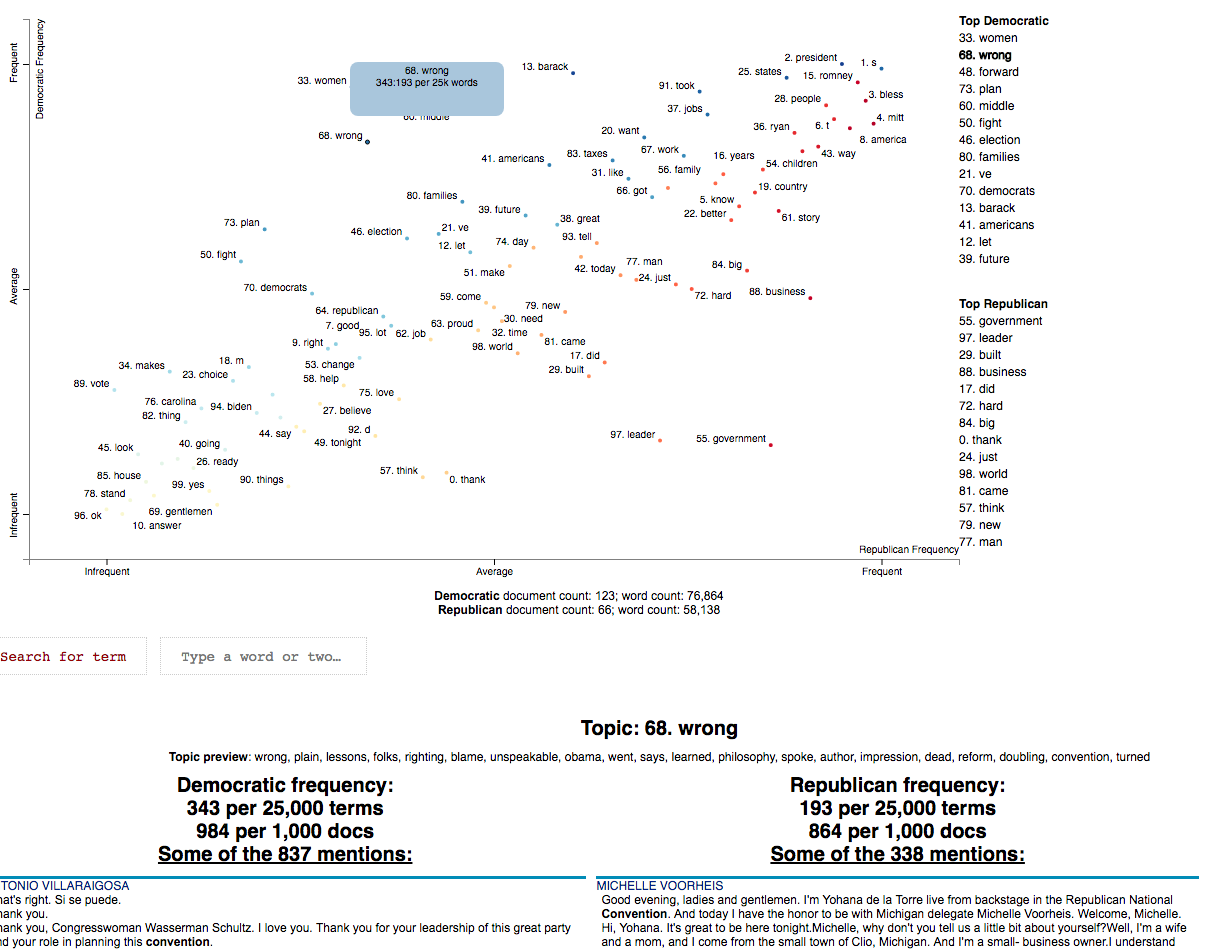#### Using a Word List to Generate a Series of Topics

A surprisingly easy way to generate good topic models is to use a term scoring formula to find words that are associated with sentences where a seed word occurs vs. where one doesn't occur.

Given a custom term list, the SentencesForTopicModeling.get_topics_from_terms will generate a series of topics. Note that the dense rank difference (RankDifference) works particularly well for this task, and is the default parameter.

term_list = ['obama', 'romney', 'democrats', 'republicans', 'health', 'military', 'taxes',
'education', 'olympics', 'auto', 'iraq', 'iran', 'israel']

unigram_corpus = (st.CorpusFromParsedDocuments(convention_df,
category_col='party',
parsed_col='parse')
.build().get_stoplisted_unigram_corpus())

topic_model = (st.SentencesForTopicModeling(unigram_corpus)
.get_topics_from_terms(term_list,
scorer=st.RankDifference(),
num_terms_per_topic=20))

topic_feature_builder = st.FeatsFromTopicModel(topic_model)
# The remaining code is identical to two examples above. See demo_word_list_topic_model.py
# for the complete example.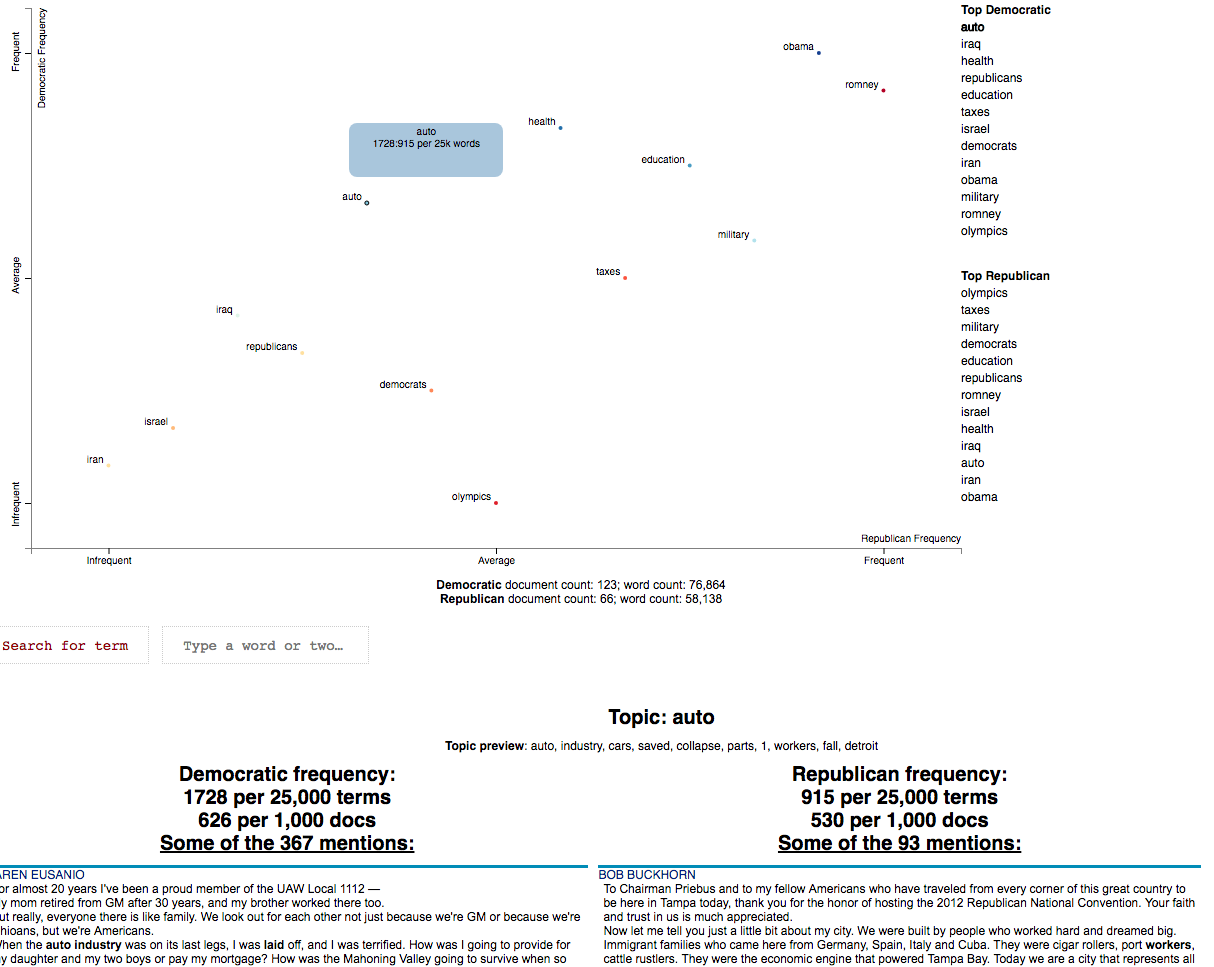### Creating T-SNE-style word embedding projection plots

Scattertext makes it easy to create word-similarity plots using projections of word embeddings as the x and y-axes. In the example below, we create a stop-listed Corpus with only unigram terms. The produce_projection_explorer function by uses Gensim to create word embeddings and then projects them to two dimentions using Uniform Manifold Approximation and Projection (UMAP).

UMAP is chosen over T-SNE because it can employ the cosine similarity between two word vectors instead of just the euclidean distance.

convention_df = st.SampleCorpora.ConventionData2012.get_data()
convention_df['parse'] = convention_df['text'].apply(st.whitespace_nlp_with_sentences)

corpus = (st.CorpusFromParsedDocuments(convention_df, category_col='party', parsed_col='parse')
.build().get_stoplisted_unigram_corpus())

html = st.produce_projection_explorer(corpus, category='democrat', category_name='Democratic',


In order to use custom word embedding functions or projection functions, pass models into the word2vec_model and projection_model parameters. In order to use T-SNE, for example, use projection_model=sklearn.manifold.TSNE().

import umap
from gensim.models.word2vec import Word2Vec

html = st.produce_projection_explorer(corpus,
word2vec_model=Word2Vec(size=100, window=5, min_count=10, workers=4),
projection_model=umap.UMAP(min_dist=0.5, metric='cosine'),
category='democrat',
category_name='Democratic',
not_category_name='Republican',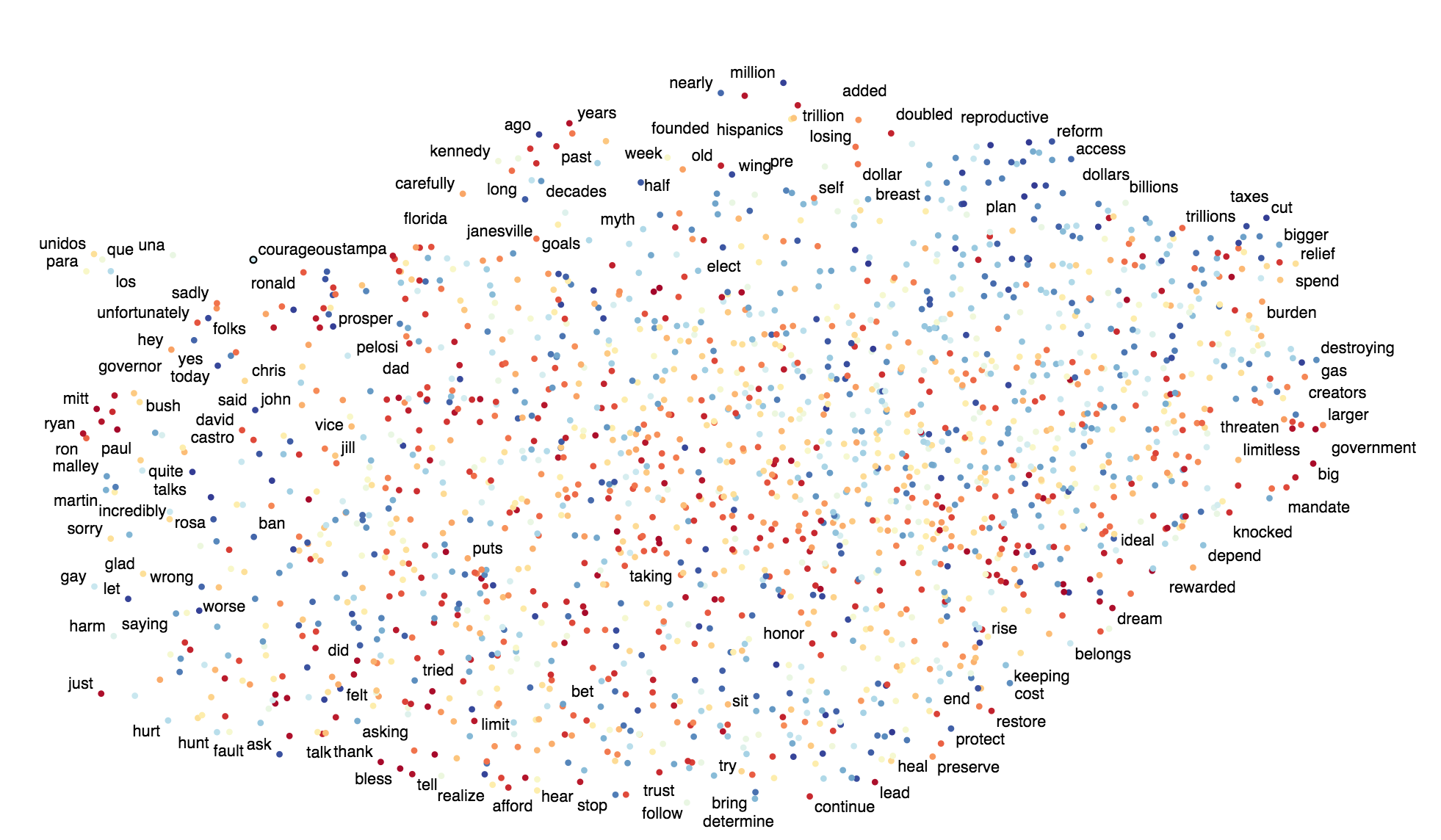### Using SVD to visualize any kind of word embeddings

Term positions can also be determined by the positions of terms according to the output of principal component analysis, and produce_projection_explorer also supports this functionality. We'll look at how axes transformations ("scalers" in Scattertext terminology) can make it easier to inspect the output of PCA.

We'll use the 2012 Conventions corpus for these visualizations. Only unigrams occurring in at least three documents will be considered.

>>> convention_df = st.SampleCorpora.ConventionData2012.get_data()
>>> convention_df['parse'] = convention_df['text'].apply(st.whitespace_nlp_with_sentences)
>>> corpus = (st.CorpusFromParsedDocuments(convention_df,
...                                        category_col='party',
...                                        parsed_col='parse')
...           .build()
...           .get_stoplisted_unigram_corpus()
...           .remove_infrequent_words(minimum_term_count=3, term_ranker=st.OncePerDocFrequencyRanker))


Next, we use scikit-learn's tf-idf transformer to find very simple, sparse embeddings for all of these words. Since, we input a #docs x #terms matrix to the transformer, we can transpose it to get a proper term-embeddings matrix, where each row corresponds to a term, and the columns correspond to document-specific tf-idf scores.

>>> from sklearn.feature_extraction.text import TfidfTransformer
>>> embeddings = TfidfTransformer().fit_transform(corpus.get_term_doc_mat())
>>> embeddings.shape
(189, 2159)
>>> corpus.get_num_docs(), corpus.get_num_terms()
(189, 2159)
>>> embeddings = embeddings.T
>>> embeddings.shape
(2159, 189)


Given these spare embeddings, we can apply sparse singular value decomposition to extract three factors. SVD outputs factorizes the term embeddings matrix into three matrices, U, Σ, and VT. Importantly, the matrix U provides the singular values for each term, and VT provides them for each document, and Σ is a vector of the singular values.

>>> from scipy.sparse.linalg import svds
>>> U, S, VT = svds(embeddings, k = 3, maxiter=20000, which='LM')
>>> U.shape
(2159, 3)
>>> S.shape
(3,)
>>> VT.shape
(3, 189)


We'll look at the first two singular values, plotting each term such that the x-axis position is the first singular value, and the y-axis term is the second. To do this, we make a "projection" data frame, where the x and y columns store the first two singular values, and key the data frame on each term. This controls the term positions on the chart.

>>> x_dim = 0; y_dim = 1;
>>> projection = pd.DataFrame({'term':corpus.get_terms(),
...                            'x':U.T[x_dim],
...                            'y':U.T[y_dim]}).set_index('term')


We'll use the produce_pca_explorer function to visualize these. Note we include the projection object, and specify which singular values were used for x and y (x_dim and y_dim) so we they can be labeled in the interactive visualization.

html = st.produce_pca_explorer(corpus,
category='democrat',
category_name='Democratic',
not_category_name='Republican',
projection=projection,
width_in_pixels=1000,
x_dim=x_dim,
y_dim=y_dim)


Click for an interactive visualization.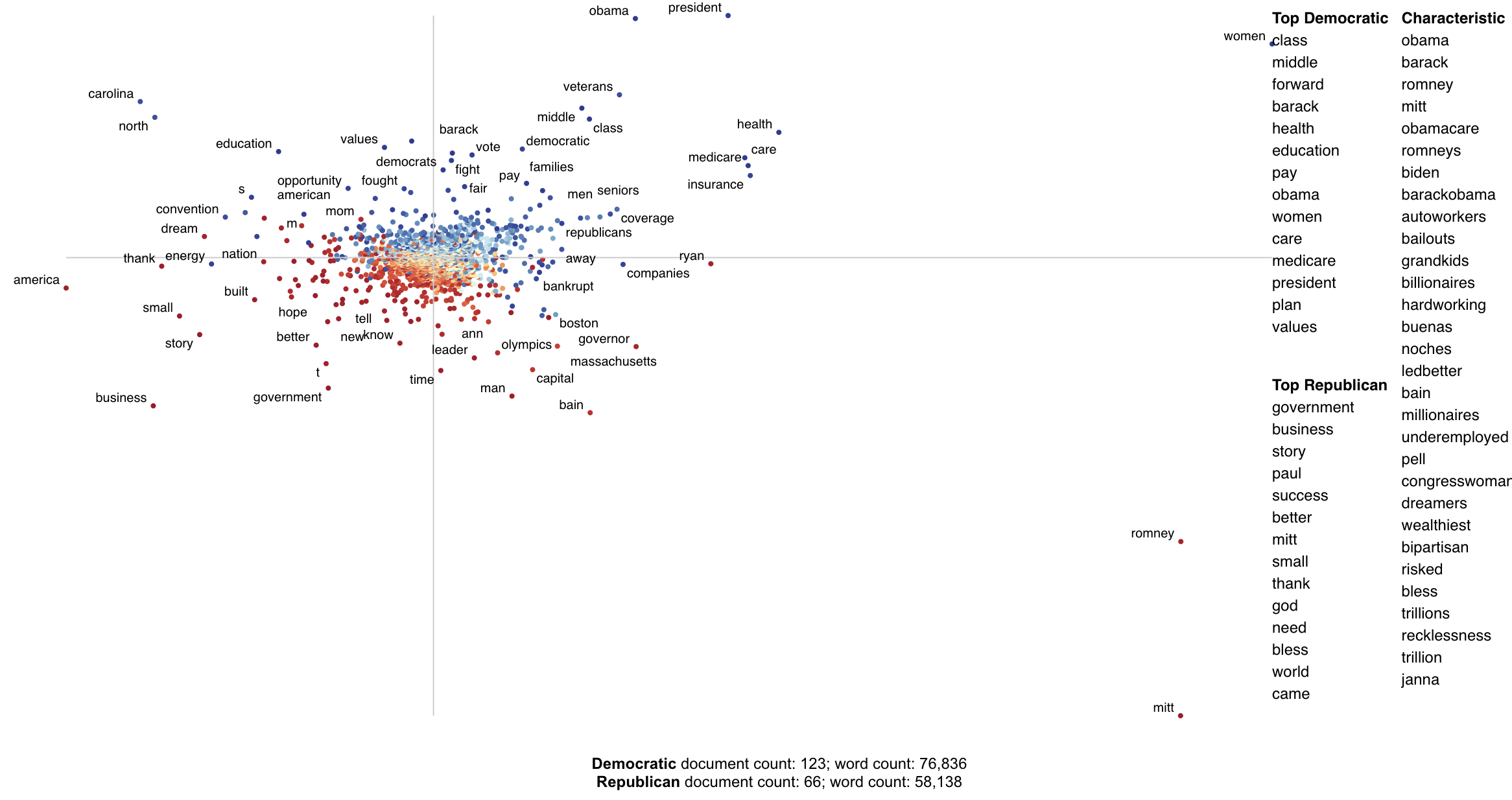We can easily re-scale the plot in order to make more efficient use of space. For example, passing in scaler=scale_neg_1_to_1_with_zero_mean will make all four quadrants take equal area.

html = st.produce_pca_explorer(corpus,
category='democrat',
category_name='Democratic',
not_category_name='Republican',
projection=projection,
width_in_pixels=1000,
scaler=st.scale_neg_1_to_1_with_zero_mean,
x_dim=x_dim,
y_dim=y_dim)


Click for an interactive visualization.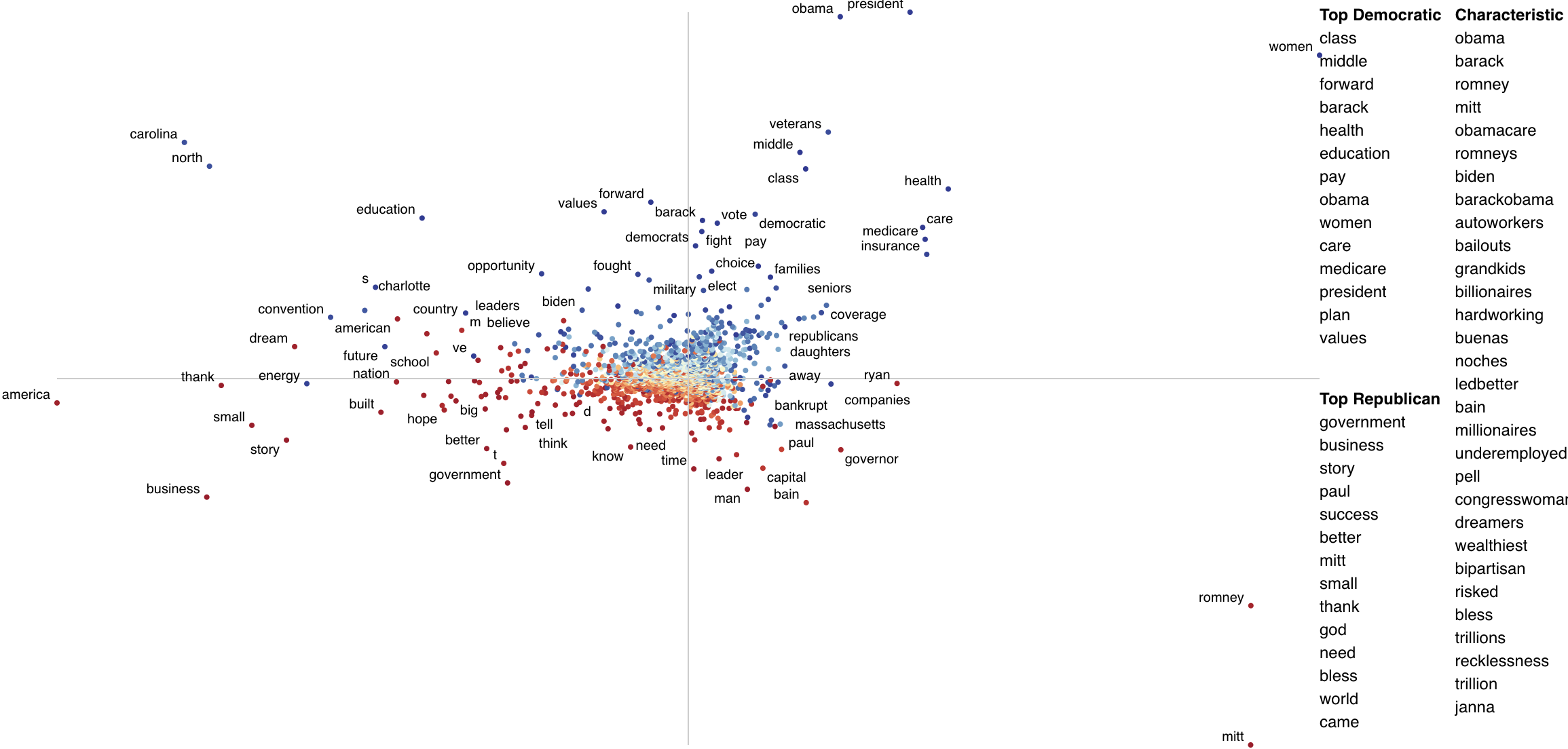### Exporting plot to matplotlib

To export the content of a scattertext explorer object (ScattertextStructure) to matplotlib you can use produce_scattertext_pyplot. The function returns a matplotlib.figure.Figure object which can be visualized using plt.show or plt.savefig as in the example below.

Note that installation of textalloc==0.0.3 and matplotlib>=3.6.0 is required before running this.

convention_df = st.SampleCorpora.ConventionData2012.get_data().assign(
parse = lambda df: df.text.apply(st.whitespace_nlp_with_sentences)
)
corpus = st.CorpusFromParsedDocuments(convention_df, category_col='party', parsed_col='parse').build()
scattertext_structure = st.produce_scattertext_explorer(
corpus,
category='democrat',
category_name='Democratic',
not_category_name='Republican',
minimum_term_frequency=5,
pmi_threshold_coefficient=8,
width_in_pixels=1000,
return_scatterplot_structure=True,
)
fig = st.produce_scattertext_pyplot(scattertext_structure)
fig.savefig('pyplot_export.png', format='png')


[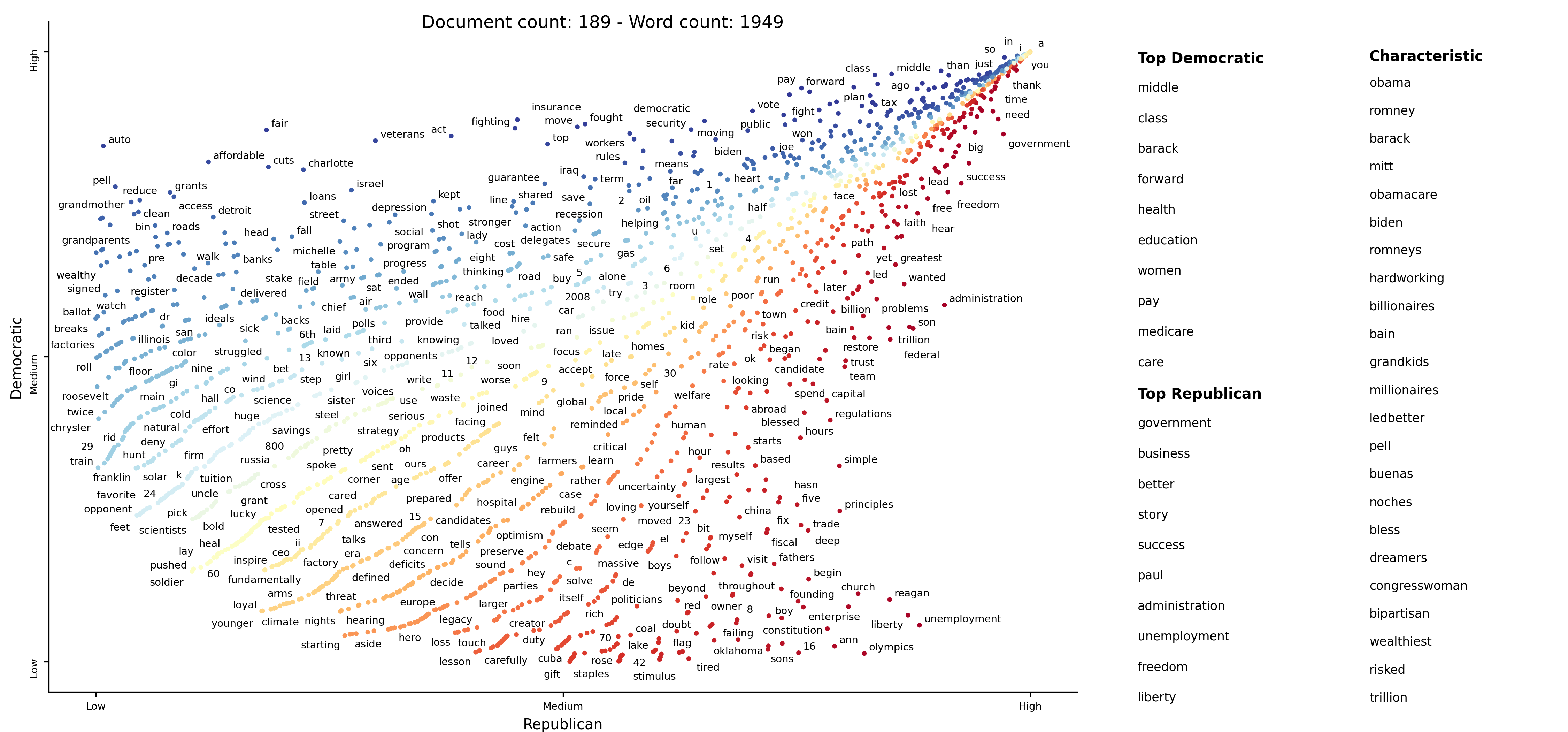]

## Examples

Please see the examples in the PyData 2017 Tutorial on Scattertext.

## A note on chart layout

Cozy: The Collection Synthesizer (Loncaric 2016) was used to help determine which terms could be labeled without overlapping a circle or another label. It automatically built a data structure to efficiently store and query the locations of each circle and labeled term.

The script to build rectangle-holder.js was

fields ax1 : long, ay1 : long, ax2 : long, ay2 : long
assume ax1 < ax2 and ay1 < ay2
query findMatchingRectangles(bx1 : long, by1 : long, bx2 : long, by2 : long)
assume bx1 < bx2 and by1 < by2
ax1 < bx2 and ax2 > bx1 and ay1 < by2 and ay2 > by1


And it was called using

### 0.0.2.8.0

Fixed bug in Scaled F-Score computations, and changed computation to better score words that are inversely correlated to category.

Added Word2VecFromParsedCorpus to automate training Gensim word vectors from a corpus, and
word_similarity_explorer_gensim to produce the visualization.

See demo_gensim_similarity.py for an example.

### 0.0.2.7.1

Added the d3_url and d3_scale_chromatic_url parameters to produce_scattertext_explorer. This provides a way to manually specify the paths to "d3.js" (i.e., the file from "https://cdnjs.cloudflare.com/ajax/libs/d3/4.6.0/d3.min.js") and "d3-scale-chromatic.v1.js" (i.e., the file from "https://d3js.org/d3-scale-chromatic.v1.min.js").

This is important if you're getting the error:

Javascript error adding output!
TypeError: d3.scaleLinear is not a function
See your browser Javascript console for more details.


It also lets you use Scattertext if you're serving in an environment with no (or a restricted) external Internet connection.

For example, if "d3.min.js" and "d3-scale-chromatic.v1.min.js" were present in the current working directory, calling the following code would reference them locally instead of the remote Javascript files. See Visualizing term associations for code context.

>>> html = st.produce_scattertext_explorer(corpus,
...          category='democrat',
...          category_name='Democratic',
...          not_category_name='Republican',
...          width_in_pixels=1000,
...          d3_url='d3.min.js',
...          d3_scale_chromatic_url='d3-scale-chromatic.v1.min.js')


### 0.0.2.7.0

Fixed a bug in 0.0.2.6.0 that transposed default axis labels.

Added a Japanese mode to Scattertext. See demo_japanese.py for an example of how to use Japanese. Please run pip install tinysegmenter to parse Japanese.

Also, the chiense_mode boolean parameter in produce_scattertext_explorer has been renamed to asian_mode.

For example, the output of demo_japanese.py is: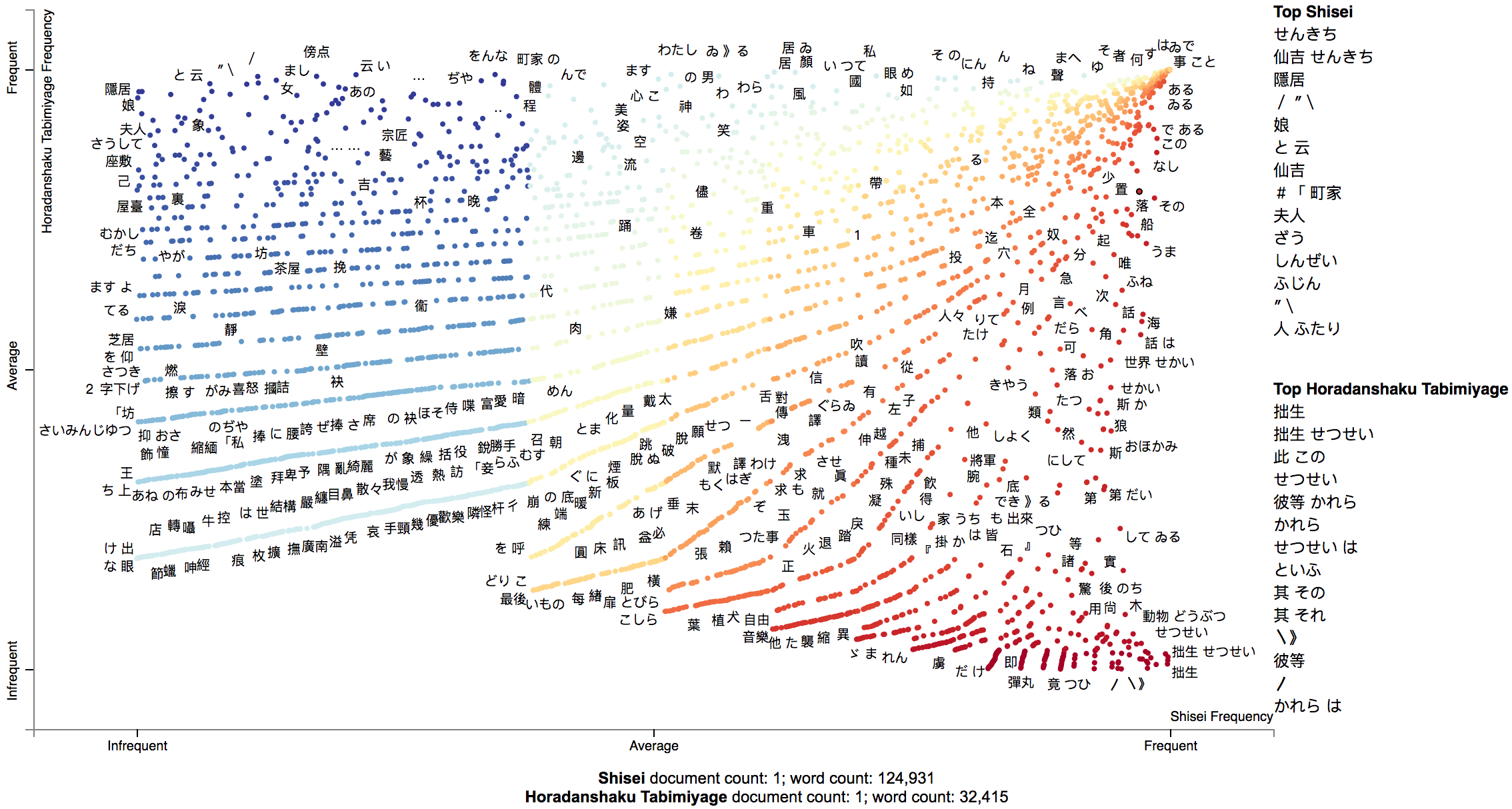### 0.0.2.6.0

Custom term positions and axis labels. Although not recommended, you can visualize different metrics on each axis in visualizations similar to Monroe et al. (2008). Please see Custom term positions for more info.

### 0.0.2.5.0

Enhanced the visualization of query-based categorical differences, a.k.a the word_similarity_explorer function. When run, a plot is produced that contains category associated terms colored in either red or blue hues, and terms not associated with either class colored in greyscale and slightly smaller. The intensity of each color indicates association with the query term. For example: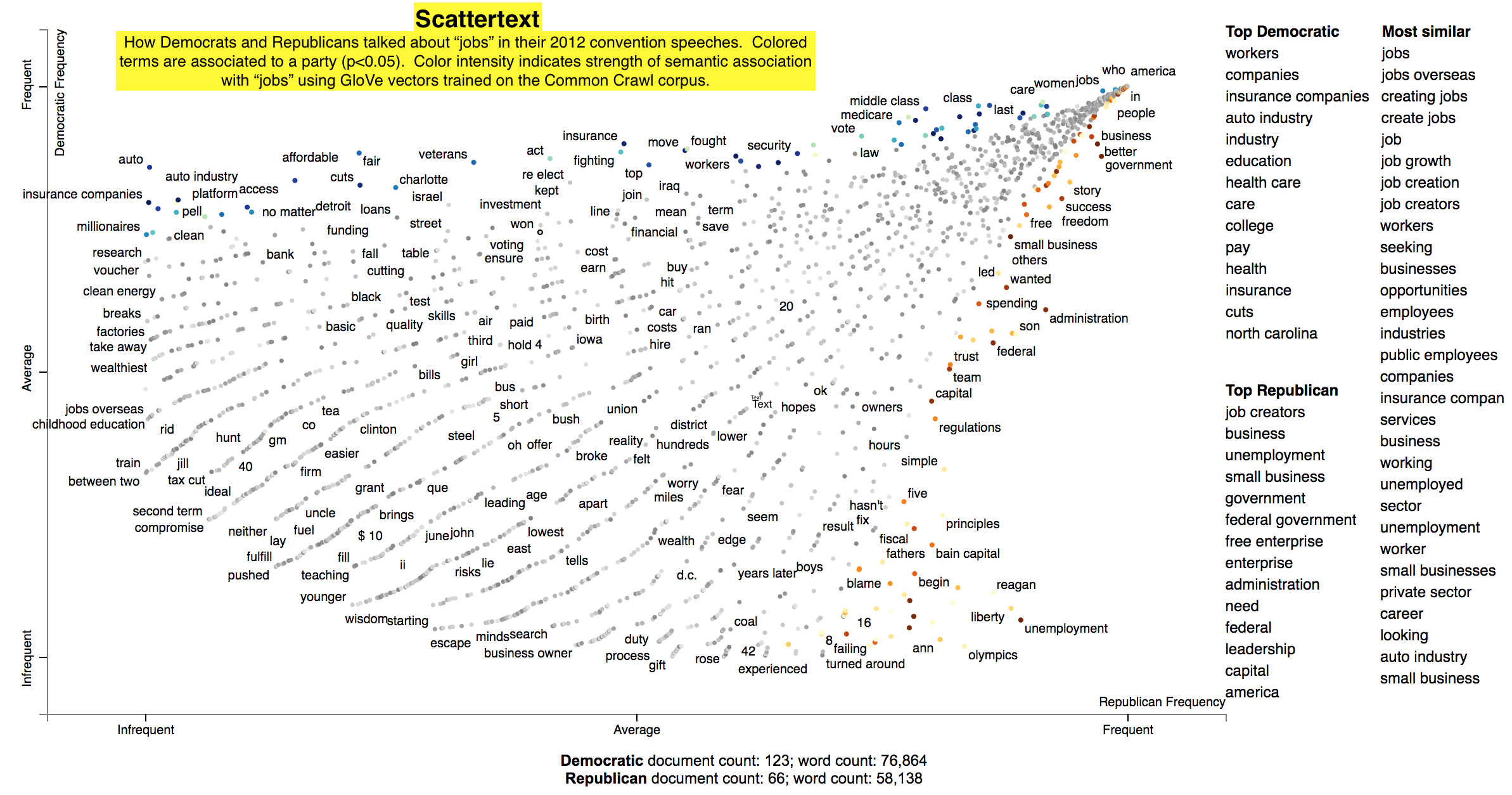### 0.0.2.4.6

Some minor bug fixes, and added a minimum_not_category_term_frequency parameter. This fixes a problem with visualizing imbalanced datasets. It sets a minimum number of times a word that does not appear in the target category must appear before it is displayed.

Added TermDocMatrix.remove_entity_tags method to remove entity type tags from the analysis.

### 0.0.2.4.5

Fixed matched snippet not displaying issue #9, and fixed a Python 2 issue in created a visualization using a ParsedCorpus prepared via CorpusFromParsedDocuments, mentioned in the latter part of the issue #8 discussion.

Again, Python 2 is supported in experimental mode only.

### 0.0.2.4.4

Fixed a bug in Issue 8 where the HTML visualization produced by produce_scattertext_html would fail.

### 0.0.2.4.2

Fixed a couple issues that rendered Scattertext broken in Python 2. Chinese processing still does not work.

Note: Use Python 3.4+ if you can.

### 0.0.2.4

Added the command line tool, and fixed a bug related to Empath visualizations.

### 0.0.2.3

Ability to see how a particular term is discussed differently between categories through the word_similarity_explorer function.

Specialized mode to view sparse term scores.

Fixed a bug that was caused by repeated values in background unigram counts.

Added true alphabetical term sorting in visualizations.

### 0.0.2.2

Addition option of showing characteristic terms (from the full set of documents) being considered. The option (show_characteristic in produce_scattertext_explorer) is on by default, but currently unavailable for Chinese. If you know of a good Chinese wordcount list, please let me know. The algorithm used to produce these is F-Score.
See this and the following slide for more details

### 0.0.2.1.5

Added document and word count statistics to main visualization.

### 0.0.2.1.3

Improved term-labeling.

### 0.0.2.1.1

Addition of strip_final_period param to FeatsFromSpacyDoc to deal with spaCy tokenization of all-caps documents that can leave periods at the end of terms.

### 0.0.2.1.0

I've added support for Chinese, including the ChineseNLP class, which uses a RegExp-based sentence splitter and Jieba for word segmentation. To use it, see the demo_chinese.py file. Note that CorpusFromPandas currently does not support ChineseNLP.

In order for the visualization to work, set the asian_mode flag to True in produce_scattertext_explorer`.

## Sources

• 2012 Convention Data: scraped from The New York Times.
• count_1w: Peter Norvig assembled this file (downloaded from norvig.com). See http://norvig.com/ngrams/ for an explanation of how it was gathered from a very large corpus.
• hamlet.txt: William Shakespeare. From shapespeare.mit.edu
• Inspiration for text scatter plots: Rudder, Christian. Dataclysm: Who We Are (When We Think No One's Looking). Random House Incorporated, 2014.
• Loncaric, Calvin. "Cozy: synthesizing collection data structures." Proceedings of the 2016 24th ACM SIGSOFT International Symposium on Foundations of Software Engineering. ACM, 2016.
• Fast, Ethan, Binbin Chen, and Michael S. Bernstein. "Empath: Understanding topic signals in large-scale text." Proceedings of the 2016 CHI Conference on Human Factors in Computing Systems. ACM, 2016.
• Burt L. Monroe, Michael P. Colaresi, and Kevin M. Quinn. 2008. Fightin’ words: Lexical feature selection and evaluation for identifying the content of political conflict. Political Analysis.
• Bo Pang and Lillian Lee. A Sentimental Education: Sentiment Analysis Using Subjectivity Summarization Based on Minimum Cuts, Proceedings of the ACL, 2004.
• Abram Handler, Matt Denny, Hanna Wallach, and Brendan O'Connor. Bag of what? Simple noun phrase extraction for corpus analysis. NLP+CSS Workshop at EMNLP 2016.
• Peter Fankhauser, Jörg Knappen, Elke Teich. Exploring and visualizing variation in language resources. LREC 2014.
• Shinichi Nakagawa and Innes C. Cuthill. Effect size, confidence interval and statistical significance: a practical guide for biologists. 2007. In Biological Reviews 82.
• Cynthia M. Whissell. The dictionary of affect in language. 1993. In The Measurement of Emotions.
• David Bamman, Jacob Eisenstein, and Tyler Schnoebelen. GENDER IDENTITY AND LEXICAL VARIATION IN SOCIAL MEDIA. 2014.
• Rada Mihalcea, Paul Tarau. TextRank: Bringing Order into Text. EMNLP. 2004.
• Frimer, J. A., Boghrati, R., Haidt, J., Graham, J., & Dehgani, M. Moral Foundations Dictionary for Linguistic Analyses 2.0. Unpublished manuscript. 2019.
• Jesse Graham, Jonathan Haidt, Sena Koleva, Matt Motyl, Ravi Iyer, Sean P Wojcik, and Peter H Ditto. 2013. Moral foundations theory: The pragmatic validity of moral pluralism. Advances in Experimental Social Psychology, 47, 55-130
• Ryan J. Gallagher, Morgan R. Frank, Lewis Mitchell, Aaron J. Schwartz, Andrew J. Reagan, Christopher M. Danforth, and Peter Sheridan Dodds. Generalized Word Shift Graphs: A Method for Visualizing and Explaining Pairwise Comparisons Between Texts. 2020. Arxiv. https://arxiv.org/pdf/2008.02250.pdf
• Kocoń, Jan; Zaśko-Zielińska, Monika and Miłkowski, Piotr, 2019, PolEmo 2.0 Sentiment Analysis Dataset for CoNLL, CLARIN-PL digital repository, http://hdl.handle.net/11321/710.
• George Forman. 2008. BNS feature scaling: an improved representation over tf-idf for svm text classification. In Proceedings of the 17th ACM conference on Information and knowledge management (CIKM '08). Association for Computing Machinery, New York, NY, USA, 263–270. https://doi.org/10.1145/1458082.1458119
• Anne-Kathrin Schumann. 2016. Brave new world: Uncovering topical dynamics in the ACL Anthology reference corpus using term life cycle information. In Proceedings of the 10th SIGHUM Workshop on Language Technology for Cultural Heritage, Social Sciences, and Humanities, pages 1–11, Berlin, Germany. Association for Computational Linguistics.
Popular Visualization Projects
Popular Machine Learning Projects
Popular User Interface Categories
Related Searches

Get A Weekly Email With Trending Projects For These Categories
No Spam. Unsubscribe easily at any time.
Python
Machine Learning
Natural Language Processing
Visualization
Plot
Sentiment
Japanese
D3
Word2vec
Eda
Word Embeddings
Text Mining
Exploratory Data Analysis
Topic Modeling